Typeco 别告诉别人你不开心 | 勤奋点做事 | 爱美食 | 爱健身 | 爱学习 2020-12-23T14:37:44.128Z http://typeco.cn/ Xu XingLing Hexo 设计模式之策略模式 http://typeco.cn/posts/99c8c8b1/ 2020-12-23T14:23:15.000Z 2020-12-23T14:37:44.128Z ]]> <link rel="stylesheet" class="aplayer-secondary-style-marker" href="/assets/css/APlayer.min.css"><script src="/assets/js/APlayer.min.js" cla 设计模式之简单工厂模式 http://typeco.cn/posts/eeb5bd44/ 2020-12-21T01:21:35.000Z 2020-12-30T01:57:40.368Z

## 概述

`简单工厂模式` 属于创建型模式，在简单工厂模式中用于被创建实例的方法通常为`静态方法`,又叫`静态工厂方法`

## 结构与业务场景

### 工厂（Factory）

`核心部分`，负责实现创建所有产品的内部逻辑，工厂类可以被外界直接调用，创建所需对象。

## 实际运用

• 首先，我们不考虑工厂类，工厂类我们放到最后去实现，我们先实现 `抽象产品类` 在我的项目里我叫它 `JCElementBaseView`，这是一个抽象产品类，用户不用初始化它，即使你初始化它也没有意义，因为它并没有什么实质的东西展示，它能做的就是对子类提供重写的方法和实现。
``@class JCElementBaseView;@protocol JCElementProtocol <NSObject>@optional- (void)elementViewTouchBegin:(JCElementBaseView *)element;- (void)elementViewDragBegin:(JCElementBaseView *)element;- (void)elementViewDoubleClick:(JCElementBaseView *)element;- (void)elementViewFrameShouldChange:(JCElementBaseView *)element;- (void)elementViewFrameDidChange:(JCElementBaseView *)element;- (void)elementViewDidClick:(JCElementBaseView *)element;@end@interface JCElementBaseView : UIView/** 旋转角度 */@property (nonatomic, assign) CGFloat rotate;/** 是否选中 */@property (nonatomic, assign) BOOL selected;/** 锁定 */@property (nonatomic, assign) BOOL isLock;/** 元素的id：时间戳，精确到毫秒 */@property (nonatomic, copy) NSString *elementId;/** 代理 */@property (nonatomic, weak) id<JCElementProtocol> delegate;/** for override */- (void)zoomDragMoving: (UIControl *) c withEvent:touches;- (void)zoomDragOutside: (UIControl *) c withEvent:touches;- (void)zoomDragEnded: (UIControl *) c withEvent:touches;/** 元素最小宽度---子类实现 毫米 */- (CGFloat)minWidth_mm;/** 元素最小高度---子类实现 毫米 */- (CGFloat)minHeight_mm;/** 元素最大宽度---子类实现 毫米 */- (CGFloat)maxWidth_mm;/** 元素最大高度---子类实现 毫米 */- (CGFloat)maxHeight_mm;/** 获取旋转角度为0时的frame */- (CGRect)getOrignalFrame;/** 刷新图像 */- (void)reloadSDKImage;@end``
• 接下来就是针对我们特定的元素的一些特定的实现一一去构建子类（`具体产品类`），这里我们以 `条码` 为例作为大概展示其子类是如何实现的,基本可以看出，都是以实现`抽象父类` 的方法为主。
``@implementation JCElementBarCode- (instancetype)init {    if (self = [super init]) {        self.backgroundColor = [UIColor clearColor];        [self initUI];    }    return self;}- (void)initUI {    [self insertSubview:self.barCodeImageView atIndex:0];    [self.barCodeImageView mas_remakeConstraints:^(MASConstraintMaker *make) {        make.size.equalTo(self);        make.top.left.equalTo(self);    }];    [self.barCodeImageView addSubview:self.errorButton];    [self.errorButton mas_makeConstraints:^(MASConstraintMaker *make) {        make.size.mas_equalTo((CGSize){116,24});        make.center.equalTo(self.barCodeImageView);    }];}- (void)reloadSDKImage {    NSString *value = [DrawBoardInfo currentValueFor:self.excelValue selectRowIndex:self.excelIndex elementId:self.elementId];    if (IsExcelString(self.excelValue)) {        _text = value;    }    // resize    CGFloat minWidth = [self minWidth_mm]*DrawBoardInfo.mm2pxScale;    rotate(           if (self.width < minWidth) {               self.width = minWidth;           }           )    [self drawBarCodeImage];}#pragma mark -- set- (void)setText:(NSString *)text {    _text = text;    /** 根据文本画一维码 */    [self reloadSDKImage];}- (void)setConfigure:(JCElementBarCodeConfigure *)configure {    _configure = configure;    /** 根据条码类型重新布局 */    [self drawBarCodeImage];}- (void)setExcelIndex:(NSInteger)excelIndex {    [super setExcelIndex:excelIndex];    [self reloadSDKImage];}#pragma mark -- getImage- (void)drawBarCodeImage {    if (!self.text || !self.configure) return;    [JCDrawCodeManager drawBarCodeWithElement:self result:^(BOOL success, UIImage * _Nonnull image, NSString * _Nonnull errorMsg) {        self.barCodeImageView.image  = image;        [self showErrorMsg:errorMsg];    }];}#pragma mark -  DragMove- (void)zoomDragMoving: (UIControl *) c withEvent:touches {    [super zoomDragMoving:c withEvent:touches];    [self drawBarCodeImage];}- (void)zoomDragOutside: (UIControl *) c withEvent:touches {    [super zoomDragOutside:c withEvent:touches];    [self drawBarCodeImage];}- (void)zoomDragEnded: (UIControl *) c withEvent:touches {    [super zoomDragEnded:c withEvent:touches];    [self drawBarCodeImage];}#pragma mark -- lazy- (UIImageView *)barCodeImageView {    if (!_barCodeImageView) {        _barCodeImageView = [[UIImageView alloc] init];        _barCodeImageView.contentMode = UIViewContentModeScaleToFill;        _barCodeImageView.backgroundColor = [UIColor clearColor];        // 最近邻居算法，图像放大不模糊        _barCodeImageView.layer.magnificationFilter = kCAFilterNearest;    }    return _barCodeImageView;}#pragma mark -- FrameProtocol- (CGFloat)minWidth_mm {    if (self.configure.codeType == JCCodeType_EAN13) {        return 13*2;    } else if (self.configure.codeType == JCCodeType_EAN8) {        return 7*2;    } else if (self.configure.codeType == JCCodeType_UPCA) {        return 11*2;    } else if (self.configure.codeType == JCCodeType_UPCE) {        return 7*2;    } else if (self.configure.codeType == JCCodeType_ITF25 || self.configure.codeType == JCCodeType_CODEBAR) {        return 2*2;    } else {        return MAX(self.text.length*2,2);    }}- (CGFloat)minHeight_mm {    return self.configure.textHeight + 3;}- (CGFloat)maxWidth_mm {    return 200;}- (CGFloat)maxHeight_mm {    return 100;}``
• 既然具体产品类都有了，剩下就是构建 `工厂类` ，这里我是用的一个单独的`Manager` 的类来实现元素的构建的 `JCDefaultElementManager`
``@implementation JCDefaultElementManager+ (JCElementBaseView *)defaultElementWithClass:(Class)eClass {    CGFloat mm2pxScale = DrawBoardInfo.mm2pxScale;    JCElementBaseView *element;    if ([eClass isEqual:[JCElementText class]]) {        JCElementText *temp = [JCElementText new];        temp.placeHolder = XY_LANGUAGE_TITLE_NAMED(@"",element_text_placeholder);        temp.text = @"";        temp.configure = [JCElementTextConfigure defaultConfigure];        temp.frame = (CGRect){10,10,4*DrawBoardInfo.mm2pxScale*text_default_font_mm_size,24};        element = temp;    } else if ([eClass isEqual:[JCElementTextImage class]]) {        JCElementTextImage *temp = [JCElementTextImage new];        temp.frame = (CGRect){10,10,4.2*DrawBoardInfo.mm2pxScale*text_default_font_mm_size,24};        temp.placeHolder = XY_LANGUAGE_TITLE_NAMED(@"",element_text_placeholder);        temp.text = XY_LANGUAGE_TITLE_NAMED(@"",element_text_placeholder);        temp.configure = [JCElementTextConfigure defaultConfigure];        element = temp;    } else if ([eClass isEqual:[JCElementBarCode class]]) {        JCElementBarCode *temp = [JCElementBarCode new];        temp.frame = (CGRect){10,10,20*DrawBoardInfo.mm2pxScale,10*DrawBoardInfo.mm2pxScale};        temp.configure = [JCElementBarCodeConfigure defaultConfigure];        temp.text = @"123456";        element = temp;    } else if ([eClass isEqual:[JCElementQRCode class]]) {        JCElementQRCode *temp = [JCElementQRCode new];        temp.frame = (CGRect){10,10,10*mm2pxScale,10*mm2pxScale};        temp.codeType = JCCodeType_QRCODE;        temp.qrCodeString = @"123456";        element = temp;    } else if ([eClass isEqual:[JCElementGraph class]]) {        JCElementGraph *temp = [JCElementGraph new];        temp.frame = (CGRect){10,10,10*DrawBoardInfo.mm2pxScale,10*DrawBoardInfo.mm2pxScale};        temp.configure = [JCEleBoxConfigure defaultConfigure];        element = temp;    } else if ([eClass isEqual:[JCElementSerialNumber class]]) {        JCElementSerialNumber *temp = [JCElementSerialNumber new];        temp.frame = (CGRect){10,10,145,58};        temp.styleConfigure = [JCSerialStyleConfigure defaultConfigure];        JCElementTextConfigure *configure = [JCElementTextConfigure defaultConfigure];        configure.textAlignment = NSTextAlignmentCenter;        temp.configure = configure;        element = temp;    } else if ([eClass isEqual:[JCElementTime class]]) {        JCElementTime *temp = [JCElementTime new];        temp.frame = (CGRect){10,10,200,29};        temp.timeConfigure = [JCEleTimeConfigure defaultConfigure];        temp.configure = [JCElementTextConfigure defaultConfigure];        element = temp;    } else if ([eClass isEqual:[JCElementSheet class]]) {        JCElementSheet *temp = [JCElementSheet new];        temp.configure = [JCSheetConfigure defaultConfigure];        temp.left = 10;        temp.top = 10;        element = temp;    } else if ([eClass isEqual:[JCElementLogoView class]]) {        JCElementLogoView *temp = [JCElementLogoView new];        element = temp;    } else if ([eClass isEqual:[JCElementLine class]]) {        JCElementLine *temp = [[JCElementLine alloc] init];        temp.frame = (CGRect){10,10,10*DrawBoardInfo.mm2pxScale,0.4*DrawBoardInfo.mm2pxScale};        temp.configure = [JCEleLineConfigure defaultConfigure];        element = temp;    }     NSInteger count = DrawBoardInfo.addElementIndex;    if (count > 8) count = 1;    if(count == 0){        element.left = 10;        element.top = 10;    }else{        element.left = 10 + count*16;        element.top = 10 + count*16;    }    /** 设置时间戳 */    element.elementId = [XYTool randomElementId];    DrawBoardInfo.addElementIndex++;    return element;}``

``-(void)add_wenben{    [self addElement:Element(JCElementTextImage)];}-(void)add_yiweima{    [self addElement:Element(JCElementBarCode)];}-(void)add_erweima{    [self addElement:Element(JCElementQRCode)];}-(void)add_liushuihao{    [self addElement:Element(JCElementSerialNumber)];}-(void)add_shijian{    [self addElement:Element(JCElementTime)];}-(void)add_xingzhuang{    [self addElement:Element(JCElementGraph)];}-(void)add_xiantiao{    [self addElement:Element(JCElementLine)];}``

## 简单工厂优缺点和适用环境

### 优点

• 用户只需要知道具体工厂的名称即可得到所要的产品，无需知道具体产品的创建过程。
• 工厂类包含必要的判断逻辑，可以决定在什么时候创建哪一个产品的实例，客户端可以免除直接创建产品对象的职责。（可以结合反射等思想，配置文件等等更加优化实现）
• 灵活性强，对于新产品的创建，只需多写一个相应的工厂类
• 典型的解耦框架，高层模块只需要知道产品的抽象类，无需关心其他实现类

### 缺点

• 类的个数容易过多，增加复杂度
• 增加了系统的抽象性和理解难度
• 抽象产品只能生产一种产品，此弊端可使用 `抽象工厂模式` 来解决

### 适用环境

• 工厂类负责创建对象比较少，因为这样不会造成工厂方法中的业务逻辑过于负责，比如上述的画板案例中，元素的种类就是那么多，不会无限制的进行扩张，在可预见范围内都是可以接受的。
• 客户端只知道传入工厂类的参数，对于如何创建对象并不关心，俗称只管拿去用。

## 总结

• 缩短开发时间
• 降低维护成本
• 在应用程序之间和内部轻松集成，减少耦合

]]>
OpenGL：正背面剔除、深度测试 http://typeco.cn/posts/4c2cf919/ 2020-07-13T14:27:05.000Z 2020-07-13T14:27:28.593Z ]]> <link rel="stylesheet" class="aplayer-secondary-style-marker" href="/assets/css/APlayer.min.css"><script src="/assets/js/APlayer.min.js" cla OpenGL：渲染之固定着色器 http://typeco.cn/posts/b5a93e1/ 2020-07-12T14:53:47.000Z 2020-07-13T14:22:39.657Z ]]> <link rel="stylesheet" class="aplayer-secondary-style-marker" href="/assets/css/APlayer.min.css"><script src="/assets/js/APlayer.min.js" cla OpenGL：渲染的基础 http://typeco.cn/posts/7f3a747e/ 2020-07-10T07:43:06.000Z 2020-07-13T16:12:36.972Z

## OpenGL渲染流程解析

• `attrubutes` 属性只可传给 `Vertex Shader(顶点着色器)`
• 通过`顶点着色器``Primitive(几何图元)` 传递 `Position``Outs`
• 可以通过 `OpenGl API` 向顶点着色器或者片元着色器
• `Texture Data(纹理数据)`也可以同时传递给顶点着色器和片元着色器，但是真正用它的是片元着色器。

### 正投影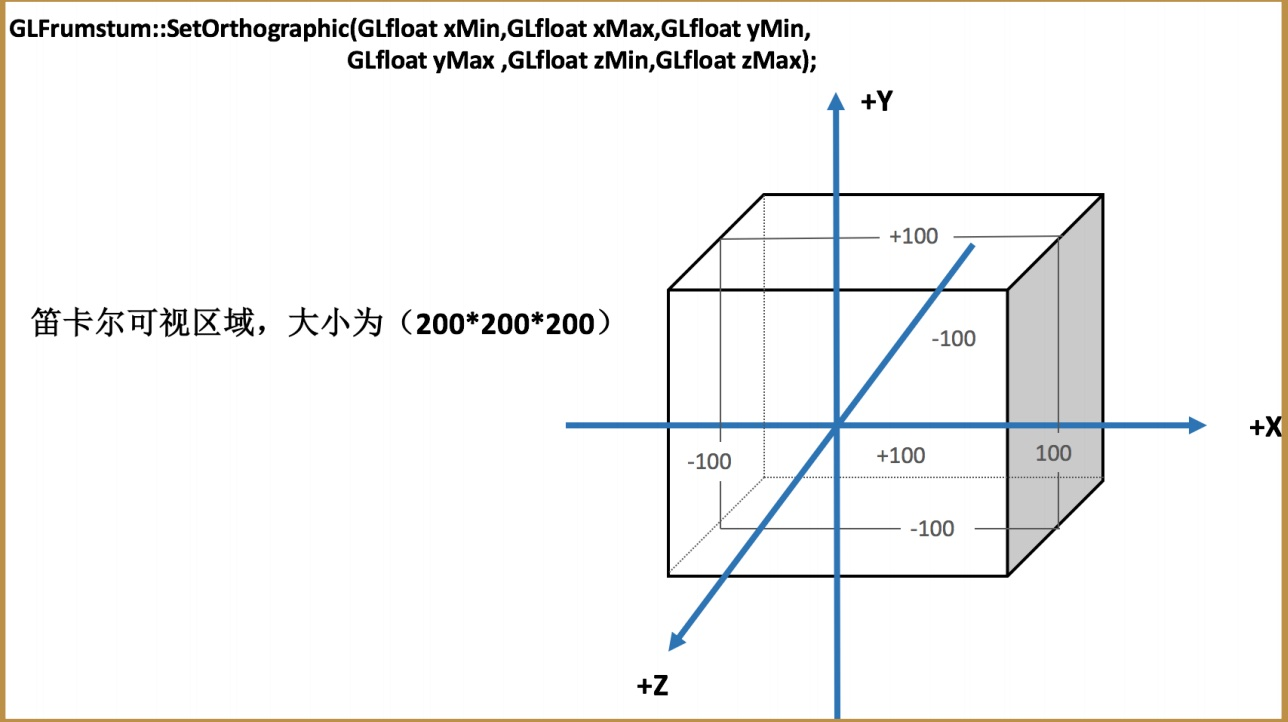### 透视投影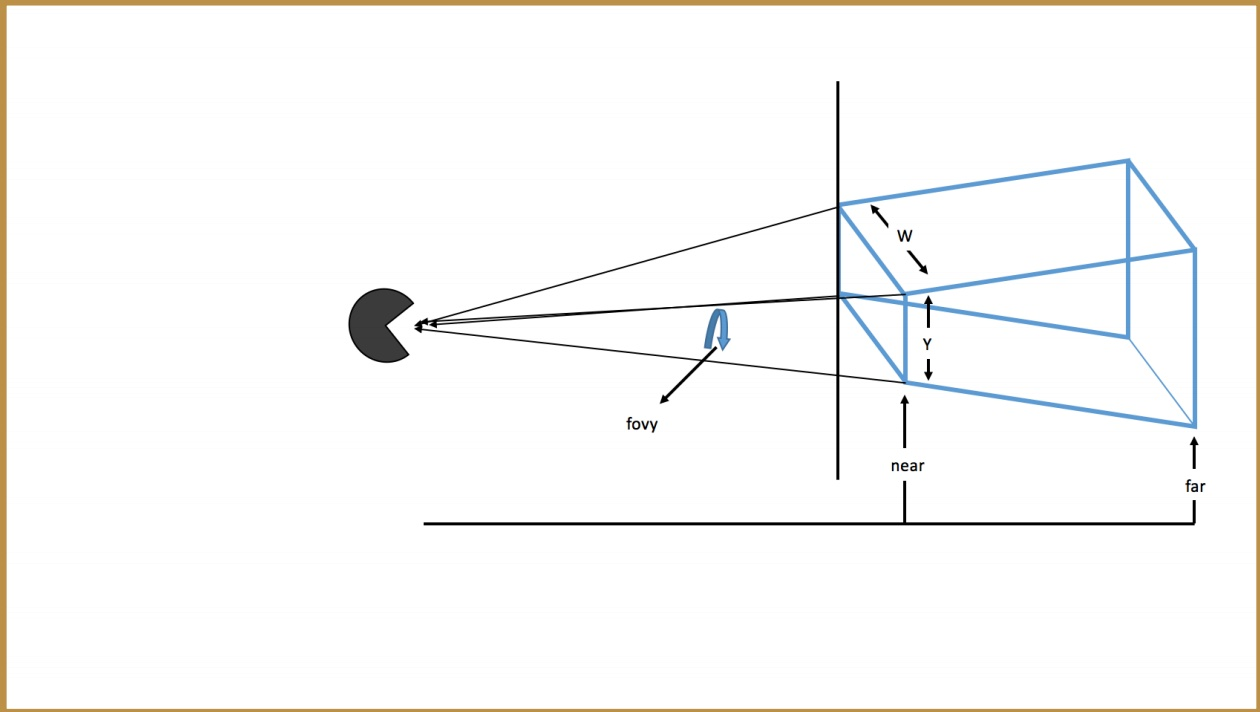`GLFrustum` 类通过`SetPerspective` 方法为我们构建一个平截头体。

`GLFrustum:SetPerspective(float fFov,float fAspect,float fNear,float fFar)`:

• `fFov`： 垂直方向上的视场角度

• `fAspect`:窗口的宽度与高度的纵横比

• `fNear`:近裁剪面距离

• `fFar`:远裁剪面距离

纵横比 = 宽（w）/高（h）

## OpenGL固定着色器

• 存储器初始化

``// 定义GLShaderManager        shaderManager;// 构造shaderManager.InitializeStockShaders();``
• 单元着色器

``GLShaderManager::UserStockShader(GLT_SHADER_IDENTITY,GLfloat vColor);参数1: 存储着⾊器种类-单元着⾊器参数2: 颜⾊使⽤场景: 绘制默认OpenGL 坐标系(-1,1)下图形. 图形所有⽚段都会以⼀种颜⾊填充 ``
• 平面着色器

``GLShaderManager::UserStockShader(GLT_SHADER_FLAT,GLfloat mvp,GLfloat vColor);参数1: 存储着⾊器种类-平⾯着⾊器参数2: 允许变化的4*4矩阵参数3: 颜⾊使⽤场景: 在绘制图形时, 可以应⽤变换(模型/投影变化).``
• 上色着色器

``GLShaderManager::UserStockShader(GLT_SHADER_SHADED,GLfloat mvp);参数1: 存储着⾊器种类-上⾊着⾊器参数2: 允许变化的4*4矩阵使⽤场景: 在绘制图形时, 可以应⽤变换(模型/投影变化) 颜⾊将会平滑地插⼊到顶点之间称为平滑着⾊. ``
• 默认光源着色器

``GLShaderManager::UserStockShader(GLT_SHADER_DEFAULT_LIGHT,GLfloat mvMatrix,GLfloat pMatrix,GLfloat vColor);参数1: 存储着⾊器种类-默认光源着⾊器参数2: 模型4*4矩阵参数3: 投影4*4矩阵参数4: 颜⾊值使⽤场景: 在绘制图形时, 可以应⽤变换(模型/投影变化) 这种着⾊器会使绘制的图形产⽣阴影和光照的效果.``
• 点光源着色器

``GLShaderManager::UserStockShader(GLT_SHADER_POINT_LIGHT_DIEF,GLfloat mvMatrix,GLfloat pMatrix,GLfloat vLightPos,GLfloat vColor); 参数1: 存储着⾊器种类-点光源着⾊器参数2: 模型4*4矩阵参数3: 投影4*4矩阵参数4: 点光源的位置参数5: 颜⾊值使⽤场景: 在绘制图形时, 可以应⽤变换(模型/投影变化) 这种着⾊器会使绘制的图形产⽣阴影和光照的效果.它与默认光源着⾊器⾮常类似,区别只是光源位置可能是特定的``
• 纹理替换矩阵着色器

``GLShaderManager::UserStockShader(GLT_SHADER_TEXTURE_REPLACE,GLfloat mvMatrix,GLint nTextureUnit);参数1: 存储着⾊器种类-纹理替换矩阵着⾊器参数2: 模型4*4矩阵参数3: 纹理单元使⽤场景: 在绘制图形时, 可以应⽤变换(模型/投影变化)这种着⾊器通过给定的模型视图投影矩阵.使⽤纹理单元来进⾏颜⾊填充.其中每个像素点的颜⾊是从纹理中获取.``
• 纹理调整着色器

``GLShaderManager::UserStockShader(GLT_SHADER_TEXTURE_MODULATE,GLfloat mvMatrix,GLfloat vColor,GLint nTextureUnit);参数1: 存储着⾊器种类-纹理调整着⾊器参数2: 模型4*4矩阵参数3: 颜⾊值参数4: 纹理单元使⽤场景: 在绘制图形时, 可以应⽤变换(模型/投影变化)这种着⾊器通过给定的模型视图投影矩阵. 着⾊器将⼀个基本⾊乘以⼀个取⾃纹理单元nTextureUnit 的纹理.将颜⾊与纹理进⾏颜⾊混合后才填充到⽚段中. ``
• 纹理光源着色器

``GLShaderManager::UserStockShader(GLT_SHADER_TEXTURE_POINT_LIGHT_DIEF,GLfloat mvMatrix,GLfloat pMatrix,GLfloat vLightPos,GLfloat vBaseColor,GLint nTextureUnit);参数1: 存储着⾊器种类-纹理光源着⾊器参数2: 模型4*4矩阵参数3: 投影4*4矩阵参数4: 点光源位置参数5: 颜⾊值(⼏何图形的基本⾊) 参数6: 纹理单元使⽤场景: 在绘制图形时, 可以应⽤变换(模型/投影变化)这种着⾊器通过给定的模型视图投影矩阵. 着⾊器将⼀个纹理通过漫反射照明计算进⾏调整(相乘). ``

## OpenGL基本图元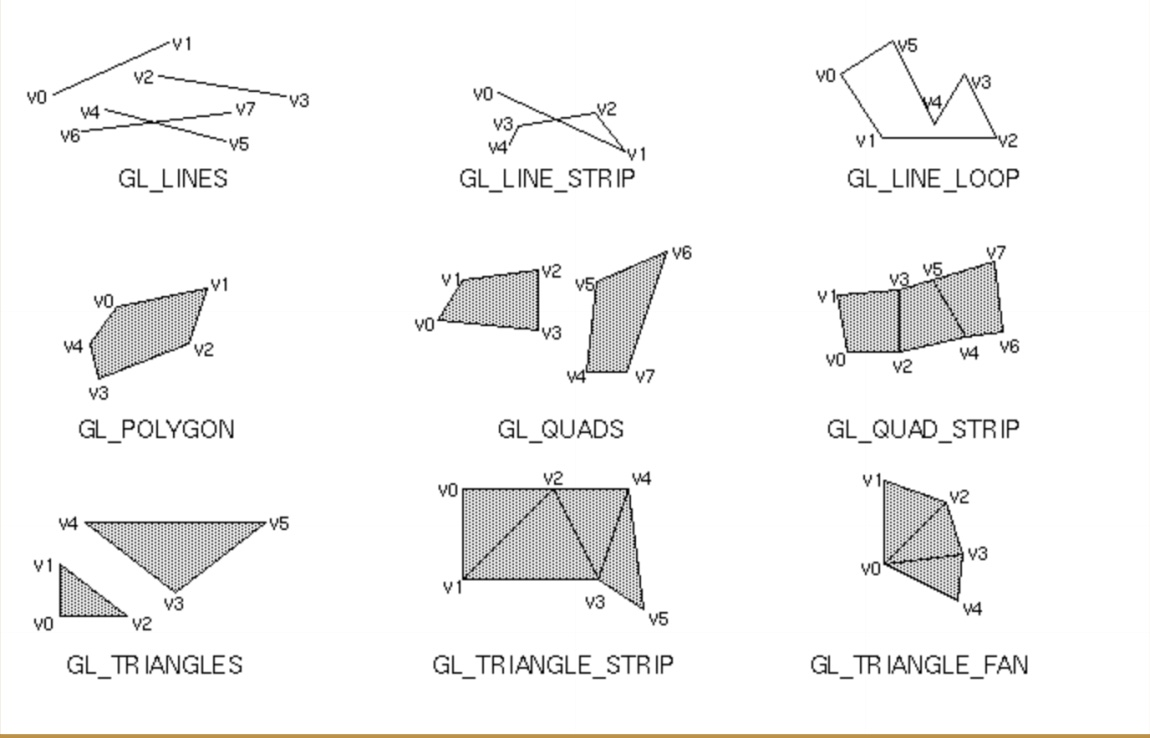`OpenGL`中的基本图元就是`点``线``三角形`等构成所有的图形，在这些基本元素组合中又抽象出一些基本图元，如上图所示。

• `GL_POINTS` :每个顶点都在屏幕上都是单独的点

• `GL_LINES`:每一对顶点定义一个线段

• `GL_LINE_LOOP`:和`GL_LINE_STRIP`相同，但是最后一个顶点和第一个顶点连接起来了

• `GL_TRIANGLES`:每3个顶点定义一个新的三角形

• `GL_TRIANGLE_STRIP`:公用一个条带（strip）上的顶点的一组三角形

• `GL_TRIANGLE_FAN`:以一个圆点为中心呈扇形排列，公用相邻顶点的一组三角形

### OpenGL中的点和线

``//1.最简单也是最常⽤的 4.0f,表示点的⼤⼩ glPointSize(4.0f); //2.设置点的⼤⼩范围和点与点之间的间隔GLfloat sizes = {2.0f,4.0f}; GLfloat step = 1.0f; //3.获取点⼤⼩范围和最⼩步⻓glGetFloatv(GL_POINT_SIZE_RANGE ,sizes); glGetFloatv(GL_POINT_GRAULARITY ,&step); //4.通过使⽤程序点⼤⼩模式来设置点⼤⼩glEnable(GL_PROGRAM_POINT_SIZE); //5.这种模式下允许我们通过编程在顶点着⾊器或⼏何着⾊器中设置点⼤⼩。着⾊器内建变量：gl_PointSize(内建变量)，并且可以在着⾊器源码直接写gl_PointSize = 5.0 //6. 设置线段宽度glLineWidth(2.5f);``

### OpenGL工具类GLBatch

``GLBatch ,是在GLTools中包含的⼀个简单容器类. void GLBatch::Begain(GLeunm primitive,GLuint nVerts,GLuint nTexttureUnints = 0); 参数1：图元参数2：顶点数参数3：⼀组或者2组纹理坐标（可选）//复制顶点数据(⼀个由3分量x,y,z顶点组成的数组) void GLBatch::CopyVerterxData3f(GLfloat *vVerts); //复制表⾯法线数据void GLBatch::CopyNormalDataf(GLfloat *vNorms); //复制颜⾊数据void GLBatch::CopyColorData4f(GLfloat *vColors); //复制纹理坐标数据void GLBatch::CopyTexCoordData2f(GLFloat *vTextCoords, GLuint uiTextureLayer); //结束数据复制void GLBatch::End(void); //绘制图形void GLBatch::Draw(void);``

]]>
<h2 id="OpenGL渲染流程解析"><a href="#OpenGL渲染流程解析" class="headerlink" title="OpenGL渲染流程解析"></a>OpenGL渲染流程解析</h2><ul> <li><code>attrubutes</code> 属性只可传给 <code>Vertex Shader(顶点着色器)</code></li> <li>通过<code>顶点着色器</code>向 <code>Primitive(几何图元)</code> 传递 <code>Position</code> 和 <code>Outs</code></li> <li>可以通过 <code>OpenGl API</code> 向顶点着色器或者片元着色器</li> <li><code>Texture Data(纹理数据)</code>也可以同时传递给顶点着色器和片元着色器，但是真正用它的是片元着色器。
OpenGL：图像的渲染、撕裂、掉帧 http://typeco.cn/posts/86ed34e7/ 2020-07-09T02:40:19.000Z 2020-07-09T06:26:15.035Z

## CPU和GPU

### CPU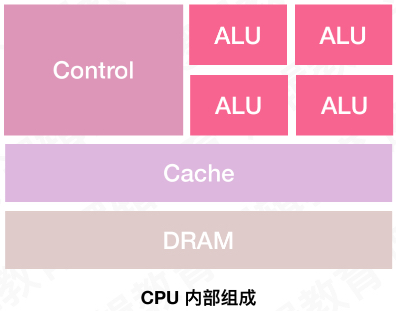### GPU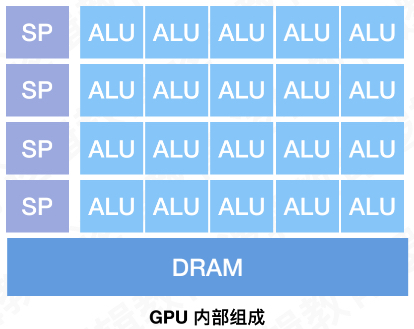## 计算机渲染原理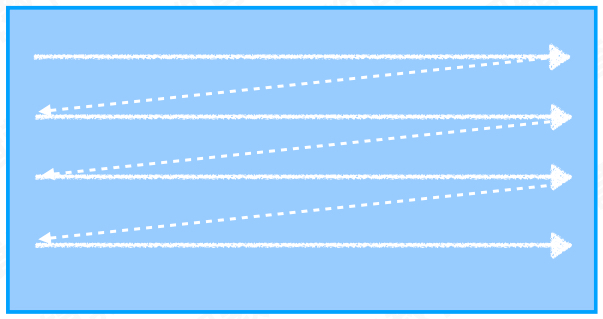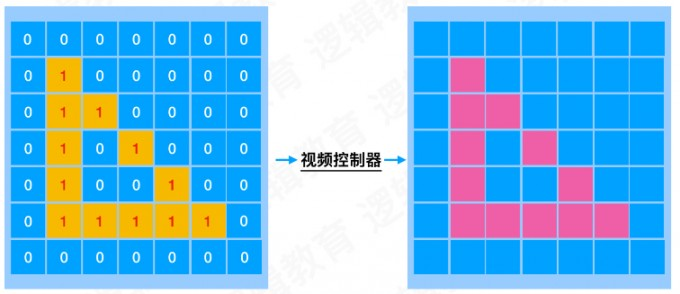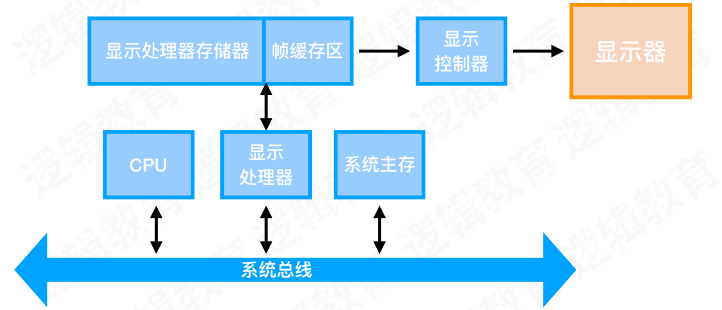## 撕裂和掉帧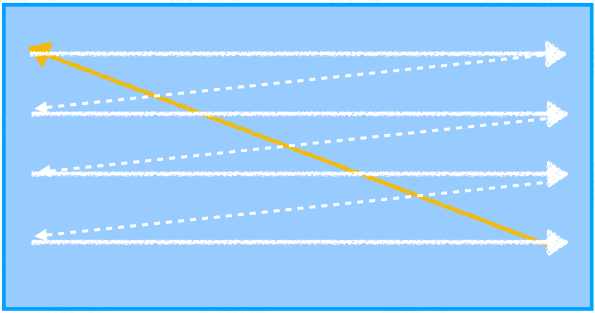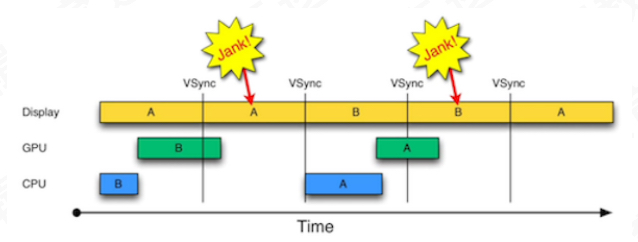GPU 开辟AB两个缓冲区，并对缓冲区进行同步加锁处理，执行流程就是当A缓冲区拿到第一帧数据，就给A缓冲区加上一把锁，屏幕控制器从A拿到数据并逐行扫描完成，A帧缓冲区解锁，并把屏幕控制器指向B缓冲区，B缓冲区加锁并逐行扫描显示，在屏幕控制器扫描B缓冲区的时候，A缓冲区拿到GPU传过来的新数据，以此类推，解决撕裂问题。

• CPU/GPU渲染流水线耗时过长导致掉帧
• 垂直同步 + 双缓冲区 解决`图像撕裂`问题，但是会造成掉帧问题
• 三级缓冲区的开辟是为了合理使用CPU/GPU以达到减少掉帧的次数

## IOS下的渲染框架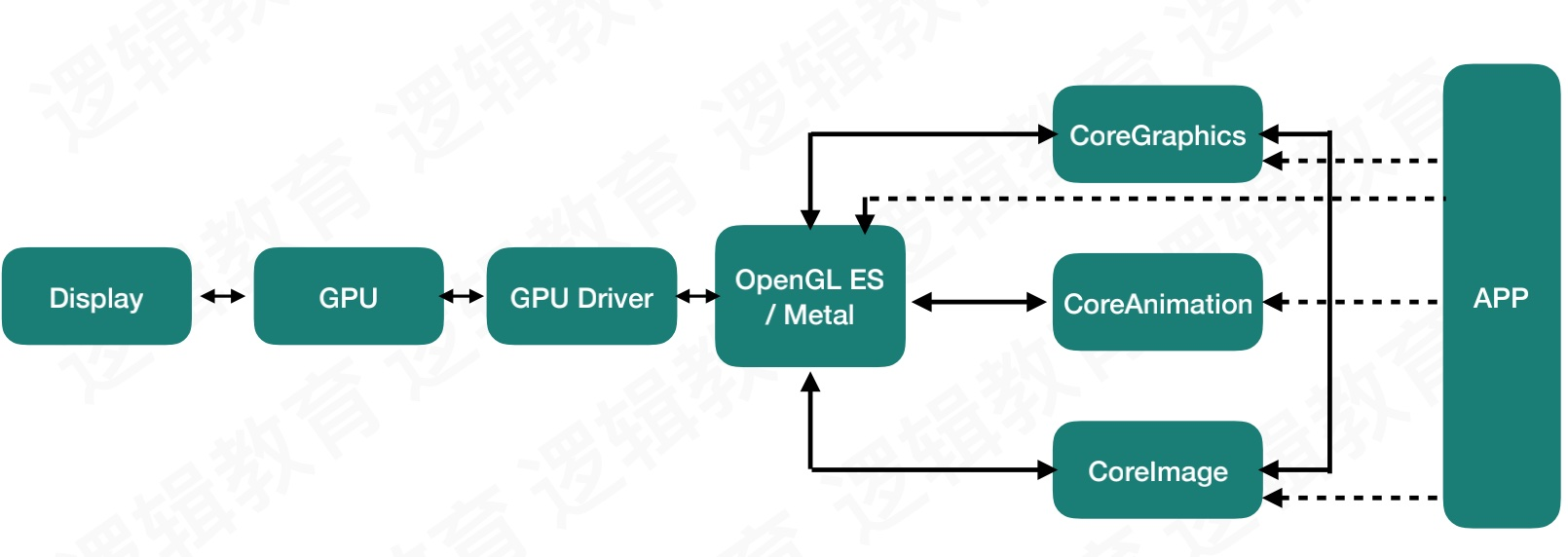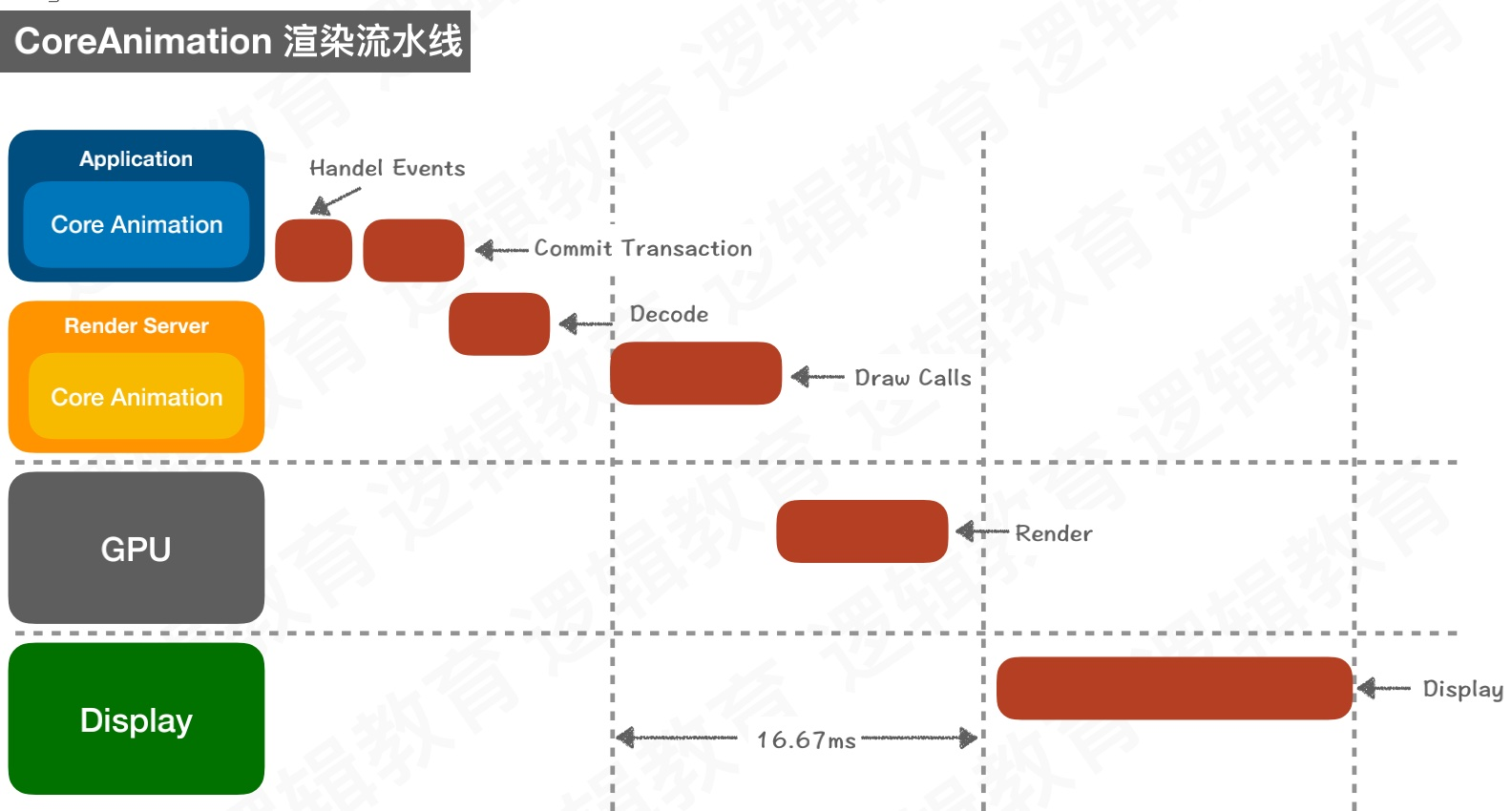## 总结

]]>
OpenGL：你真的懂离屏渲染吗？ http://typeco.cn/posts/93a4c1aa/ 2020-07-07T14:42:05.000Z 2020-07-09T06:10:58.129Z

## 检测离屏渲染

`ViewController.m` 中写入以下代码：

``    // 1. 按钮：图片 + 背景    UIButton *btn1 = [UIButton buttonWithType:UIButtonTypeCustom];    btn1.frame = (CGRect){100,30,100,100};    btn1.backgroundColor = [UIColor blueColor];    [btn1 setImage:[UIImage imageNamed:@"weixin"] forState:UIControlStateNormal];    btn1.clipsToBounds = YES;    btn1.layer.cornerRadius = 50;    [self.view addSubview:btn1];    //2. 按钮 ： 只有背景    UIButton *btn2 = [UIButton buttonWithType:UIButtonTypeCustom];    btn2.frame = (CGRect){100,180,100,100};    btn2.backgroundColor = [UIColor blueColor];    btn2.layer.cornerRadius = 50;    btn2.clipsToBounds = YES;    [self.view addSubview:btn2];    // 3. UIImageView  图片 + 颜色    UIImageView *img1 = [UIImageView new];    img1.frame = (CGRect){100,320,100,100};    img1.backgroundColor = [UIColor blueColor];    img1.image = [UIImage imageNamed:@"weixin"];    img1.layer.cornerRadius = 50;    img1.layer.masksToBounds = YES;    [self.view addSubview:img1];    // 4. UIImageView  只有图片    UIImageView *img2 = [UIImageView new];    img2.frame = (CGRect){100,480,100,100};    img2.image = [UIImage imageNamed:@"weixin"];    img2.layer.cornerRadius = 50;    img2.layer.masksToBounds = YES;    [self.view addSubview:img2];``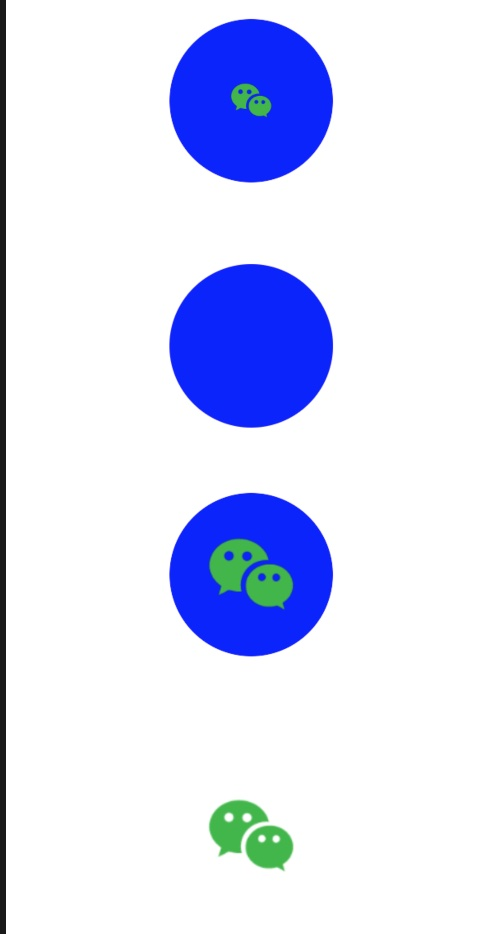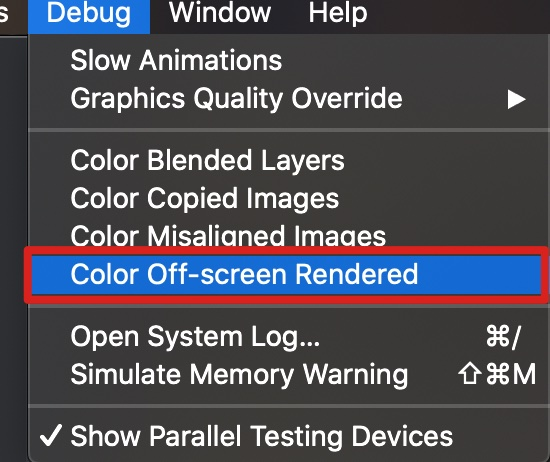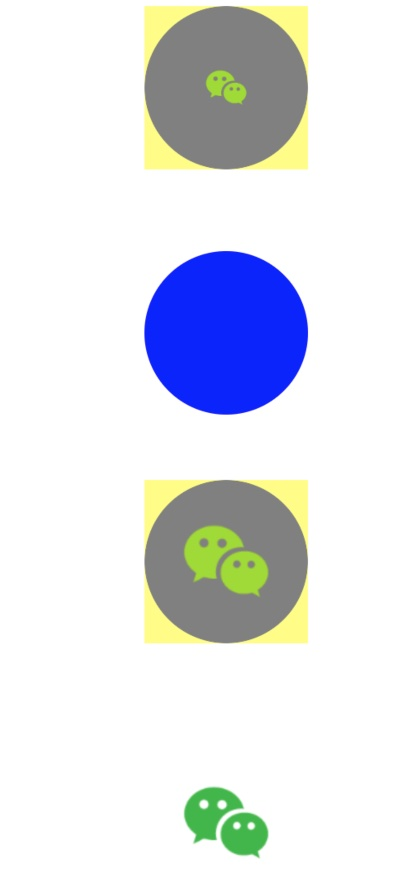~不对啊，不是说好的 `cornerRadius``clipsToBounds` 会触发离屏渲染吗，他们都用了这些属性啊，那么为什么同样是按钮，同样是UIImageView，为什么一个有离屏渲染，而另外一个却没有呢，这和我们理解的似乎并不一致。

## 渲染流程

### 普通渲染

`普通渲染` 就是我们通常比如把一个图片显示到屏幕上，它的过程是：先把图片的数据转换成像素数据然后传到`帧缓冲区`渲染成图像，紧接着数据从`帧缓冲区` 交给显示控制器最终显示到显示器上。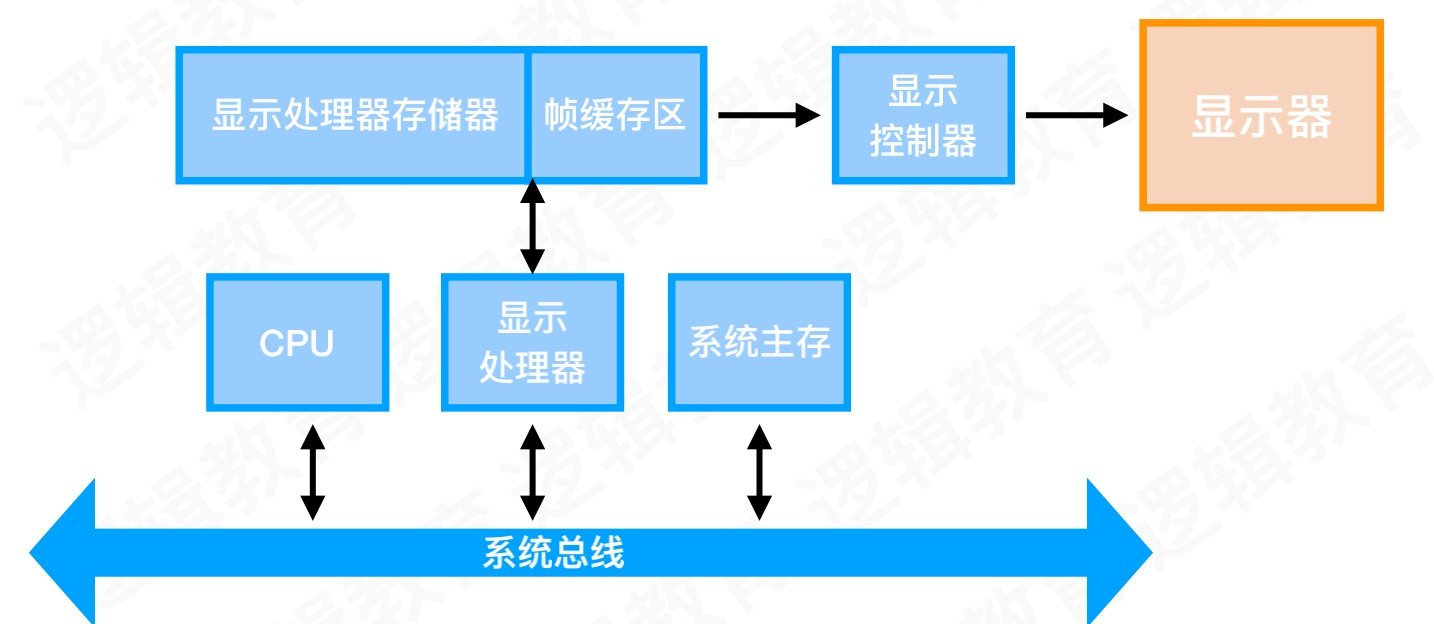### 离屏渲染

`离屏渲染` 为什么叫离屏，就是因为系统在处理这种渲染情况下，会额外开辟一块离屏缓冲区，用于存放需要离屏渲染的图层数据，等到各个图层数据准备整合完毕就会将最终的图层交给显示控制器来显示。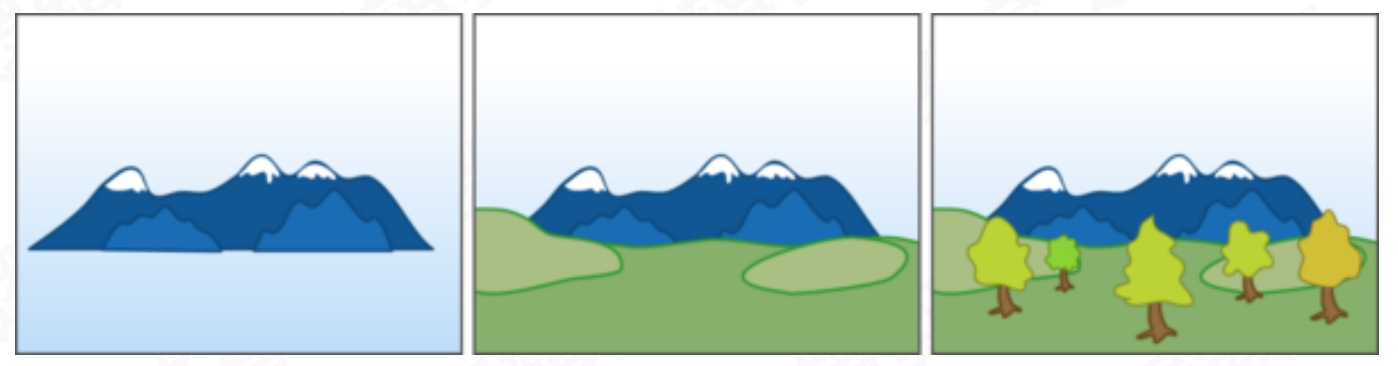### 渲染过程对比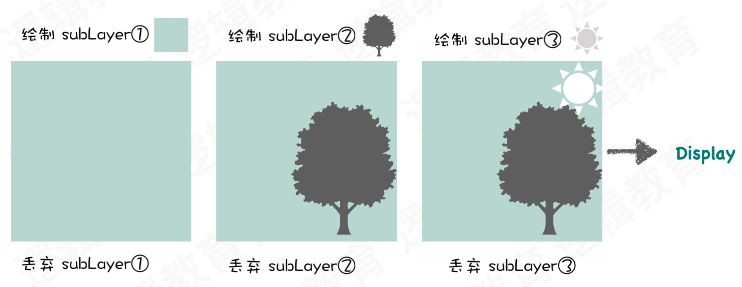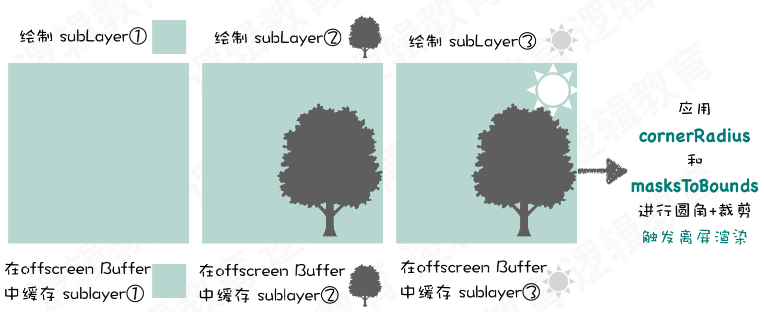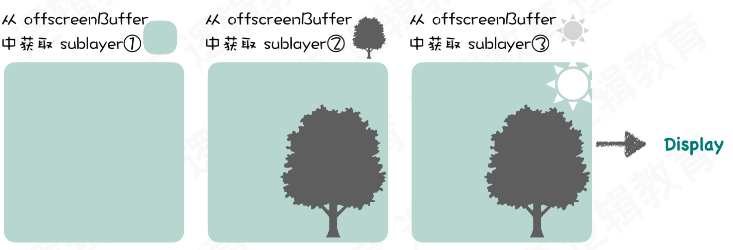`圆角`并不会导致离屏渲染，导致离屏渲染的核心是 `view.layer.maskToBounds= YES`

### UIImageView 离屏渲染的原因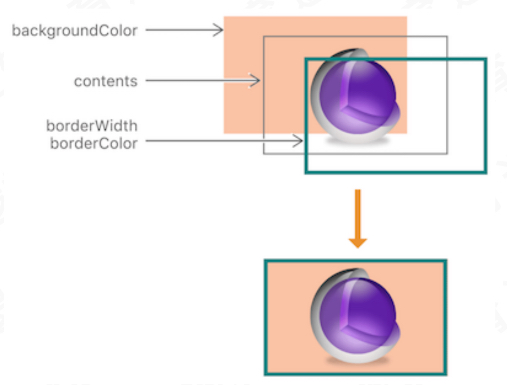## 触发离屏渲染的情景

• 使用了 `mask``layer (layer.mask)`
• 需要进行裁剪的 `layer(layer.maskToBounds 或 view.clipsToBounds)`
• 设置了组透明度YES，并且透明度不为 1 的 layer `layer.allowsGroupOpacity 或 layer.opacity`
• 添加了投影的layer `layer.shadow*`
• 采用了光栅化的layer `(layer.shouldRasterize)`
• 绘制了文字的layer `(UILabel,CATextLayer,CoreText 等)`

## 圆角处理方案

### 方案一

``btn2.layer.cornerRadius = 50;btn2.clipsToBounds = YES;``

### 方案二 (UIImage+Category)

``- (UIImage *)viewWithCornerRadius:(CGFloat)cornerRadius {    CGFloat w = self.size.width;    CGFloat h = self.size.height;    CGFloat scale = [UIScreen mainScreen].scale;    if (cornerRadius < 0) {        cornerRadius = 0;    } else if (cornerRadius > MIN(w, h)) {        cornerRadius = MIN(w, h)/2;    }    UIImage *image = nil;    CGRect imageFrame = (CGRect){0,0,w,h};    UIGraphicsBeginImageContextWithOptions(self.size, NO, scale);    [[UIBezierPath bezierPathWithRoundedRect:imageFrame cornerRadius:cornerRadius] addClip];    [self drawInRect:imageFrame];    image = UIGraphicsGetImageFromCurrentImageContext();    UIGraphicsEndImageContext();    return image;}``

### 方案三 (YYWebImage)

`推荐`

``- (UIImage *)yy_imageByRoundCornerRadius:(CGFloat)radius                                 corners:(UIRectCorner)corners                             borderWidth:(CGFloat)borderWidth                             borderColor:(UIColor *)borderColor                          borderLineJoin:(CGLineJoin)borderLineJoin {    if (corners != UIRectCornerAllCorners) {        UIRectCorner tmp = 0;        if (corners & UIRectCornerTopLeft) tmp |= UIRectCornerBottomLeft;        if (corners & UIRectCornerTopRight) tmp |= UIRectCornerBottomRight;        if (corners & UIRectCornerBottomLeft) tmp |= UIRectCornerTopLeft;        if (corners & UIRectCornerBottomRight) tmp |= UIRectCornerTopRight;        corners = tmp;    }    UIGraphicsBeginImageContextWithOptions(self.size, NO, self.scale);    CGContextRef context = UIGraphicsGetCurrentContext();    CGRect rect = CGRectMake(0, 0, self.size.width, self.size.height);    CGContextScaleCTM(context, 1, -1);    CGContextTranslateCTM(context, 0, -rect.size.height);    CGFloat minSize = MIN(self.size.width, self.size.height);    if (borderWidth < minSize / 2) {        UIBezierPath *path = [UIBezierPath bezierPathWithRoundedRect:CGRectInset(rect, borderWidth, borderWidth) byRoundingCorners:corners cornerRadii:CGSizeMake(radius, borderWidth)];        [path closePath];        CGContextSaveGState(context);        [path addClip];        CGContextDrawImage(context, rect, self.CGImage);        CGContextRestoreGState(context);    }    if (borderColor && borderWidth < minSize / 2 && borderWidth > 0) {        CGFloat strokeInset = (floor(borderWidth * self.scale) + 0.5) / self.scale;        CGRect strokeRect = CGRectInset(rect, strokeInset, strokeInset);        CGFloat strokeRadius = radius > self.scale / 2 ? radius - self.scale / 2 : 0;        UIBezierPath *path = [UIBezierPath bezierPathWithRoundedRect:strokeRect byRoundingCorners:corners cornerRadii:CGSizeMake(strokeRadius, borderWidth)];        [path closePath];        path.lineWidth = borderWidth;        path.lineJoinStyle = borderLineJoin;        [borderColor setStroke];        [path stroke];    }    UIImage *image = UIGraphicsGetImageFromCurrentImageContext();    UIGraphicsEndImageContext();    return image;}``

]]>
<p>作为一个IOS开发者，<code>离屏渲染</code> 这个名词或许你并不陌生，或多或少你都能说点什么，比如我们通常最简单的圆角方式 <code>cornerRadius</code> 和 <code>clipsToBounds</code> 会触发离屏渲染，会影响性能….
OpenGL：基础概念以及项目搭建 http://typeco.cn/posts/7f4eafc3/ 2020-07-03T09:36:25.000Z 2020-07-05T15:46:38.391Z

## 基本概念一览

• OpenGL： PC图形图像渲染处理，主要针对MAC、windows平台

• OpengGL ES： ES是嵌入式的意思，主要针对移动端（IOS、安卓）

• OpenCV： 主要是识别（人脸识别、身份识别、物体 -> OpenCV， face++）主要是与人工智能结合

• Metal： 苹果底层使用的，在2018年前苹果底层渲染部分->CoreAnimation -> 基于OpenGL ES 封装的上层框架；2018年以后底层渲染迁移到Metal

• 图形API解决了哪些问题：

• 系统的按钮、图片、视图、图层等渲染都是通过图形API来完成的
• 游戏引擎-> 人物、场景的渲染
• 视频播放的框架 -> ijkplayer，kxmovie，视频解码 -> 渲染
• 核心动画 -> 动画操作（旋转、缩放、移动、图层特效）
• 视频、图片等特效
• 上下文（context）：

• 应用程序调用任何OpenGL指令之前，需要安排首先创建一个OpengGL的上下文。这个上下文是一个非常庞大的状态机，保存了OpengGL中的各种状态，这也是OpenGL执行指令的基础
• OpenGL的函数不论在哪个语言中，都是类C语言一样的面向过程函数，本质上都是对OpenGL上下文这个庞大的状态机中的某个状态或者对象进行操作，当然你得先把这个对象设置为当前对象。因此，通过对OpenGL指令的封装，是可以把OpenGL的相关调用封装成为一个面向对象的图形API的
• 由于OpenGL上下文是一个巨大的状态机，切换上下文往往会产生较大的开销，但是不同的绘制模块，可能需要使用完全独立的状态管理。因此，可以在应用程序中分别创建多个不同的上下文，在不同的线程中使用不同的上下文，上下文之间共享纹理、缓冲区等资源。这样的方案，会比反复切换上下文，或者大量修改渲染状态更加合理高效。
• OpenGL状态机：

• 理论上是一个机器，其实可以这么理解，状态机描述了一个对象在其生命周期内所经历的各种状态，状态机描述了一个对象在其生命周期内所经历的各种状态，状态间的转变，发生转变的动因，条件及转变中所执行的活动。或者说，状态机是一种行为，说明对象在其生命周期中相应事件所经历的状态序列以及对那些状态事件的相应
• 有记忆功能，能记住其当前的状态
• 可以接收输入，根据输入的内容和自己的原先的状态，修改自己当前的状态，并且可以有对应的输出
• 当进入特殊状态（停机等）的时候，便不再接收输入，停止工作
• 渲染（Rendering）： 将图形、图像数据转换成2D空间图像的操作叫渲染

• 图片、按钮、视频 -> 解码图片 - > 渲染
• 顶点数组（VertexArray）| 顶点缓冲区： OpenGL中的图像都是由图元组成的，在OpenGL ES中有3中类型的图元：点、线、三角形。那这部分图元数据是如何存储的呢？开发者可以选择设定函数指针，在调用绘制方法的时候，直接由内存传入顶点数据，也就是说这部分数据之前是存储在内存当中，被称为顶点数组。而性能更高的做法是，提前分配一块显存，将顶点数据预先传入到显存当中。这部分显存就被称为顶点缓冲区

• 位图： 比如我们120120像素的图片 = 14400 像素 ,然后每个像素又包含RGBA 每个位置8个字节总共 4位 所以 144004 的纹理，纹理就是位图

• 管线（流水线）：其实就是一个制作的过程，分为固定管线和可编程管线

• 固定管线：对象非常多固定的模具（固定着色器）-> 结果（OpenGL 固定的画面）
• 可编程管线：可自定义的着色器，相当于个人定制的模块
• 着色器： 其实就是 函数、方法或者代码段 -> CPU使用 ；shader代码段 -> GPU来调用

• 渲染过程中，必须存储两种着色器，分别是顶点着色器和片元着色器。顶点着色器是第一个着色器，片元着色器是对最后一个。顶点着色器处理顶点，片元着色器处理其他像素点的颜色。

• 顶点着色器：用来处理顶点相关的代码：1.确定位置 2.缩放、平移、旋转等位置换算 3.手机端显示3D屏幕实际是2D，3D图形数据 -> 2D（投影换算） -> OpenGL ES

• 片元着色器：是处理一个一个像素点的，GPU并行运算，比如图片进行饱和度调整，那么就是片元着色器进行一个一个像素点的修改 ，因为GPU是并发运算，所以效率会很高。

• 固定着色器：苹果提供的固定API，固定代码片段，调用参数生成，OpenGL来提供

• 自定义着色器：进行自定义（基于GLSL语法来进行编写的代码段）

• GLSL 是一种语言，自定义着色器的语言（代码），OpenGL标准调用GPU

• 光栅化（Rasterization）： 是把顶点数据转换为片元的过程，片元中的每一个元素对应帧缓冲区中的一个像素，分为两个过程，都不可控制也不可编程

• 第一个过程：确定图形的像素范围（决定窗口坐标中哪些整形栅格区域被基本图元占用）
• 第二个过程：颜色附着上去（分配一个颜色值和一个深度值到各个区域）
• 光栅化过程是产生片元的过程
• 纹理： 纹理其实就是位图，也可以理解为图片，大家在渲染图形的时候需要在其编码填充图片，为了使得场景更加逼真，而这里使用的图片就是常说的纹理，但是在OpenGL，我们更加习惯叫纹理而不是图片。

• 混合（Blending）： 在测试阶段之后，如果像素依然没有被剔除，那么像素的颜色将会和帧缓冲区中颜色附着上的颜色进行混合，混合的算法可以通过OpenGL的函数进行制定，但是OpenGL提供的缓和算法是有限的，如果需要更加复杂的缓和算法，一般可以通过像素着色器进行实现，当然性能会比原生的混合算法差一些。

• 变换矩阵（Transformation）： 图形想发生平移、缩放、旋转变换等，就需要使用变换矩阵

• 投影矩阵（Projection）： 用于将3D坐标转换为二维屏幕坐标，实际线条也将在二维坐标下进行绘制。

• 渲染上屏： 如果将图像直接渲染到窗口对应的渲染缓冲区，则可以将图像显示到屏幕上。值得注意的是，如果每个窗口只有一个缓冲区，那么在绘制的过程中屏幕进行了刷新，窗口可能显示出不完整的图像，为了解决这个问题，常规的OpengGL程序至少都会有两个缓冲区。显示在屏幕上的称为屏幕缓冲区，没有显示的称为离屏缓冲区，在一个缓冲区渲染完成之后，通过将屏幕缓冲区和离屏缓冲区交换，实现图像在屏幕上的显示。

• 由于显示器的刷新是逐行进行的，因此为了防止交换缓冲区的时候屏幕上下区域的图像分属于两个不同的帧信号，因此交换一般会等待显示器刷新完成的信号，在显示器两次刷新的间隔中进行交换，这个信号被称为垂直同步信号，这个技术被称为垂直同步。这个就是双缓冲区技术。
• 在双缓冲区和垂直同步技术之后，由于总是要等待缓冲区交换之后再进行下一帧的渲染，使得帧率无法完全达到硬件允许的最高水平。为了解决这个问题，引入了三缓冲区技术，在等待垂直同步的同时来回交替两个离屏的缓冲区，而垂直同步发生时，屏幕缓冲区和最近渲染完成的离屏缓冲区交换，实现充分利用硬件性能的目的。

## MAC环境和项目搭建

### 创建工程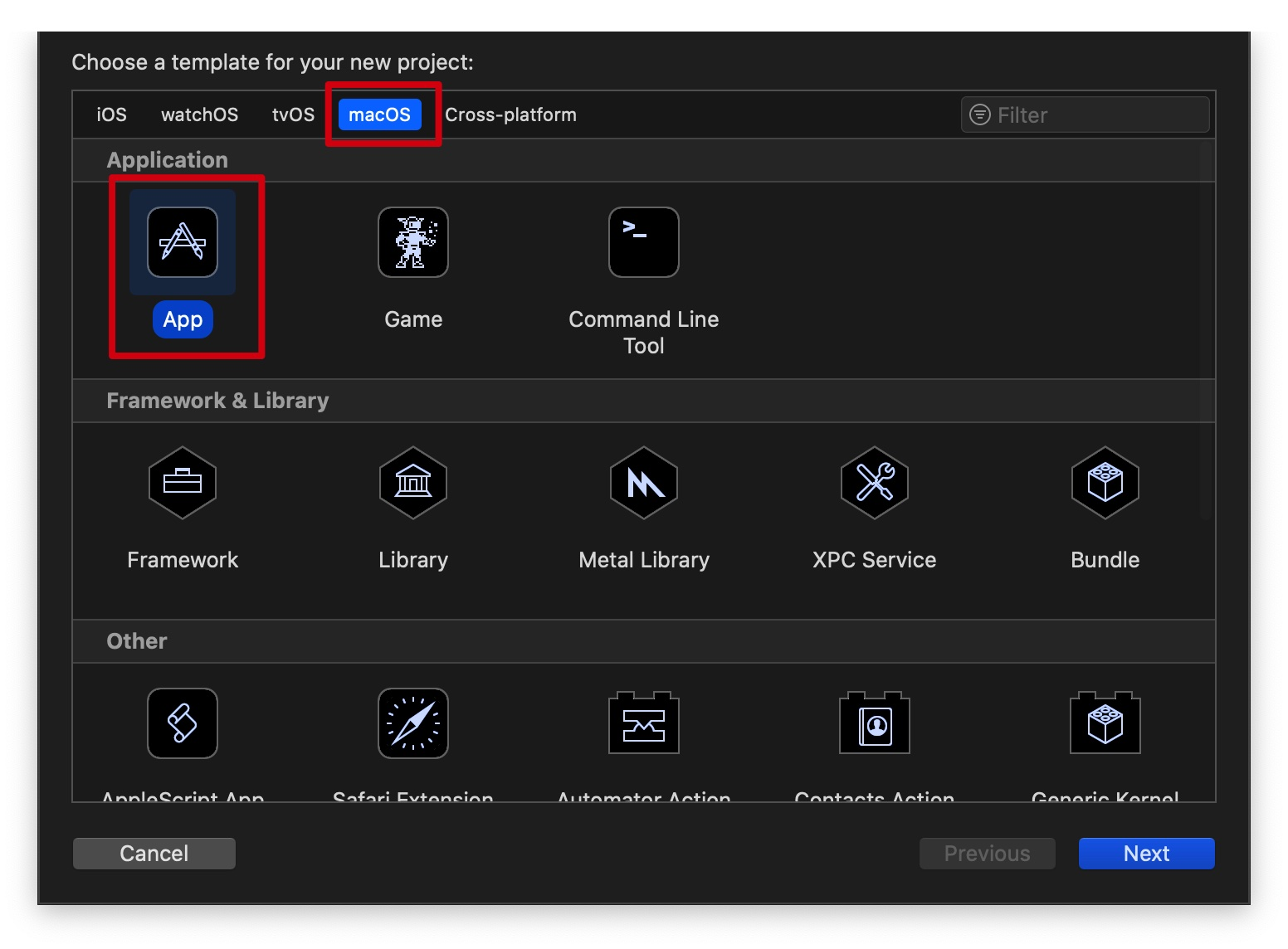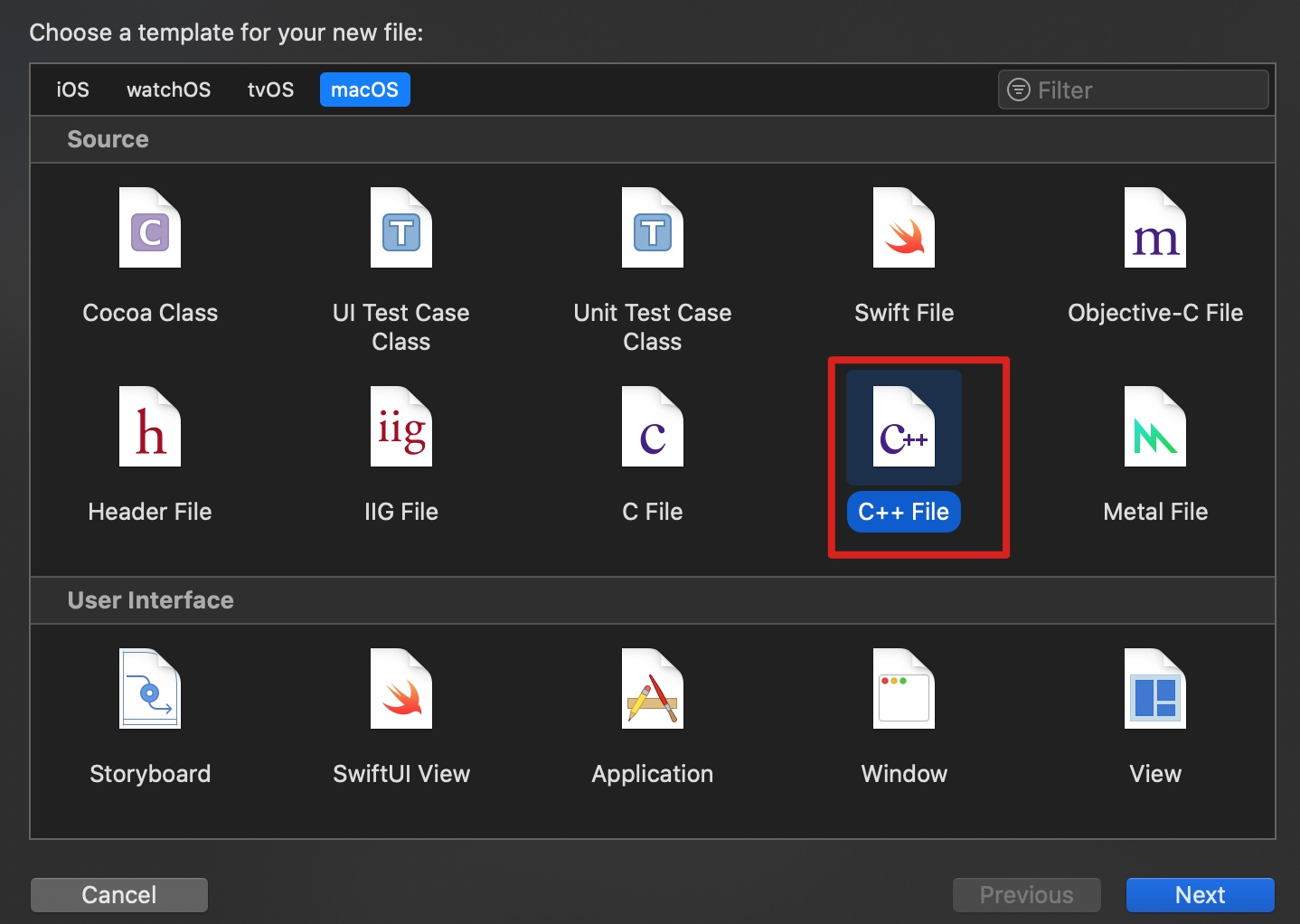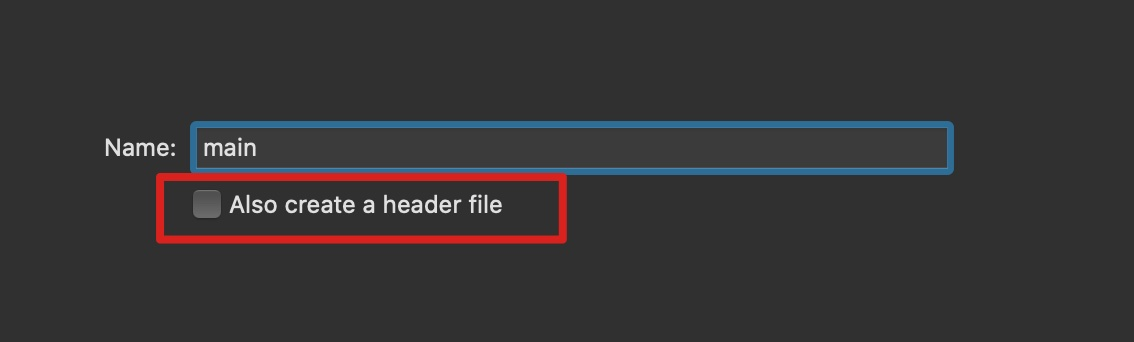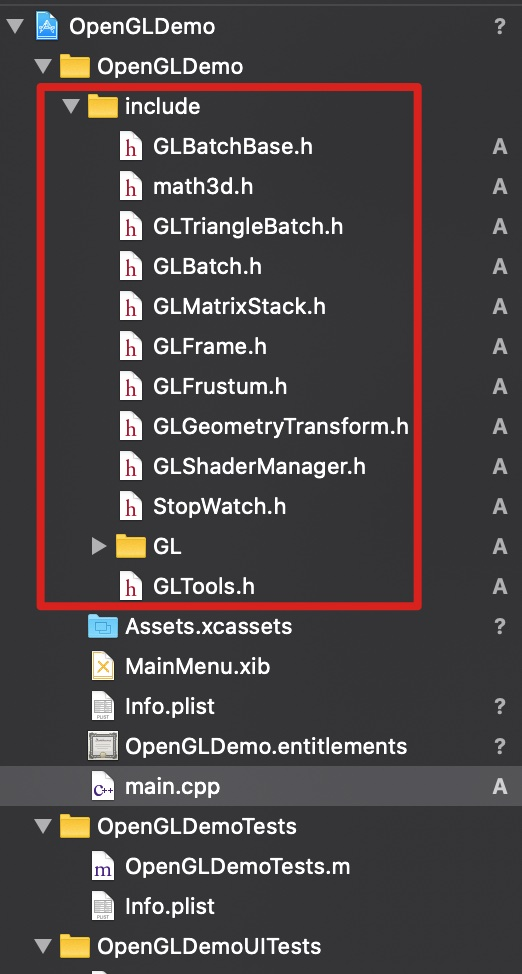LibGLTool.a文件拖到framework文件夹下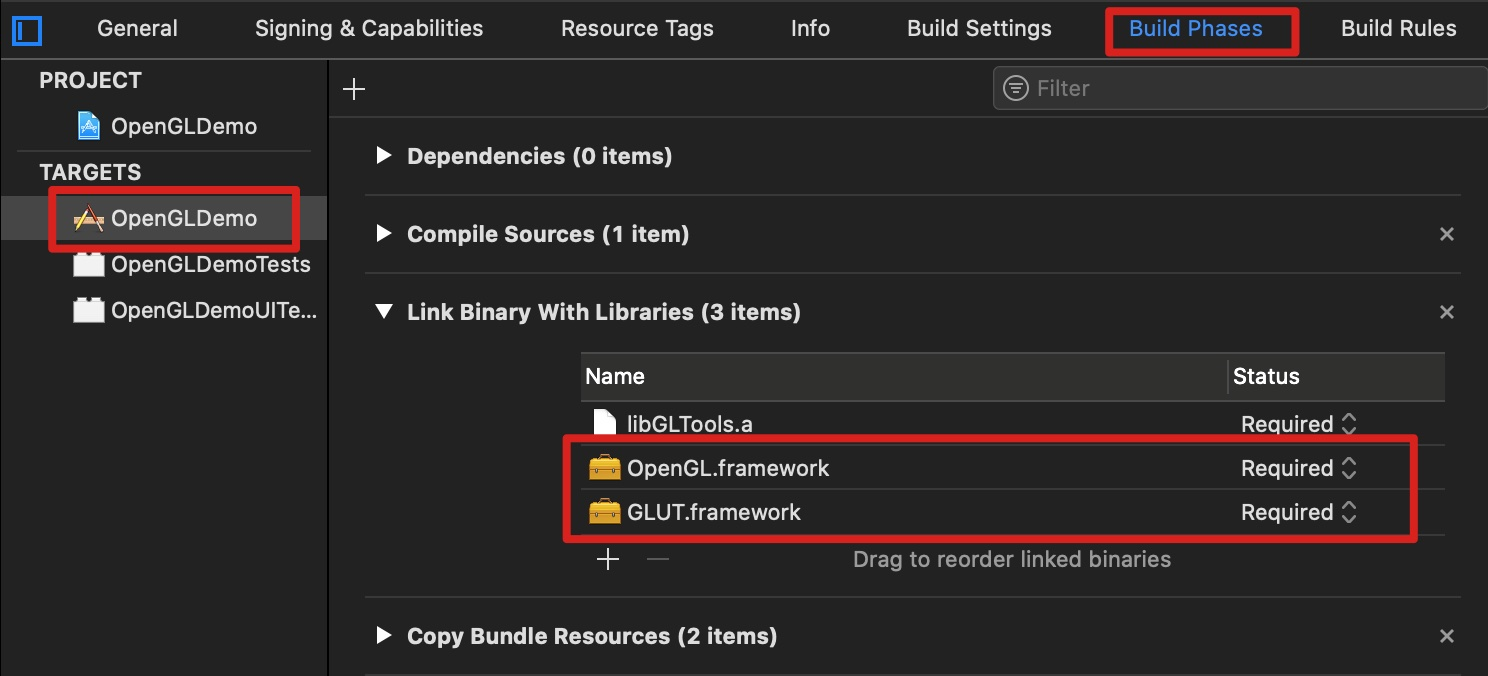``#include "GLShaderManager.h"#include "GLTools.h"#include <GLUT/GLUT.h>//定义一个，着色管理器GLShaderManager shaderManager;//简单的批次容器，是GLTools的一个简单的容器类。GLBatch triangleBatch;/*在窗口大小改变时，接收新的宽度&高度。*/void changeSize(int w,int h){    glViewport(0, 0, w, h);}void RenderScene(void){    //1.清除一个或者一组特定的缓存区    glClear(GL_COLOR_BUFFER_BIT | GL_DEPTH_BUFFER_BIT|GL_STENCIL_BUFFER_BIT);    //2.设置一组浮点数来表示红色    GLfloat vRed[] = {1.0,0.5,0.0,1.0f};    //传递到存储着色器，即GLT_SHADER_IDENTITY着色器，这个着色器只是使用指定颜色以默认笛卡尔坐标第在屏幕上渲染几何图形    shaderManager.UseStockShader(GLT_SHADER_IDENTITY,vRed);    //提交着色器    triangleBatch.Draw();    //将后台缓冲区进行渲染，然后结束后交换给前台    glutSwapBuffers();}void setupRC(){    //设置清屏颜色（背景颜色）    glClearColor(0.98f, 0.90f, 0.3f, 1);    //没有着色器，在OpenGL 核心框架中是无法进行任何渲染的。初始化一个渲染管理器。    //在前面的课程，我们会采用固管线渲染，后面会学着用OpenGL着色语言来写着色器    shaderManager.InitializeStockShaders();    //指定顶点    //在OpenGL中，三角形是一种基本的3D图元绘图原素。    GLfloat vVerts[] = {        -0.5f,0.0f,0.0f,        0.5f,0.0f,0.0f,        0.0f,0.5f,0.0f    };    triangleBatch.Begin(GL_TRIANGLES, 3);    triangleBatch.CopyVertexData3f(vVerts);    triangleBatch.End();}int main(int argc,char *argv[]){    //初始化GLUT库,这个函数只是传说命令参数并且初始化glut库    glutInit(&argc, argv);    /*    初始化双缓冲窗口，    */    glutInitDisplayMode(GLUT_DOUBLE|GLUT_RGBA|GLUT_DEPTH|GLUT_STENCIL);    //GLUT窗口大小、窗口标题    glutInitWindowSize(800, 600);    glutCreateWindow("Triangle");    /*    GLUT 内部运行一个本地消息循环，拦截适当的消息。然后调用我们不同时间注册的回调函数。    */    //注册重塑函数    glutReshapeFunc(changeSize);    //注册显示函数    glutDisplayFunc(RenderScene);    /*    初始化一个GLEW库,确保OpenGL API对程序完全可用。    在试图做任何渲染之前，要检查确定驱动程序的初始化过程中没有任何问题    */    GLenum status = glewInit();    if (GLEW_OK != status) {        printf("GLEW Error:%s\n",glewGetErrorString(status));        return 1;    }    //设置我们的渲染环境    setupRC();    glutMainLoop();    return  0;}``]]>
<p>最近开始学习OpenGL相关知识，针对学习的知识点以及学习的过程做一些笔记，固记录下来以便后续查阅和大家共同学习。

## 初识图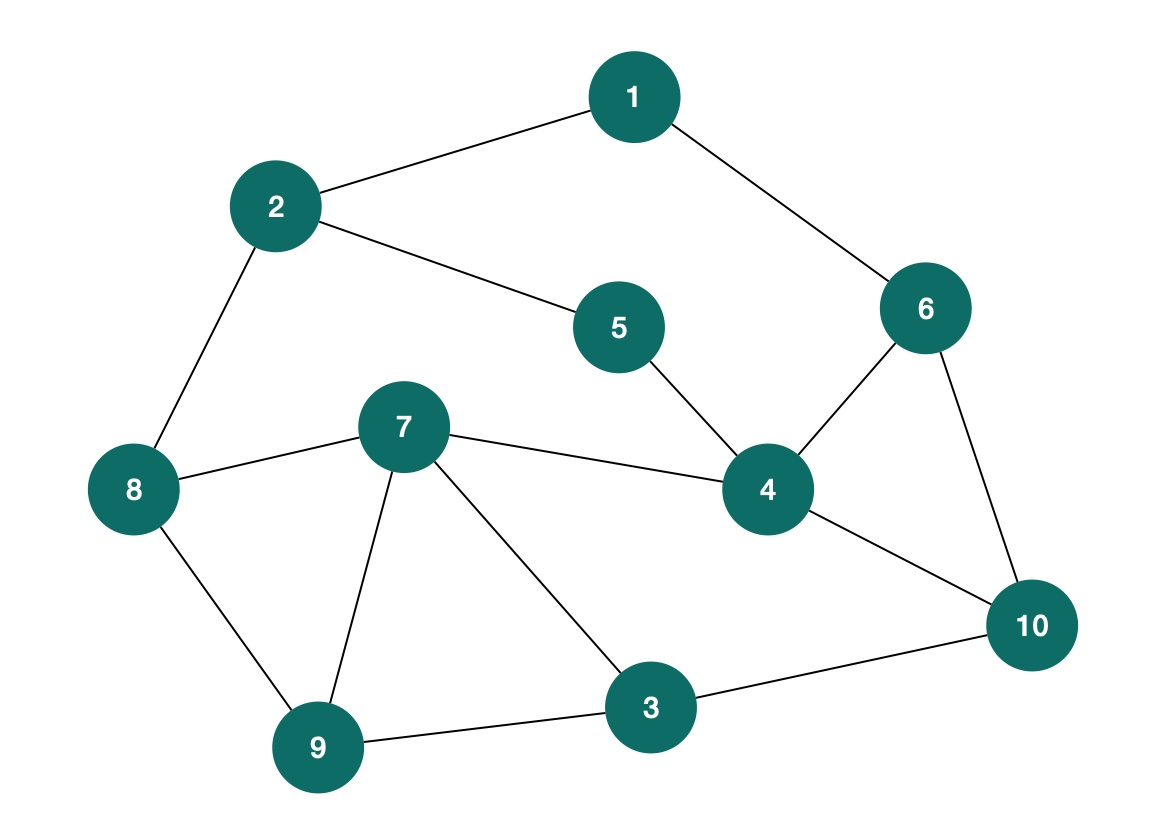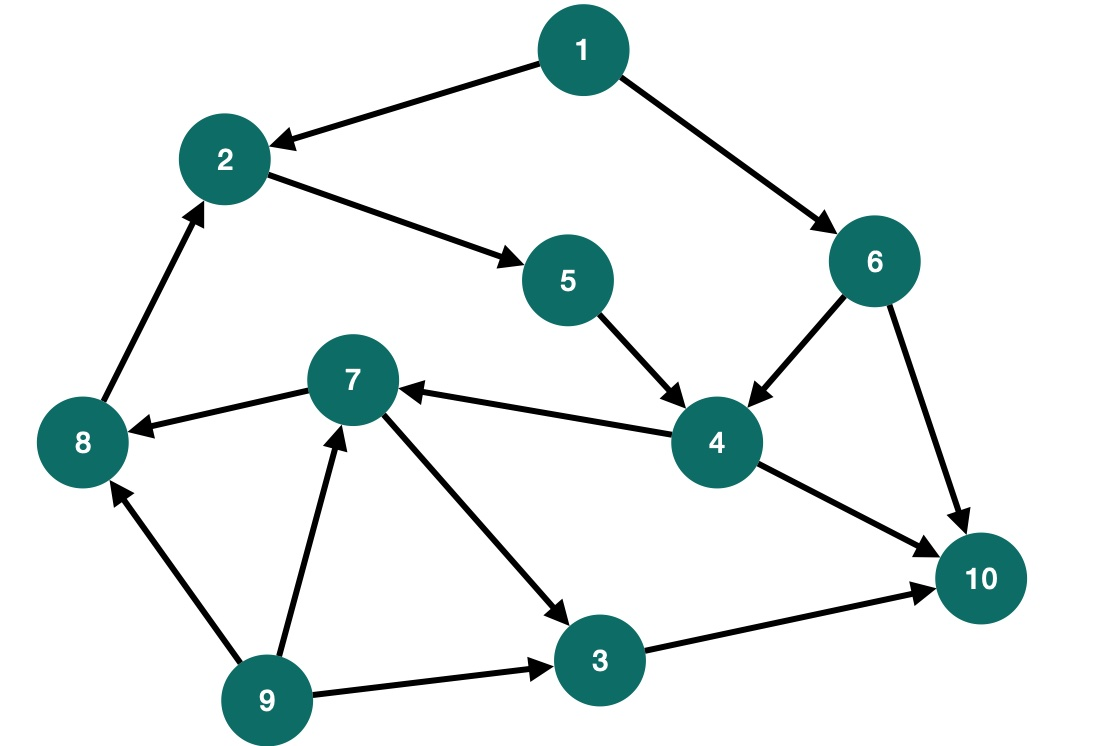## 图的存储思考

### 邻接矩阵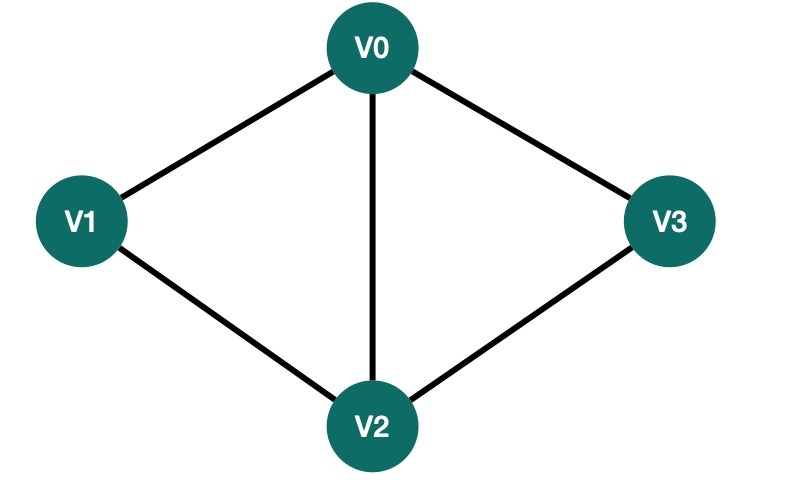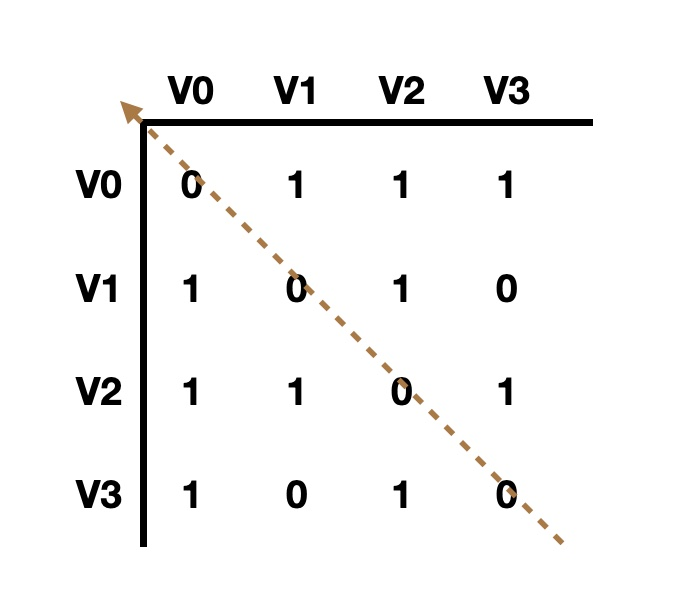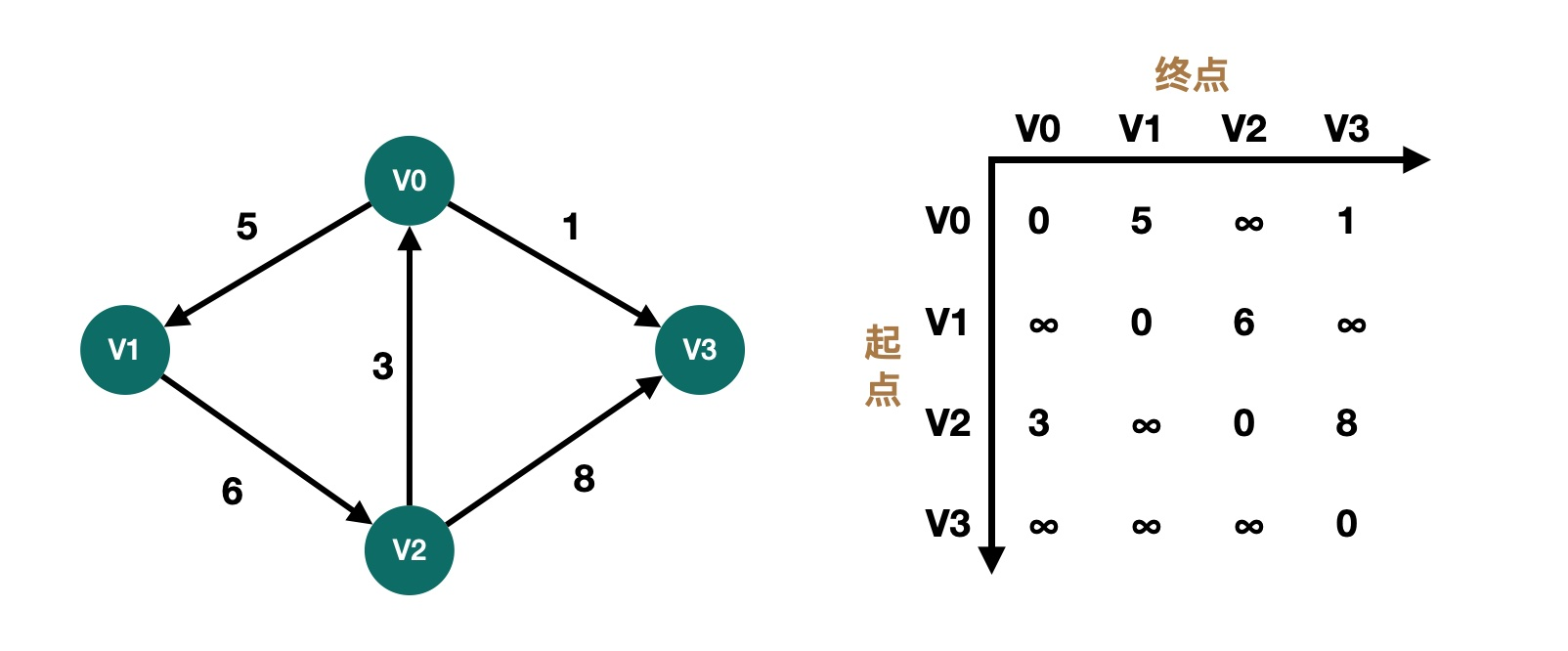``#define MAXVEX 100 /* 最大顶点数，应由用户定义 */#define INFINITYC 0typedef int Status;    /* Status是函数的类型,其值是函数结果状态代码，如OK等 */typedef char VertexType; /* 顶点类型应由用户定义  */typedef int EdgeType; /* 边上的权值类型应由用户定义 */typedef struct{    VertexType vexs[MAXVEX]; /* 顶点表 */    EdgeType arc[MAXVEX][MAXVEX];/* 邻接矩阵，可看作边表 */    int numNodes, numEdges; /* 图中当前的顶点数和边数  */}MGraph;``

``void CreateMGraph(MGraph *G){    int i,j,k,w;    printf("输入顶点数和边数:\n");    //1. 输入顶点数/边数    scanf("%d,%d",&G->numNodes,&G->numEdges);    printf("顶点数:%d,边数:%d\n",G->numNodes,G->numEdges);    //2.输入顶点信息/顶点表    for(i = 0; i<= G->numNodes;i++)        scanf("%c",&G->vexs[i]);    //3.初始化邻接矩阵    for(i = 0; i < G->numNodes;i++)         for(j = 0; j < G->numNodes;j++)             G->arc[i][j] = INFINITYC;    //4.输入边表信息    for(k = 0; k < G->numEdges;k++){        printf("输入边(vi,vj)上的下标i,下标j,权w\n");        scanf("%d,%d,%d",&i,&j,&w);        G->arc[i][j] = w;        //如果无向图,矩阵对称;        G->arc[j][i] = G->arc[i][j];    }    /*5.打印邻接矩阵*/    for (int i = 0; i < G->numNodes; i++) {        printf("\n");        for (int j = 0; j < G->numNodes; j++) {            printf("%d ",G->arc[i][j]);        }    }    printf("\n");}``

### 邻接表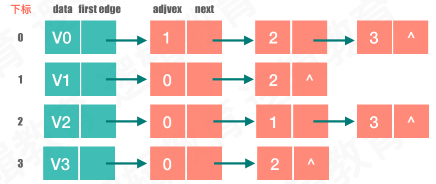``//邻接表的节点typedef struct Node{    int adj_vex_index;  //弧头的下标，也就是被指向的下标    Element data;       //权重值    struct Node * next; //边指针}EdgeNode;//顶点节点表typedef struct vNode{    Element data;          //顶点的权值    EdgeNode * firstedge;  //顶点下一个是谁？}VertexNode, Adjlist[M];//总图的一些信息typedef struct Graph{    Adjlist adjlist;       //顶点表    int arc_num;           //边的个数    int node_num;          //节点个数    BOOL is_directed;      //是不是有向图}Graph, *GraphLink;``

``void creatGraph(GraphLink *g){    int i,j,k;    EdgeNode *p;    //1. 顶点,边,是否有向    printf("输入顶点数目,边数和有向？：\n");    scanf("%d %d %d", &(*g)->node_num, &(*g)->arc_num, &(*g)->is_directed);    //2.顶点表     printf("输入顶点信息：\n");    for (i = 0; i < (*g)->node_num; i++) {        getchar();        scanf("%c", &(*g)->adjlist[i].data);        (*g)->adjlist[i].firstedge = NULL;    }    //3.    printf("输入边信息：\n");    for (k = 0; k < (*g)->arc_num; k++){        getchar();        scanf("%d %d", &i, &j);        //①新建一个节点        p = (EdgeNode *)malloc(sizeof(EdgeNode));        //②弧头的下标        p->adj_vex_index = j;        //③头插法插进去，插的时候要找到弧尾，那就是顶点数组的下标i        p->next = (*g)->adjlist[i].firstedge;        //④将顶点数组[i].firstedge 设置为p        (*g)->adjlist[i].firstedge = p;        //j->i        if(!(*g)->is_directed)        {            // j -----> i            //①新建一个节点            p = (EdgeNode *)malloc(sizeof(EdgeNode));            //②弧头的下标i            p->adj_vex_index = i;            //③头插法插进去，插的时候要找到弧尾，那就是顶点数组的下标i            p->next = (*g)->adjlist[j].firstedge;            //④将顶点数组[i].firstedge 设置为p            (*g)->adjlist[j].firstedge = p;        }    }}void putGraph(GraphLink g){    int i;    printf("邻接表中存储信息:\n");    //遍历一遍顶点坐标，每个再进去走一次    for (i = 0; i < g->node_num; i++) {        EdgeNode * p = g->adjlist[i].firstedge;        while (p) {            printf("%c->%c ", g->adjlist[i].data, g->adjlist[p->adj_vex_index].data);            p = p->next;        }        printf("\n");    }}``

## 深度优先搜索

1. 任意选择一个顶点作为起始点。
2. 若当前访问的顶点的邻接顶点有未被访问的，任选一个访问之；反之退回到最近访问过的顶点；直到与起始顶点想通的全部顶点都访问完毕。
3. 若此时图中尚有顶点未被访问，则再选其中一个顶点作为起始顶点并访问之，转2；反之遍历结束。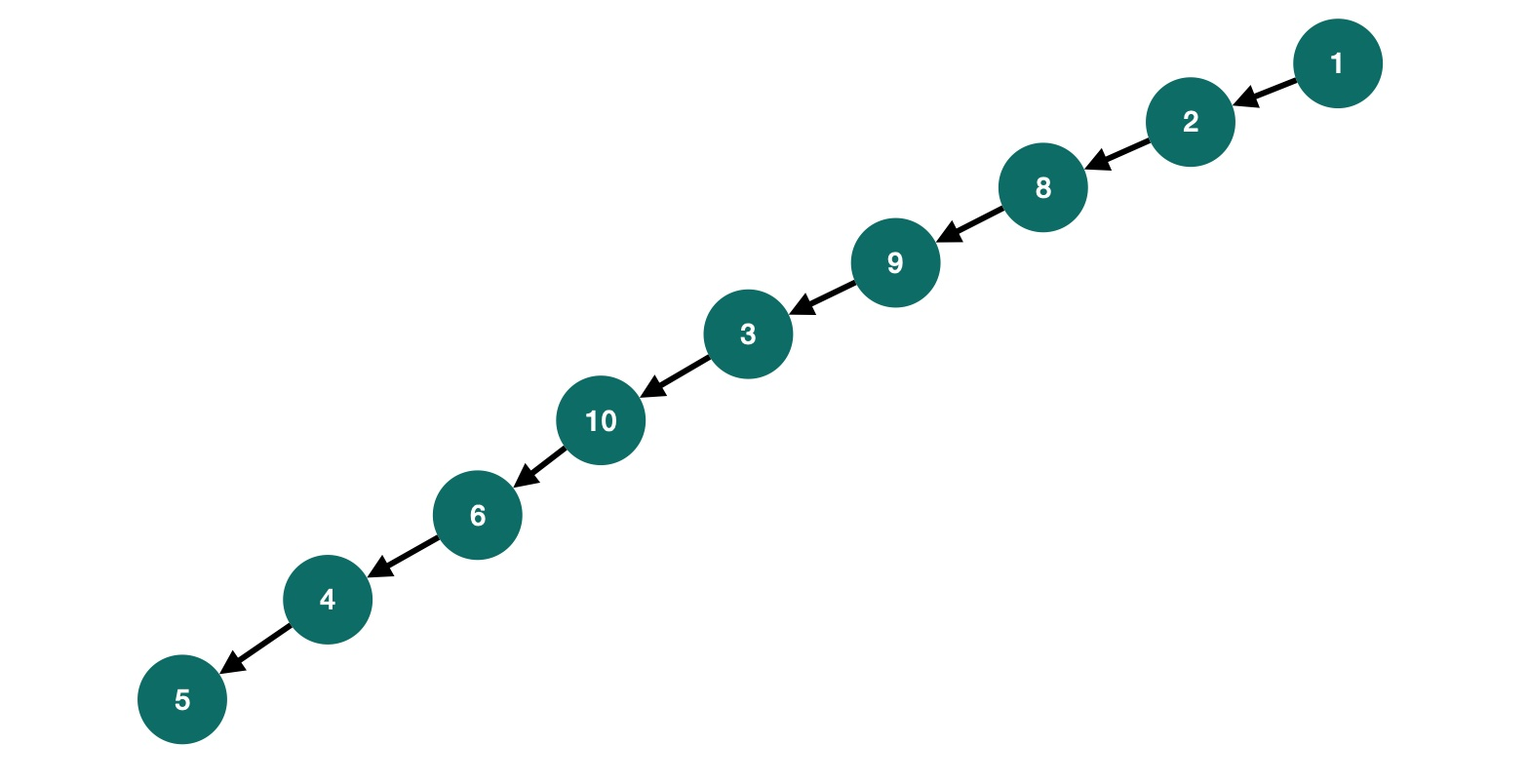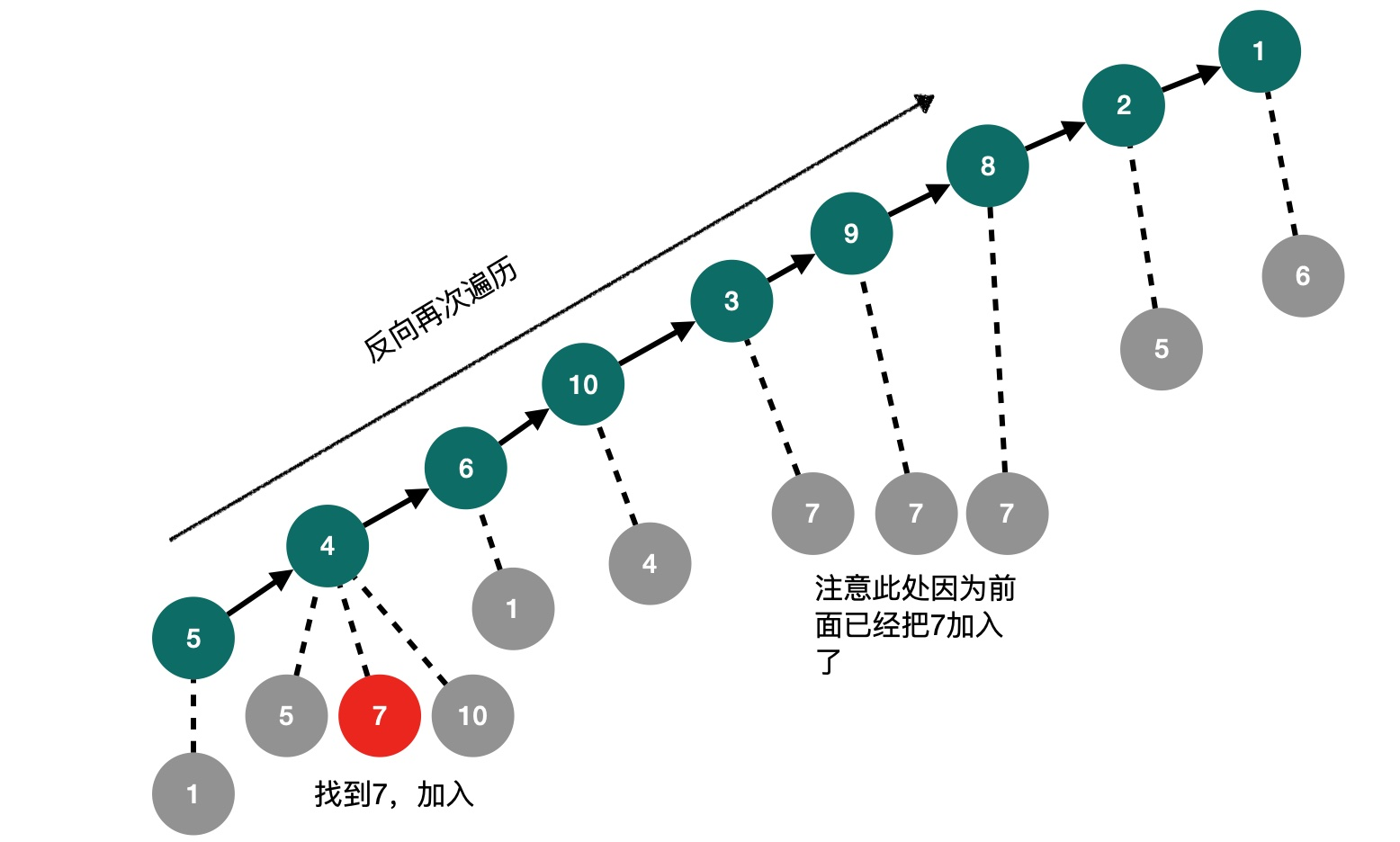### 邻接矩阵的深度优先搜索

``#define OK 1#define ERROR 0#define TRUE 1#define FALSE 0typedef int Status;    /* Status是函数的类型,其值是函数结果状态代码，如OK等 */typedef int Boolean; /* Boolean是布尔类型,其值是TRUE或FALSE */typedef char VertexType; /* 顶点类型应由用户定义 */typedef int EdgeType; /* 边上的权值类型应由用户定义 */#define MAXSIZE 9 /* 存储空间初始分配量 */#define MAXEDGE 15#define MAXVEX 9#define INFINITYC 65535typedef struct{    VertexType vexs[MAXVEX]; /* 顶点表 */    EdgeType arc[MAXVEX][MAXVEX];/* 邻接矩阵，可看作边表 */    int numVertexes, numEdges; /* 图中当前的顶点数和边数 */}MGraph;/*4.1 构建一个邻接矩阵*/void CreateMGraph(MGraph *G){    int i, j;    //1. 确定图的顶点数以及边数    G->numEdges=15;    G->numVertexes=9;    /*2.读入顶点信息，建立顶点表 */    G->vexs='A';    G->vexs='B';    G->vexs='C';    G->vexs='D';    G->vexs='E';    G->vexs='F';    G->vexs='G';    G->vexs='H';    G->vexs='I';    /*3. 初始化图中的边表*/    for (i = 0; i < G->numVertexes; i++)    {        for ( j = 0; j < G->numVertexes; j++)        {            G->arc[i][j]=0;        }    }    /*4.将图中的连接信息输入到边表中*/    G->arc=1;    G->arc=1;    G->arc=1;    G->arc=1;    G->arc=1;    G->arc=1;    G->arc=1;    G->arc=1;    G->arc=1;    G->arc=1;    G->arc=1;    G->arc=1;    G->arc=1;    G->arc=1;    G->arc=1;    /*5.无向图是对称矩阵.构成对称*/    for(i = 0; i < G->numVertexes; i++)    {        for(j = i; j < G->numVertexes; j++)        {            G->arc[j][i] =G->arc[i][j];        }    }}/*4.2 DFS遍历*/Boolean visited[MAXVEX]; /* 访问标志的数组 *///1. 标识顶点是否被标记过;//2. 选择从某一个顶点开始(注意:非连通图的情况)//3. 进入递归,打印i点信息,标识; 边表//4. [i][j] 是否等于1,没有变遍历过vistedvoid DFS(MGraph G,int i){    //1.    visited[i] = TRUE;    printf("%c",G.vexs[i]);    //2.0~numVertexes    for(int j = 0; j < G.numVertexes;j++){        if(G.arc[i][j] == 1 && !visited[j])            DFS(G, j);    }}void DFSTravese(MGraph G){    //1.初始化    for(int i=0;i<G.numVertexes;i++){        visited[i] = FALSE;    }    //2.某一个顶点    for(int i = 0;i<G.numVertexes;i++){        if(!visited[i]){            DFS(G, i);        }    }}int main(int argc, const char * argv[]) {    // insert code here...    printf("邻接矩阵的深度优先遍历!\n");    MGraph G;    CreateMGraph(&G);    DFSTravese(G);    printf("\n");    return 0;}``

### 邻接表的深度优先搜索

``#define OK 1#define ERROR 0#define TRUE 1#define FALSE 0#define MAXSIZE 9 /* 存储空间初始分配量 */#define MAXEDGE 15#define MAXVEX 9#define INFINITYC 65535typedef int Status;    /* Status是函数的类型,其值是函数结果状态代码,如OK等 */typedef int Boolean; /* Boolean是布尔类型,其值是TRUE或FALSE */typedef char VertexType; /* 顶点类型应由用户定义 */typedef int EdgeType; /* 边上的权值类型应由用户定义 *//* 邻接矩阵结构 */typedef struct{    VertexType vexs[MAXVEX]; /* 顶点表 */    EdgeType arc[MAXVEX][MAXVEX];/* 邻接矩阵,可看作边表 */    int numVertexes, numEdges; /* 图中当前的顶点数和边数 */}MGraph;/* 邻接表结构****************** */typedef struct EdgeNode /* 边表结点 */{    int adjvex;    /* 邻接点域,存储该顶点对应的下标 */    int weight;        /* 用于存储权值,对于非网图可以不需要 */    struct EdgeNode *next; /* 链域,指向下一个邻接点 */}EdgeNode;typedef struct VertexNode /* 顶点表结点 */{    int in;    /* 顶点入度 */    char data; /* 顶点域,存储顶点信息 */    EdgeNode *firstedge;/* 边表头指针 */}VertexNode, AdjList[MAXVEX];typedef struct{    AdjList adjList;    int numVertexes,numEdges; /* 图中当前顶点数和边数 */}graphAdjList,*GraphAdjList;/*4.1 构建一个邻接矩阵*/void CreateMGraph(MGraph *G){    int i, j;    //1. 确定图的顶点数以及边数    G->numEdges=15;    G->numVertexes=9;    /*2.读入顶点信息，建立顶点表 */    G->vexs='A';    G->vexs='B';    G->vexs='C';    G->vexs='D';    G->vexs='E';    G->vexs='F';    G->vexs='G';    G->vexs='H';    G->vexs='I';    /*3. 初始化图中的边表*/    for (i = 0; i < G->numVertexes; i++)    {        for ( j = 0; j < G->numVertexes; j++)        {            G->arc[i][j]=0;        }    }    /*4.将图中的连接信息输入到边表中*/    G->arc=1;    G->arc=1;    G->arc=1;    G->arc=1;    G->arc=1;    G->arc=1;    G->arc=1;    G->arc=1;    G->arc=1;    G->arc=1;    G->arc=1;    G->arc=1;    G->arc=1;    G->arc=1;    G->arc=1;    /*5.无向图是对称矩阵.构成对称*/    for(i = 0; i < G->numVertexes; i++)    {        for(j = i; j < G->numVertexes; j++)        {            G->arc[j][i] =G->arc[i][j];        }    }}/*4.2 利用邻接矩阵构建邻接表*/void CreateALGraph(MGraph G,GraphAdjList *GL){    //1.创建邻接表,并且设计邻接表的顶点数以及弧数    *GL = (GraphAdjList)malloc(sizeof(graphAdjList));    (*GL)->numVertexes = G.numVertexes;    (*GL)->numEdges = G.numEdges;    //2. 从邻接矩阵中将顶点信息输入    for (int i = 0; i < G.numVertexes; i++) {        //顶点入度为0        (*GL)->adjList[i].in = 0;        //顶点信息        (*GL)->adjList[i].data = G.vexs[i];        //顶点边表置空        (*GL)->adjList[i].firstedge = NULL;    }    //3. 建立边表    EdgeNode *e;    for (int i = 0; i < G.numVertexes; i++) {        for (int j = 0; j < G.numVertexes; j++) {            if (G.arc[i][j] == 1) {                //创建边表中的邻近结点 i->j                e = (EdgeNode *)malloc(sizeof(EdgeNode));                //邻接序号为j                e->adjvex = j;                //将当前结点的指向adjList[i]的顶点边表上                e->next = (*GL)->adjList[i].firstedge;                (*GL)->adjList[i].firstedge = e;                //顶点j 上的入度++;                (*GL)->adjList[j].in++;//                //创建边表中的邻近结点 j->i//                e = (EdgeNode *)malloc(sizeof(EdgeNode));//                //邻接序号为j//                e->adjvex = i;//                //将当前结点的指向adjList[i]的顶点边表上//                e->next = (*GL)->adjList[j].firstedge;//                (*GL)->adjList[j].firstedge = e;//                //顶点j 上的入度++;//                (*GL)->adjList[i].in++;            }        }    }}Boolean visited[MAXSIZE]; /* 访问标志的数组 *//* 邻接表的深度优先递归算法 */void DFS(GraphAdjList GL, int i){    EdgeNode *p;    visited[i] = TRUE;    //2.打印顶点 A    printf("%c ",GL->adjList[i].data);    p = GL->adjList[i].firstedge;    //3.    while (p) {        if(!visited[p->adjvex])            DFS(GL,p->adjvex);        p = p->next;    }}/* 邻接表的深度遍历操作 */void DFSTraverse(GraphAdjList GL){    //1. 将访问记录数组默认置为FALSE    for (int i = 0; i < GL->numVertexes; i++) {        /*初始化所有顶点状态都是未访问过的状态*/        visited[i] = FALSE;    }    //2. 选择一个顶点开始DFS遍历. 例如A    for(int i = 0; i < GL->numVertexes; i++)        //对未访问过的顶点调用DFS, 若是连通图则只会执行一次.        if(!visited[i])            DFS(GL, i);}int main(int argc, const char * argv[]) {    // insert code here...    printf("邻接表的深度优先遍历!\n");    MGraph G;    GraphAdjList GL;    CreateMGraph(&G);    CreateALGraph(G,&GL);    DFSTraverse(GL);    printf("\n");    return 0;}``

## 广度优先搜索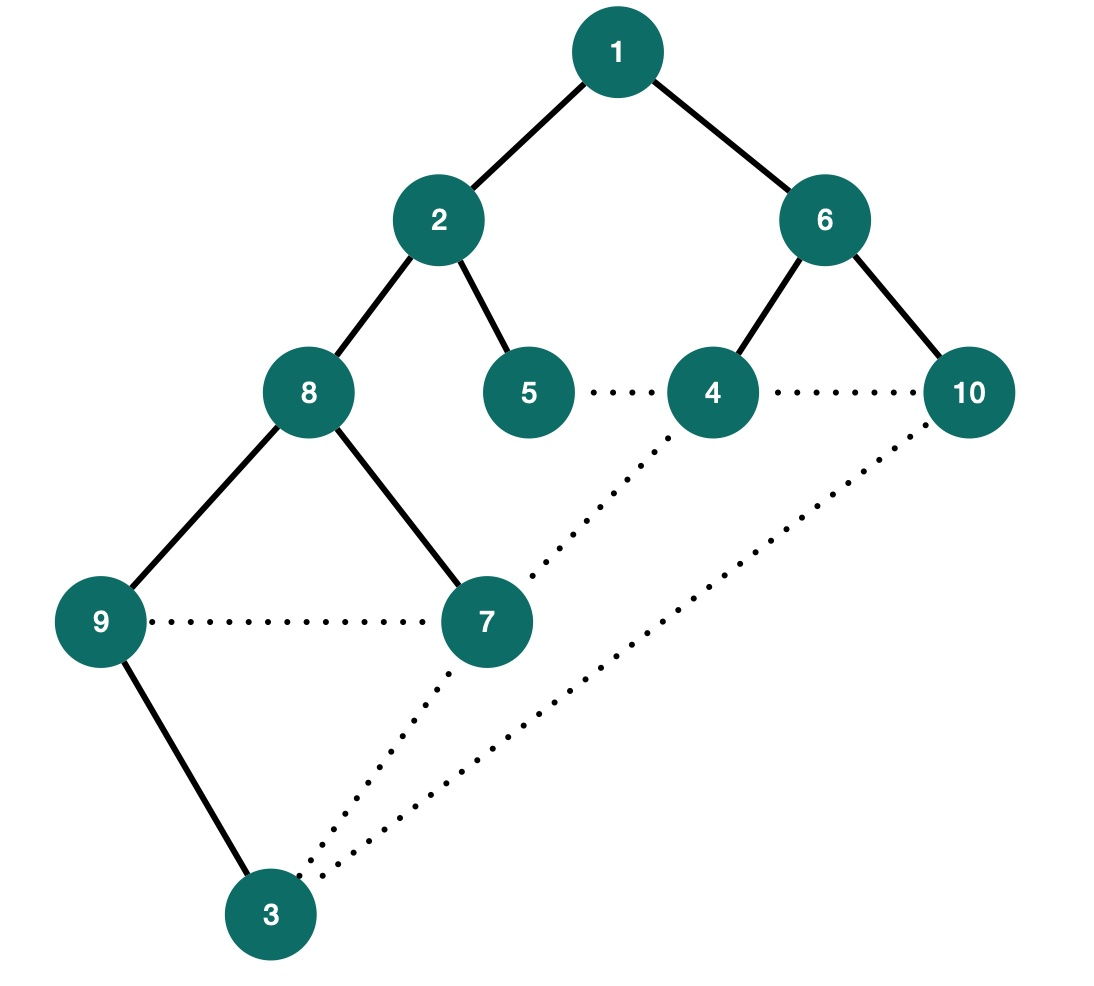### 邻接矩阵的广度优先搜索

``#define OK 1#define ERROR 0#define TRUE 1#define FALSE 0typedef int Status;    /* Status是函数的类型,其值是函数结果状态代码，如OK等 */typedef int Boolean; /* Boolean是布尔类型,其值是TRUE或FALSE */typedef char VertexType; /* 顶点类型应由用户定义 */typedef int EdgeType; /* 边上的权值类型应由用户定义 */#define MAXSIZE 9 /* 存储空间初始分配量 */#define MAXEDGE 15#define MAXVEX 9#define INFINITYC 65535typedef struct{    VertexType vexs[MAXVEX]; /* 顶点表 */    EdgeType arc[MAXVEX][MAXVEX];/* 邻接矩阵，可看作边表 */    int numVertexes, numEdges; /* 图中当前的顶点数和边数 */}MGraph;/*4.1 构建一个邻接矩阵*/void CreateMGraph(MGraph *G){    int i, j;    //1. 确定图的顶点数以及边数    G->numEdges=15;    G->numVertexes=9;    /*2.读入顶点信息，建立顶点表 */    G->vexs='A';    G->vexs='B';    G->vexs='C';    G->vexs='D';    G->vexs='E';    G->vexs='F';    G->vexs='G';    G->vexs='H';    G->vexs='I';    /*3. 初始化图中的边表*/    for (i = 0; i < G->numVertexes; i++)    {        for ( j = 0; j < G->numVertexes; j++)        {            G->arc[i][j]=0;        }    }    /*4.将图中的连接信息输入到边表中*/    G->arc=1;    G->arc=1;    G->arc=1;    G->arc=1;    G->arc=1;    G->arc=1;    G->arc=1;    G->arc=1;    G->arc=1;    G->arc=1;    G->arc=1;    G->arc=1;    G->arc=1;    G->arc=1;    G->arc=1;    /*5.无向图是对称矩阵.构成对称*/    for(i = 0; i < G->numVertexes; i++)    {        for(j = i; j < G->numVertexes; j++)        {            G->arc[j][i] =G->arc[i][j];        }    }}/* 4.2 ***需要用到的队列结构与相关功能函数*** *//* 循环队列的顺序存储结构 */typedef struct{    int data[MAXSIZE];    int front;        /* 头指针 */    int rear;        /* 尾指针，若队列不空，指向队列尾元素的下一个位置 */}Queue;/* 初始化一个空队列Q */Status InitQueue(Queue *Q){    Q->front=0;    Q->rear=0;    return  OK;}/* 若队列Q为空队列,则返回TRUE,否则返回FALSE */Status QueueEmpty(Queue Q){    if(Q.front==Q.rear) /* 队列空的标志 */    return TRUE;    else    return FALSE;}/* 若队列未满，则插入元素e为Q新的队尾元素 */Status EnQueue(Queue *Q,int e){    if ((Q->rear+1)%MAXSIZE == Q->front)    /* 队列满的判断 */    return ERROR;    Q->data[Q->rear]=e;            /* 将元素e赋值给队尾 */    Q->rear=(Q->rear+1)%MAXSIZE;/* rear指针向后移一位置， */    /* 若到最后则转到数组头部 */    return  OK;}/* 若队列不空，则删除Q中队头元素，用e返回其值 */Status DeQueue(Queue *Q,int *e){    if (Q->front == Q->rear)            /* 队列空的判断 */    return ERROR;    *e=Q->data[Q->front];                /* 将队头元素赋值给e */    Q->front=(Q->front+1)%MAXSIZE;    /* front指针向后移一位置， */    /* 若到最后则转到数组头部 */    return  OK;}/******** Queue End **************//*4.3 邻接矩阵广度优先遍历-代码实现*/Boolean visited[MAXVEX]; /* 访问标志的数组 */void BFSTraverse(MGraph G){    int temp = 0;    //1.    Queue Q;    InitQueue(&Q);    //2.将访问标志数组全部置为"未访问状态FALSE"    for (int i = 0 ; i < G.numVertexes; i++) {        visited[i] = FALSE;    }    //3.对遍历邻接表中的每一个顶点(对于连通图只会执行1次,这个循环是针对非连通图)    for (int i = 0 ; i < G.numVertexes; i++) {        if(!visited[i]){            visited[i] = TRUE;            printf("%c  ",G.vexs[i]);            //4. 入队            EnQueue(&Q, i);            while (!QueueEmpty(Q)) {                //出队                DeQueue(&Q, &i);                for (int j = 0; j < G.numVertexes; j++) {                    if(G.arc[i][j] == 1 && !visited[j])                    {    visited[j] = TRUE;                        printf("%c   ",G.vexs[j]);                        EnQueue(&Q, j);                    }                }            }        }    }}int main(int argc, const char * argv[]) {    // insert code here...    printf("邻接矩阵广度优先遍历!\n");    MGraph G;    CreateMGraph(&G);    BFSTraverse(G);    printf("\n");    return 0;}``

### 邻接表的广度优先搜索

``#define OK 1#define ERROR 0#define TRUE 1#define FALSE 0#define MAXSIZE 9 /* 存储空间初始分配量 */#define MAXEDGE 15#define MAXVEX 9#define INFINITYC 65535typedef int Status;    /* Status是函数的类型,其值是函数结果状态代码,如OK等 */typedef int Boolean; /* Boolean是布尔类型,其值是TRUE或FALSE */typedef char VertexType; /* 顶点类型应由用户定义 */typedef int EdgeType; /* 边上的权值类型应由用户定义 *//* 邻接矩阵结构 */typedef struct{    VertexType vexs[MAXVEX]; /* 顶点表 */    EdgeType arc[MAXVEX][MAXVEX];/* 邻接矩阵,可看作边表 */    int numVertexes, numEdges; /* 图中当前的顶点数和边数 */}MGraph;/* 邻接表结构****************** */typedef struct EdgeNode /* 边表结点 */{    int adjvex;    /* 邻接点域,存储该顶点对应的下标 */    int weight;        /* 用于存储权值,对于非网图可以不需要 */    struct EdgeNode *next; /* 链域,指向下一个邻接点 */}EdgeNode;typedef struct VertexNode /* 顶点表结点 */{    int in;    /* 顶点入度 */    char data; /* 顶点域,存储顶点信息 */    EdgeNode *firstedge;/* 边表头指针 */}VertexNode, AdjList[MAXVEX];typedef struct{    AdjList adjList;    int numVertexes,numEdges; /* 图中当前顶点数和边数 */}graphAdjList,*GraphAdjList;/*4.1 构建一个邻接矩阵*/void CreateMGraph(MGraph *G){    int i, j;    //1. 确定图的顶点数以及边数    G->numEdges=15;    G->numVertexes=9;    /*2.读入顶点信息，建立顶点表 */    G->vexs='A';    G->vexs='B';    G->vexs='C';    G->vexs='D';    G->vexs='E';    G->vexs='F';    G->vexs='G';    G->vexs='H';    G->vexs='I';    /*3. 初始化图中的边表*/    for (i = 0; i < G->numVertexes; i++)    {        for ( j = 0; j < G->numVertexes; j++)        {            G->arc[i][j]=0;        }    }    /*4.将图中的连接信息输入到边表中*/    G->arc=1;    G->arc=1;    G->arc=1;    G->arc=1;    G->arc=1;    G->arc=1;    G->arc=1;    G->arc=1;    G->arc=1;    G->arc=1;    G->arc=1;    G->arc=1;    G->arc=1;    G->arc=1;    G->arc=1;    /*5.无向图是对称矩阵.构成对称*/    for(i = 0; i < G->numVertexes; i++)    {        for(j = i; j < G->numVertexes; j++)        {            G->arc[j][i] =G->arc[i][j];        }    }}/*4.2 利用邻接矩阵构建邻接表*/void CreateALGraph(MGraph G,GraphAdjList *GL){    //1.创建邻接表,并且设计邻接表的顶点数以及弧数    *GL = (GraphAdjList)malloc(sizeof(graphAdjList));    (*GL)->numVertexes = G.numVertexes;    (*GL)->numEdges = G.numEdges;    //2. 从邻接矩阵中将顶点信息输入    for (int i = 0; i < G.numVertexes; i++) {        //顶点入度为0        (*GL)->adjList[i].in = 0;        //顶点信息        (*GL)->adjList[i].data = G.vexs[i];        //顶点边表置空        (*GL)->adjList[i].firstedge = NULL;    }    //3. 建立边表    EdgeNode *e;    for (int i = 0; i < G.numVertexes; i++) {        for (int j = 0; j < G.numVertexes; j++) {            if (G.arc[i][j] == 1) {                //创建边表中的邻近结点 i->j                e = (EdgeNode *)malloc(sizeof(EdgeNode));                //邻接序号为j                e->adjvex = j;                //将当前结点的指向adjList[i]的顶点边表上                e->next = (*GL)->adjList[i].firstedge;                (*GL)->adjList[i].firstedge = e;                //顶点j 上的入度++;                (*GL)->adjList[j].in++;                //                //创建边表中的邻近结点 j->i                //                e = (EdgeNode *)malloc(sizeof(EdgeNode));                //                //邻接序号为j                //                e->adjvex = i;                //                //将当前结点的指向adjList[i]的顶点边表上                //                e->next = (*GL)->adjList[j].firstedge;                //                (*GL)->adjList[j].firstedge = e;                //                //顶点j 上的入度++;                //                (*GL)->adjList[i].in++;            }        }    }}/* 5.2 ***需要用到的队列结构与相关功能函数*** *//* 循环队列的顺序存储结构 */typedef struct{    int data[MAXSIZE];    int front;        /* 头指针 */    int rear;        /* 尾指针,若队列不空,指向队列尾元素的下一个位置 */}Queue;/* 初始化一个空队列Q */Status InitQueue(Queue *Q){    Q->front=0;    Q->rear=0;    return  OK;}/* 若队列Q为空队列,则返回TRUE,否则返回FALSE */Status QueueEmpty(Queue Q){    if(Q.front==Q.rear) /* 队列空的标志 */    return TRUE;    else    return FALSE;}/* 若队列未满,则插入元素e为Q新的队尾元素 */Status EnQueue(Queue *Q,int e){    if ((Q->rear+1)%MAXSIZE == Q->front)    /* 队列满的判断 */    return ERROR;    Q->data[Q->rear]=e;            /* 将元素e赋值给队尾 */    Q->rear=(Q->rear+1)%MAXSIZE;/* rear指针向后移一位置, */    /* 若到最后则转到数组头部 */    return  OK;}/* 若队列不空,则删除Q中队头元素,用e返回其值 */Status DeQueue(Queue *Q,int *e){    if (Q->front == Q->rear)            /* 队列空的判断 */    return ERROR;    *e=Q->data[Q->front];                /* 将队头元素赋值给e */    Q->front=(Q->front+1)%MAXSIZE;    /* front指针向后移一位置, */    /* 若到最后则转到数组头部 */    return  OK;}/* *********************** Queue End ******************************* *//*5.3 邻接表广度优先遍历*/Boolean visited[MAXSIZE]; /* 访问标志的数组 */void BFSTraverse(GraphAdjList GL){    //1.创建结点    EdgeNode *p;    Queue Q;    InitQueue(&Q);    //2.将访问标志数组全部置为"未访问状态FALSE"    for(int i = 0; i < GL->numVertexes; i++)        visited[i] = FALSE;    //3.对遍历邻接表中的每一个顶点(对于连通图只会执行1次,这个循环是针对非连通图)    for(int i = 0 ;i < GL->numVertexes;i++){        //4.判断当前结点是否被访问过.        if(!visited[i]){            visited[i] = TRUE;            //打印顶点            printf("%c ",GL->adjList[i].data);            EnQueue(&Q, i);            while (!QueueEmpty(Q)) {                DeQueue(&Q, &i);                p = GL->adjList[i].firstedge;                while (p) {                    //判断                    if(!visited[p->adjvex]){                        visited[p->adjvex] = TRUE;                         printf("%c ",GL->adjList[p->adjvex].data);                        EnQueue(&Q, p->adjvex);                    }                    p = p->next;                }            }        }    }}int main(int argc, const char * argv[]) {    // insert code here...    printf("邻接表广度优先遍历\n");    MGraph G;    GraphAdjList GL;    CreateMGraph(&G);    CreateALGraph(G,&GL);    BFSTraverse(GL);    printf("\n");    return 0;}``

]]>

## 哈夫曼（David Huffman）PS：貌似人家不是程序员，人家是科学家好吧….

## 哈夫曼编码背景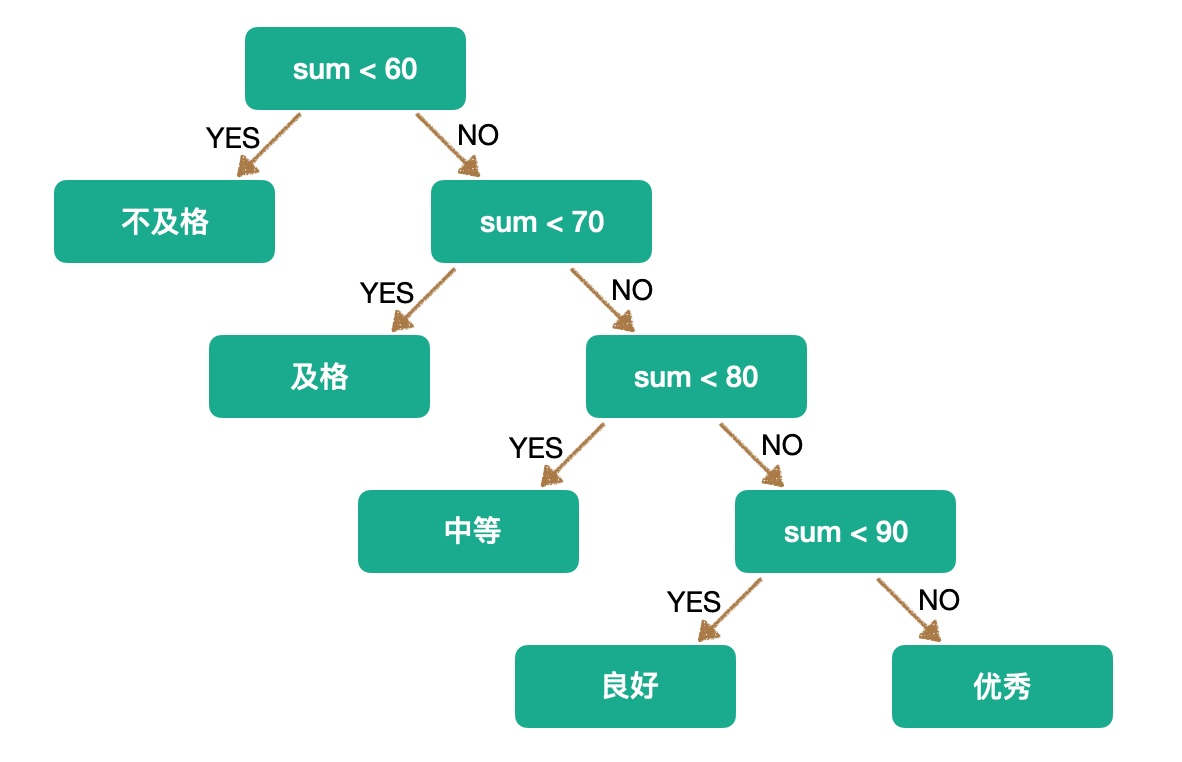## 哈夫曼思考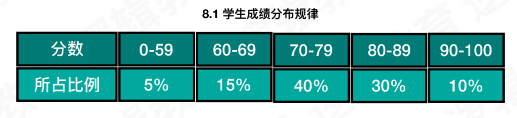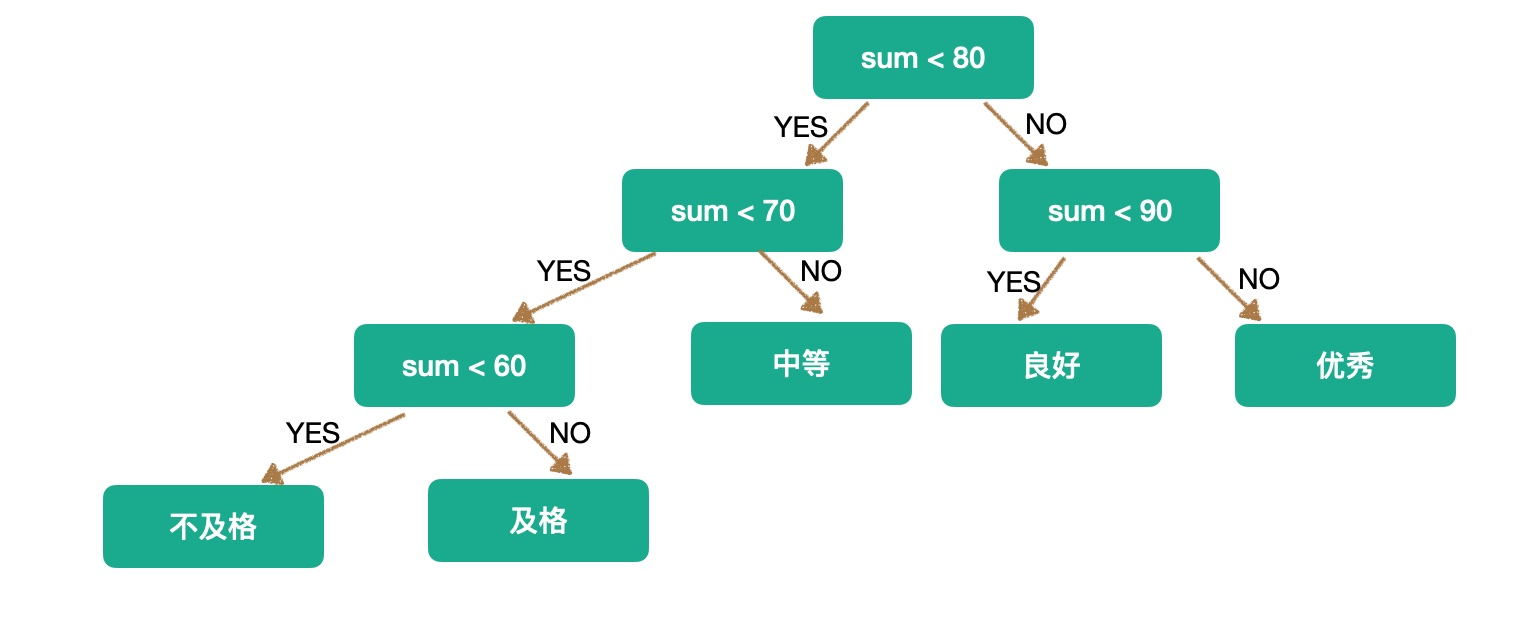## 哈弗曼树的构建

1. 首先找到目前权值最小的两个子几点，构成一个二叉树，如下：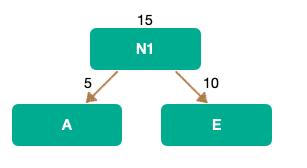1. 由于A和E生成一个二叉树，其根节点的权值就会变成 A+E = 15 ，所以接下来 我们就要从 N1,B,D,C 中找到最小的两个，PS：其原理就是每次都要找最小的两个权值生成树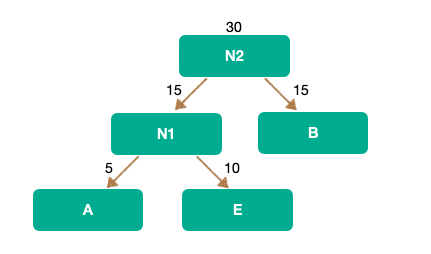1. 以此类推，最终这个哈弗曼树应该是这样：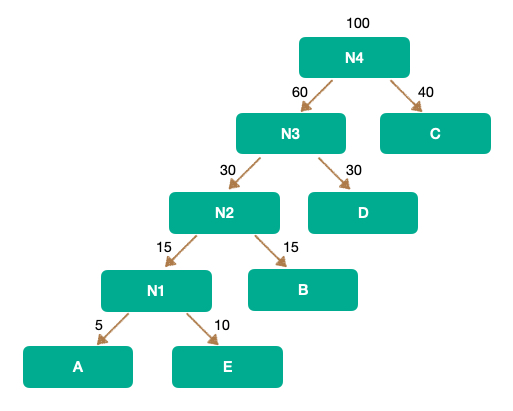## 代码

``const int MaxValue = 10000;//初始设定的权值最大值const int MaxBit = 4;//初始设定的最大编码位数const int MaxN = 10;//初始设定的最大结点个数typedef struct HaffNode{    int weight;    int flag;    int parent;    int leftChild;    int rightChild;}HaffNode;typedef struct Code//存放哈夫曼编码的数据元素结构{    int bit[MaxBit];//数组    int start;  //编码的起始下标    int weight;//字符的权值}Code;//1.//根据权重值,构建哈夫曼树;//{2,4,5,7}//n = 4;void Haffman(int weight[],int n,HaffNode *haffTree){    int j,m1,m2,x1,x2;    //1.哈夫曼树初始化    //n个叶子结点. 2n-1    for(int i = 0; i < 2*n-1;i++){        if(i<n)            haffTree[i].weight = weight[i];        else            haffTree[i].weight = 0;        haffTree[i].parent = 0;        haffTree[i].flag = 0;        haffTree[i].leftChild = -1;        haffTree[i].rightChild = -1;    }    //2.构造哈夫曼树haffTree的n-1个非叶结点    for (int i = 0; i< n - 1; i++){         m1 = m2 = MaxValue;         x1 = x2 = 0;        //2,4,5,7        for (j = 0; j< n + i; j++)//循环找出所有权重中，最小的二个值--morgan        {            if (haffTree[j].weight < m1 && haffTree[j].flag == 0)            {                m2 = m1;                x2 = x1;                m1 = haffTree[j].weight;                x1 = j;            } else if(haffTree[j].weight<m2 && haffTree[j].flag == 0)            {                m2 = haffTree[j].weight;                x2 = j;            }        }        //3.将找出的两棵权值最小的子树合并为一棵子树        haffTree[x1].parent = n + i;        haffTree[x2].parent = n + i;        //将2个结点的flag 标记为1,表示已经加入到哈夫曼树中        haffTree[x1].flag = 1;        haffTree[x2].flag = 1;        //修改n+i结点的权值        haffTree[n + i].weight = haffTree[x1].weight + haffTree[x2].weight;        //修改n+i的左右孩子的值        haffTree[n + i].leftChild = x1;        haffTree[n + i].rightChild = x2;    }}/* 9.2 哈夫曼编码 由n个结点的哈夫曼树haffTree构造哈夫曼编码haffCode //{2,4,5,7} */void HaffmanCode(HaffNode haffTree[], int n, Code haffCode[]){    //1.创建一个结点cd    Code *cd = (Code * )malloc(sizeof(Code));    int child, parent;    //2.求n个叶结点的哈夫曼编码    for (int i = 0; i<n; i++)    {        //从0开始计数        cd->start = 0;        //取得编码对应权值的字符        cd->weight = haffTree[i].weight;        //当叶子结点i 为孩子结点.        child = i;        //找到child 的双亲结点;        parent = haffTree[child].parent;        //由叶结点向上直到根结点        while (parent != 0)        {            if (haffTree[parent].leftChild == child)                cd->bit[cd->start] = 0;//左孩子结点编码0            else                cd->bit[cd->start] = 1;//右孩子结点编码1            //编码自增            cd->start++;            //当前双亲结点成为孩子结点            child = parent;            //找到双亲结点            parent = haffTree[child].parent;        }         int temp = 0;        for (int j = cd->start - 1; j >= 0; j--){            temp = cd->start-j-1;            haffCode[i].bit[temp] = cd->bit[j];        }        //把cd中的数据赋值到haffCode[i]中.        //保存好haffCode 的起始位以及权值;        haffCode[i].start = cd->start;        //保存编码对应的权值        haffCode[i].weight = cd->weight;    }}int main(int argc, const char * argv[]) {    // insert code here...    printf("Hello, 哈夫曼编码!\n");    int i, j, n = 4, m = 0;    //权值    int weight[] = {2,4,5,7};    //初始化哈夫曼树, 哈夫曼编码    HaffNode *myHaffTree = malloc(sizeof(HaffNode)*2*n-1);    Code *myHaffCode = malloc(sizeof(Code)*n);    //当前n > MaxN,表示超界. 无法处理.    if (n>MaxN)    {        printf("定义的n越界，修改MaxN!");        exit(0);    }    //1. 构建哈夫曼树    Haffman(weight, n, myHaffTree);    //2.根据哈夫曼树得到哈夫曼编码    HaffmanCode(myHaffTree, n, myHaffCode);    //3.    for (i = 0; i<n; i++)    {        printf("Weight = %d\n",myHaffCode[i].weight);        for (j = 0; j<myHaffCode[i].start; j++)            printf("%d",myHaffCode[i].bit[j]);        m = m + myHaffCode[i].weight*myHaffCode[i].start;         printf("\n");    }    printf("Huffman's WPS is:%d\n",m);    return 0;}``
]]>
<h2 id="哈夫曼（David-Huffman）"><a href="#哈夫曼（David-Huffman）" class="headerlink" title="哈夫曼（David Huffman）"></a>哈夫曼（David Huffman）</h2><blockquote> <p>著名的 <strong>哈夫曼编码</strong> 发明人 戴维·霍夫曼于1999年10月17日因癌症去世，享年74岁，他发明了著名的的 <strong>霍夫曼编码</strong>，除了霍夫曼编码以外，他还涉及出二叉最优搜索树的算法，因为其效率最高，所以被命名为霍夫曼算法，是动态规划的一个范例

## 背景

### 指针域浪费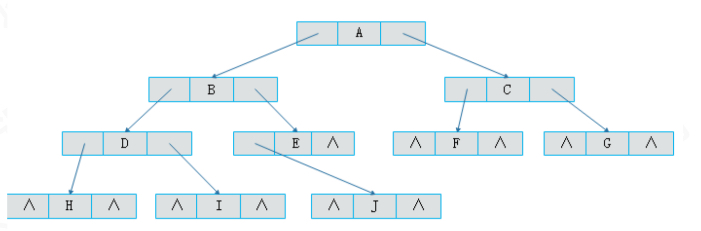### 重复查找遍历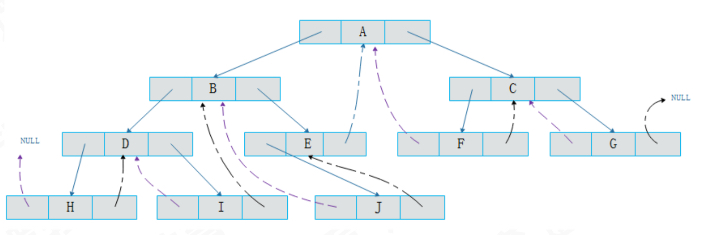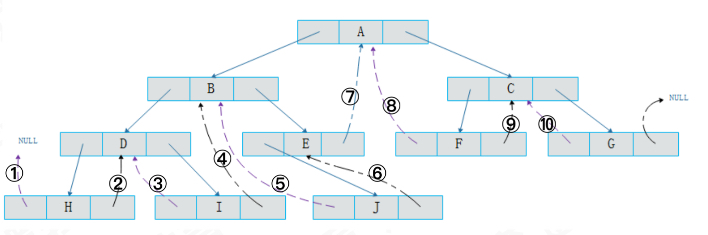### 问题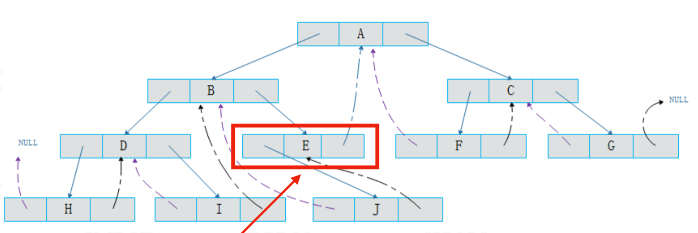## 线索二叉树的实现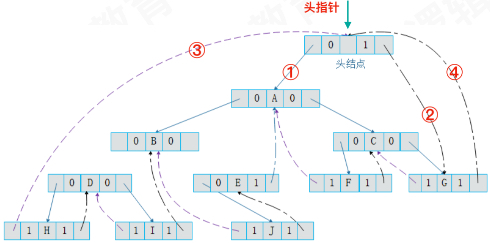``#define OK 1#define ERROR 0#define TRUE 1#define FALSE 0#define MAXSIZE 100 /* 存储空间初始分配量 *//* Status是函数的类型,其值是函数结果状态代码,如OK等 */typedef int Status;typedef char CElemType;/* 字符型以空格符为空 */CElemType Nil='#';#pragma mark--二叉树构造int indexs = 1;typedef char String; /*  0号单元存放串的长度 */String str;Status StrAssign(String T,char *chars){    int i;    if(strlen(chars)>MAXSIZE)        return ERROR;    else    {        T=strlen(chars);        for(i=1;i<=T;i++)            T[i]=*(chars+i-1);        return OK;    }}/* Link==0表示指向左右孩子指针, *//* Thread==1表示指向前驱或后继的线索 */typedef enum {Link,Thread} PointerTag;/* 线索二叉树存储结点结构*/typedef struct BiThrNode{    //数据    CElemType data;    //左右孩子指针    struct BiThrNode *lchild,*rchild;    //左右标记    PointerTag LTag;    PointerTag RTag;}BiThrNode,*BiThrTree;/* 8.1 打印 */Status visit(CElemType e){    printf("%c ",e);    return OK;}/* 8.3 构造二叉树 按照前序输入线索二叉树结点的值,构造二叉树T */Status CreateBiThrTree(BiThrTree *T){    CElemType h;    //scanf("%c",&h);    //获取字符    h = str[indexs++];    if (h == Nil) {        *T = NULL;    }else{        *T = (BiThrTree)malloc(sizeof(BiThrNode));        if (!*T) {            exit(OVERFLOW);        }        //生成根结点(前序)        (*T)->data = h;        //递归构造左子树        CreateBiThrTree(&(*T)->lchild);        //存在左孩子->将标记LTag设置为Link        if ((*T)->lchild) (*T)->LTag = Link;        //递归构造右子树        CreateBiThrTree(&(*T)->rchild);        //存在右孩子->将标记RTag设置为Link        if ((*T)->rchild) (*T)->RTag = Link;    }    return OK;}/* 8.3 中序遍历二叉树T, 将其中序线索化,Thrt指向头结点 */BiThrTree pre; /* 全局变量,始终指向刚刚访问过的结点 *//* 中序遍历进行中序线索化*/void InThreading(BiThrTree p){    /*     InThreading(p->lchild);     .....     InThreading(p->rchild);     */    if (p) {        //递归左子树线索化        InThreading(p->lchild);        //无左孩子        if (!p->lchild) {            //前驱线索            p->LTag = Thread;            //左孩子指针指向前驱            p->lchild  = pre;        }else        {            p->LTag = Link;        }        //前驱没有右孩子        if (!pre->rchild) {            //后继线索            pre->RTag = Thread;            //前驱右孩子指针指向后继(当前结点p)            pre->rchild = p;        }else        {            pre->RTag = Link;        }        //保持pre指向p的前驱        pre = p;        //递归右子树线索化        InThreading(p->rchild);    }}/* 中序遍历二叉树T,并将其中序线索化,Thrt指向头结点 */Status InOrderThreading(BiThrTree *Thrt , BiThrTree T){    *Thrt=(BiThrTree)malloc(sizeof(BiThrNode));    if (! *Thrt) {        exit(OVERFLOW);    }    //建立头结点;    (*Thrt)->LTag = Link;    (*Thrt)->RTag = Thread;    //右指针回指向    (*Thrt)->rchild = (*Thrt);    /* 若二叉树空,则左指针回指 */    if (!T) {        (*Thrt)->lchild=*Thrt;    }else{        (*Thrt)->lchild=T;        pre=(*Thrt);        //中序遍历进行中序线索化        InThreading(T);        //最后一个结点rchil 孩子        pre->rchild = *Thrt;        //最后一个结点线索化        pre->RTag = Thread;        (*Thrt)->rchild = pre;    }    return OK;}/*中序遍历二叉线索树T*/Status InOrderTraverse_Thr(BiThrTree T){    BiThrTree p;    p=T->lchild; /* p指向根结点 */    while(p!=T)    { /* 空树或遍历结束时,p==T */        while(p->LTag==Link)            p=p->lchild;        if(!visit(p->data)) /* 访问其左子树为空的结点 */            return ERROR;        while(p->RTag==Thread&&p->rchild!=T)        {            p=p->rchild;            visit(p->data); /* 访问后继结点 */        }        p=p->rchild;    }    return OK;}int main(int argc, const char * argv[]) {    // insert code here...    printf("Hello, 线索化二叉树!\n");    BiThrTree H,T;    //StrAssign(str,"ABDH#K###E##CFI###G#J##");    StrAssign(str,"ABDH##I##EJ###CF##G##");    CreateBiThrTree(&T); /* 按前序产生二叉树 */    InOrderThreading(&H,T); /* 中序遍历,并中序线索化二叉树 */    InOrderTraverse_Thr(H);    printf("\n\n");    return 0;}``
]]>
<h2 id="背景"><a href="#背景" class="headerlink" title="背景"></a>背景</h2><p>之前我们讲过二叉树这种数据结构，在他的链式存储结构中我们采用了这种数据结构：</p> <figure class="highlight c"><table><tr><td class="gutter"><pre><span class="line">1</span><br><span class="line">2</span><br><span class="line">3</span><br><span class="line">4</span><br><span class="line">5</span><br><span class="line">6</span><br></pre></td><td class="code"><pre><span class="line"><span class="keyword">typedef</span> <span class="class"><span class="keyword">struct</span> <span class="title">BiThrNode</span>&#123;</span></span><br><span class="line"> <span class="comment">//数据</span></span><br><span class="line"> CElemType data;</span><br><span class="line"> <span class="comment">//左右孩子指针</span></span><br><span class="line"> <span class="class"><span class="keyword">struct</span> <span class="title">BiThrNode</span> *<span class="title">lchild</span>,*<span class="title">rchild</span>;</span> </span><br><span class="line">&#125;BiThrNode</span><br></pre></td></tr></table></figure>

## 树结构

### 一般树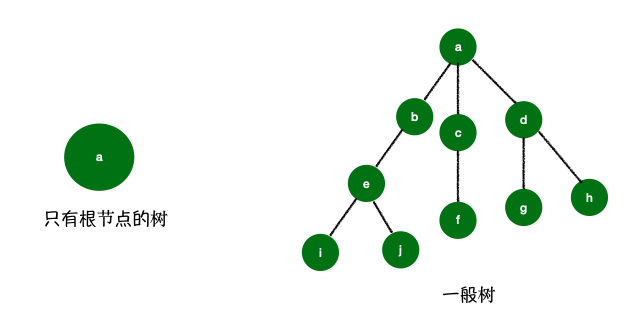### 二叉树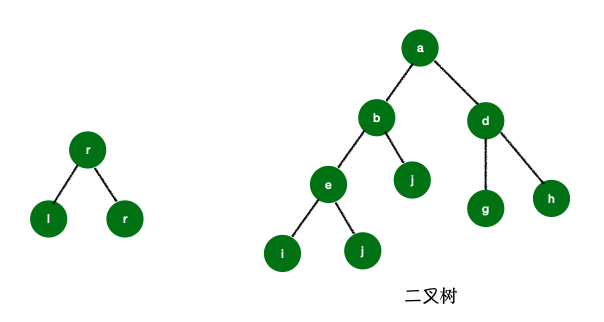#### 满二叉树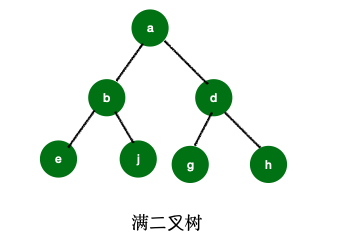#### 完全二叉树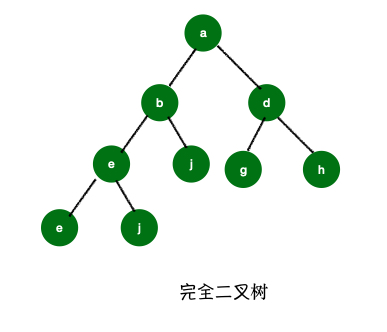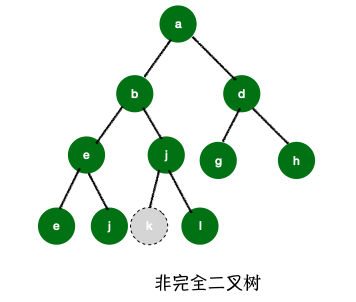## 顺序存储

``typedef struct {    int level; //结点层    int order; //本层的序号(按照满二叉树给定序号规则)}Position;#pragma mark -- 二叉树的基本操作//6.1 visitStatus visit(CElemType c){    printf("%d ",c);    return OK;}//6.2 构造空二叉树T,因为T是固定数组,不会改变.Status InitBiTree(SqBiTree T){    for (int i = 0; i < MAX_TREE_SIZE; i++) {        //将二叉树初始化值置空        T[i] = Nil;    }    return OK;}//6.3 按层序次序输入二叉树中的结点值(字符型或整型),构造顺序存储的二叉树TStatus CreateBiTree(SqBiTree T){    int i = 0;    //printf("按层序输入结点的值(整型),0表示空结点, 输入999结束.结点数<=%d\n",MAX_TREE_SIZE);    /*     1      -->1     2     3   -->2     4  5  6   7 -->3     8  9 10       -->4     1 2 3 4 5 6 7 8 9 10 Nil Nil Nil     */    while (i < 10) {        T[i] = i+1;        printf("%d ",T[i]);        //结点不为空,且无双亲结点        if (i != 0 && T[(i+1)/2-1] == Nil && T[i] != Nil) {            printf("出现无双亲的非根结点%d\n",T[i]);            exit(ERROR);        }        i++;    }    //将空赋值给T的后面的结点    while (i < MAX_TREE_SIZE) {        T[i] = Nil;        i++;    }    return OK;}//技巧://如果大家想要2个函数的结果一样,但是目的不同;//在顺序存储结构中, 两个函数完全一样的结果#define ClearBiTree InitBiTree/*6.4 判断二叉树是否为空 初始条件: 二叉树已存在 操作结果: 若T为空二叉树,则返回TRUE,否则返回FALSE; */Status BiTreeEmpty(SqBiTree T){    //根结点为空,则二叉树为空    if (T == Nil)        return TRUE;    return FALSE;}/*6.5 获取二叉树的深度 初始条件: 二叉树已存在 操作结果: 返回二叉树T深度; */int BiTreeDepth(SqBiTree T){    int j = -1;    int i;    //找到最后一个结点    //MAX_TREE_SIZE -> 100 -> 10 目的找到最后一个结点10的位置    for (i = MAX_TREE_SIZE-1 ; i>=0; i--) {        if (T[i] != Nil)            break;    }    do {        j++;    } while ( powl(2, j) <= i); //计算2的次幂    return j;}/*6.6 返回处于位置e(层,本层序号)的结点值 初始条件: 二叉树T存在,e是T中某个结点(的位置) 操作结构: 返回处于位置e(层,本层序号)的结点值 */CElemType Value(SqBiTree T,Position e){    /*     Position.level -> 结点层.表示第几层;     Position.order -> 本层的序号(按照满二叉树给定序号规则)     */    //pow(2,e.level-1) 找到层序    printf("%d\n",(int)pow(2,e.level-1));    //e.order    printf("%d\n",e.order);    //4+2-2;    return T[(int)pow(2, e.level-1)+e.order-2];}/*6.7 获取二叉树跟结点的值 初始条件: 二叉树T存在 操作结果: 当T不空,用e返回T的根, 返回OK; 否则返回ERROR */Status Root(SqBiTree T,CElemType *e){    if (BiTreeEmpty(T)) {        return ERROR;    }    *e = T;    return OK;}/* 6.8 给处于位置e的结点赋值 初始条件: 二叉树存在,e是T中某个结点的位置 操作结果: 给处于位置e的结点赋值Value; */Status Assign(SqBiTree T,Position e,CElemType value){    //找到当前e在数组中的具体位置索引    int i = (int)powl(2, e.level-1)+e.order -2;    //叶子结点的双亲为空    if (value != Nil &&  T[(i+1)/2-1] == Nil) {        return ERROR;    }    //给双亲赋空值但是有叶子结点    if (value == Nil && (T[i*2+1] != Nil || T[i*2+2] != Nil)) {        return  ERROR;    }    T[i] = value;    return OK;}/* 6.9 获取e的双亲; 初始条件: 二叉树存在,e是T中的某一个结点 操作结果: 若e是T的非根结点, 则返回它的双亲,否则返回"空" */CElemType Parent(SqBiTree T, CElemType e){    //空树    if (T == Nil) {        return Nil;    }    for (int i = 1 ; i < MAX_TREE_SIZE; i++) {        //找到e        if (T[i] == e) {            return T[(i+1)/2 - 1];        }    }    //没有找到    return Nil;}/* 6.10 获取某个结点的左孩子; 初始条件:二叉树T存在,e是某个结点 操作结果:返回e的左孩子,若e无左孩子,则返回"空" */CElemType LeftChild(SqBiTree T,CElemType e){    //空树    if (T == Nil) {        return Nil;    }    for (int i = 0 ; i < MAX_TREE_SIZE-1; i++) {        //找到e        if (T[i] == e) {            return T[i*2+1];        }    }    //没有找到    return Nil;}/* 6.11 获取某个结点的右孩子; 初始条件:二叉树T存在,e是某个结点 操作结果:返回e的左孩子,若e无左孩子,则返回"空" */CElemType RightChild(SqBiTree T,CElemType e){    //空树    if (T == Nil) {        return Nil;    }    for (int i = 0 ; i < MAX_TREE_SIZE-1; i++) {        //找到e        if (T[i] == e) {            return T[i*2+2];        }    }    //没有找到    return Nil;}/* 6.12 获取结点的左兄弟 初始条件:  二叉树T存在,e是T中某个结点 操作结果: 返回e的左兄弟。若e是T的左孩子或无左兄弟,则返回＂空＂ */CElemType LeftSibling(SqBiTree T,CElemType e){    /* 空树 */    if(T==Nil)        return Nil;    for(int i=1;i<=MAX_TREE_SIZE-1;i++)    /* 找到e且其序号为偶数(是右孩子) */        if(T[i]==e&&i%2==0)            return T[i-1];    return Nil; /* 没找到e */}/* 6.13 获取结点的右兄弟 初始条件: 二叉树T存在,e是T中某个结点 操作结果: 返回e的右兄弟。若e是T的右孩子或无右兄弟,则返回＂空＂ */CElemType RightSibling(SqBiTree T,CElemType e){    /* 空树 */    if(T==Nil)        return Nil;    for(int i=1;i<=MAX_TREE_SIZE-1;i++)    /* 找到e且其序号为奇数(是左孩子) */        if(T[i]==e&&i%2==1)            return T[i+1];    return Nil; /* 没找到e */}#pragma mark -- 二叉树的遍历/* 6.14 层序遍历二叉树 */void LevelOrderTraverse(SqBiTree T){    int i = MAX_TREE_SIZE-1;    //找到最后一个非空结点的序号    while (T[i] == Nil) i--;    //从根结点起,按层序遍历二叉树    for (int j = 0; j <= i; j++)        //只遍历非空结点        if (T[j] != Nil)            visit(T[j]);    printf("\n");}/* 6.15 前序遍历二叉树 */void PreTraverse(SqBiTree T,int e){    //打印结点数据    visit(T[e]);    //先序遍历左子树    if (T[2 * e + 1] != Nil) {        PreTraverse(T, 2*e+1);    }    //最后先序遍历右子树    if (T[2 * e + 2] != Nil) {        PreTraverse(T, 2*e+2);    }}Status PreOrderTraverse(SqBiTree T){    //树不为空    if (!BiTreeEmpty(T)) {        PreTraverse(T, 0);    }    printf("\n");    return  OK;}/* 6.16 中序遍历 */void InTraverse(SqBiTree T, int e){    /* 左子树不空 */    if (T[2*e+1] != Nil)        InTraverse(T, 2*e+1);    visit(T[e]);    /* 右子树不空 */    if (T[2*e+2] != Nil)        InTraverse(T, 2*e+2);}Status InOrderTraverse(SqBiTree T){    /* 树不空 */    if (!BiTreeEmpty(T)) {        InTraverse(T, 0);    }    printf("\n");    return OK;}/* 6.17 后序遍历 */void PostTraverse(SqBiTree T,int e){   /* 左子树不空 */    if(T[2*e+1]!=Nil)        PostTraverse(T,2*e+1);    /* 右子树不空 */    if(T[2*e+2]!=Nil)        PostTraverse(T,2*e+2);    visit(T[e]);}Status PostOrderTraverse(SqBiTree T){    if(!BiTreeEmpty(T)) /* 树不空 */        PostTraverse(T,0);    printf("\n");    return OK;}``

## 链式存储

``typedef char CElemType;CElemType Nil=' '; /* 字符型以空格符为空 */typedef struct BiTNode  /* 结点结构 */{    CElemType data;        /* 结点数据 */    struct BiTNode *lchild,*rchild; /* 左右孩子指针 */}BiTNode,*BiTree;/*7.1 打印数据*/Status visit(CElemType e){    printf("%c ",e);    return OK;}/* 7.2 构造空二叉树T */Status InitBiTree(BiTree *T){    *T=NULL;    return OK;}/* 7.3 销毁二叉树 初始条件: 二叉树T存在。 操作结果: 销毁二叉树T */void DestroyBiTree(BiTree *T){    if(*T)    {        /* 有左孩子 */        if((*T)->lchild)            DestroyBiTree(&(*T)->lchild); /* 销毁左孩子子树 */        /* 有右孩子 */        if((*T)->rchild)            DestroyBiTree(&(*T)->rchild); /* 销毁右孩子子树 */        free(*T); /* 释放根结点 */        *T=NULL; /* 空指针赋0 */    }}#define ClearBiTree DestroyBiTree/*7.4 创建二叉树 按前序输入二叉树中的结点值(字符),#表示空树; */void CreateBiTree(BiTree *T){    CElemType ch;    //获取字符    ch = str[indexs++];    //判断当前字符是否为'#'    if (ch == '#') {        *T = NULL;    }else    {        //创建新的结点        *T = (BiTree)malloc(sizeof(BiTNode));        //是否创建成功        if (!*T) {            exit(OVERFLOW);        }        /* 生成根结点 */        (*T)->data = ch;        /* 构造左子树 */        CreateBiTree(&(*T)->lchild);        /* 构造右子树 */        CreateBiTree(&(*T)->rchild);    }}/* 7.5 二叉树T是否为空; 初始条件: 二叉树T存在 操作结果: 若T为空二叉树,则返回TRUE,否则FALSE */Status BiTreeEmpty(BiTree T){    if(T)        return FALSE;    else        return TRUE;}/* 7.6 二叉树T的深度 初始条件: 二叉树T存在 操作结果: 返回T的深度 */int BiTreeDepth(BiTree T){    int i,j;    if(!T)        return 0;    //计算左孩子的深度    if(T->lchild)        i=BiTreeDepth(T->lchild);    else        i=0;    //计算右孩子的深度    if(T->rchild)        j=BiTreeDepth(T->rchild);    else        j=0;    //比较i和j    return i>j?i+1:j+1;}/* 7.7 二叉树T的根 初始条件: 二叉树T存在 操作结果: 返回T的根 */CElemType Root(BiTree T){    if (BiTreeEmpty(T))        return Nil;    return T->data;}/* 7.8 返回p所指向的结点值; 初始条件: 二叉树T存在，p指向T中某个结点 操作结果: 返回p所指结点的值 */CElemType Value(BiTree p){    return p->data;}/* 7.8 给p所指结点赋值为value; 初始条件: 二叉树T存在，p指向T中某个结点 操作结果: 给p所指结点赋值为value */void Assign(BiTree p,CElemType value){    p->data=value;}#pragma mark--二叉树遍历/* 7.8  前序递归遍历T 初始条件:二叉树T存在; 操作结果: 前序递归遍历T */void PreOrderTraverse(BiTree T){    if(T==NULL)        return;    printf("%c",T->data);/* 显示结点数据，可以更改为其它对结点操作 */    PreOrderTraverse(T->lchild); /* 再先序遍历左子树 */    PreOrderTraverse(T->rchild); /* 最后先序遍历右子树 */}/* 7.9  中序递归遍历T 初始条件:二叉树T存在; 操作结果: 中序递归遍历T */void InOrderTraverse(BiTree T){    if(T==NULL)        return ;    InOrderTraverse(T->lchild); /* 中序遍历左子树 */    printf("%c",T->data);/* 显示结点数据，可以更改为其它对结点操作 */    InOrderTraverse(T->rchild); /* 最后中序遍历右子树 */}/* 7.10  后序递归遍历T 初始条件:二叉树T存在; 操作结果: 中序递归遍历T */void PostOrderTraverse(BiTree T){    if(T==NULL)        return;    PostOrderTraverse(T->lchild); /* 先后序遍历左子树  */    PostOrderTraverse(T->rchild); /* 再后序遍历右子树  */    printf("%c",T->data);/* 显示结点数据，可以更改为其它对结点操作 */}``

## 总结

]]>
<p><strong>树</strong> 的结构在日常生活中我们有涉及到：比如我们的 <strong>族谱</strong>、<strong>公司的架构图</strong>等等，今天我们就具体看看树到底是一个什么样的数据结构，它有哪些分类以及如何去实现树。

## BF算法

BF算法 Brute Force，其实就是暴力算法，是普通的模式匹配算法，BF算法的思想就是将目标串S的第一个字符与模式串T的第一个字符进行匹配，弱相等，则继续比较S的第二个字符；若不相等，则比较S的第二个字符和T的第一个字符，一次比较下去，直到最后的的匹配结果。

``// BF算法int indexOf(char *S,char *T) {    int i = 0,j = 0;    int lenS = (int)strlen(S);    int lenT = (int)strlen(T);    while (i < lenS && j < lenT ) {        // 比较两个字母若相同则继续比较        if (S[i] == T[j]) {            i++;            j++;        } else {            // 找到上一次匹配的首位的下一位            i = i-j+1;            // j返回到子串T首位            j = 0;        }    }    if (j == lenT) {        // 这里+1是为了将index转化为现实生活中的位置        return i-lenT+1;    } else {        return -1;    }}int main(int argc, const char * argv[]) {    // insert code here...    printf("Hello, World!\n");    int index = indexOf("abcdex", "ex");    printf("第一个匹配的位置是 %d \n",index);    return 0;}-----Hello, World!第一个匹配的位置是 5 ``

## RK算法

RK算法 是由 Rabin 和 Karp 共同提出的一个算法，RK算法是对BF算法的一个改进，只进行一次比较就判定两者是否相等。RK的大体思想跟BF一样，把主串拆分成若干个子串，循环遍历每一个子串和目标串T进行比对，但是其利用了Hash算法的设计思想，每个子串都对应唯一一个哈希值，我们设计一个尽量不冲突的哈希算法来求每个子串的哈希值，一一匹配直到相等即可。

### 哈希函数设计

RK 算法中还用到一个技巧就是，只要算出第一个数字，后面的数字就可以根据第一个数字一一计算出，具体我们以十进制为例

``#define d  26 // 进制数// 即使我们求出了结果，但是为了验证是否冲突加一个验证int isMatch(char *S, int i, char *P, int m){    int is, ip;    for(is=i, ip=0; is != m && ip != m; is++, ip++)        if(S[is] != P[ip])            return 0;    return 1;}// 计算最高位的幂次方 比如三位子串就是26的平方int getMaxValue(int Tlength) {    int value = 1;    for (int i = 0; i < Tlength-1; i ++) {        value *= d;    }    return value;}// RK算法int RK(char *S, char *T) {    int slen = (int)strlen(S);    int tlen = (int)strlen(T);    int hashS = 0;    int hashT = 0;    // 分别保存两个字符串的首个哈希值    for (int i = 0; i < tlen; i++) {        hashS = hashS*d +(S[i]-'a');        hashT = hashT*d +(T[i]-'a');    }    int hValue = getMaxValue(tlen);    for (int i = 0; i <= slen-tlen; i++) {        // 如果哈希值相等就是找到了        if (hashS == hashT) {            // 验证是否真的相同            if (isMatch(S, i, T, tlen)) {                return i+1;            }        }        // 更新hashS        hashS = (hashS- (S[i]-'a')*hValue)*d + (S[i+tlen]-'a');    }    return -1;}int main(int argc, const char * argv[]) {    // insert code here...    printf("Hello, World!\n");    int index = RK("abcdex", "ex");    printf("第一个匹配的位置是 %d \n",index);    return 0;}-----Hello, World!第一个匹配的位置是 5 ``

## KMP 算法

D.E.KnuthJ.H.MorrisV.R.Pratt 共同发表模式匹配算法，称之为 克鲁特-莫里斯-普拉特算法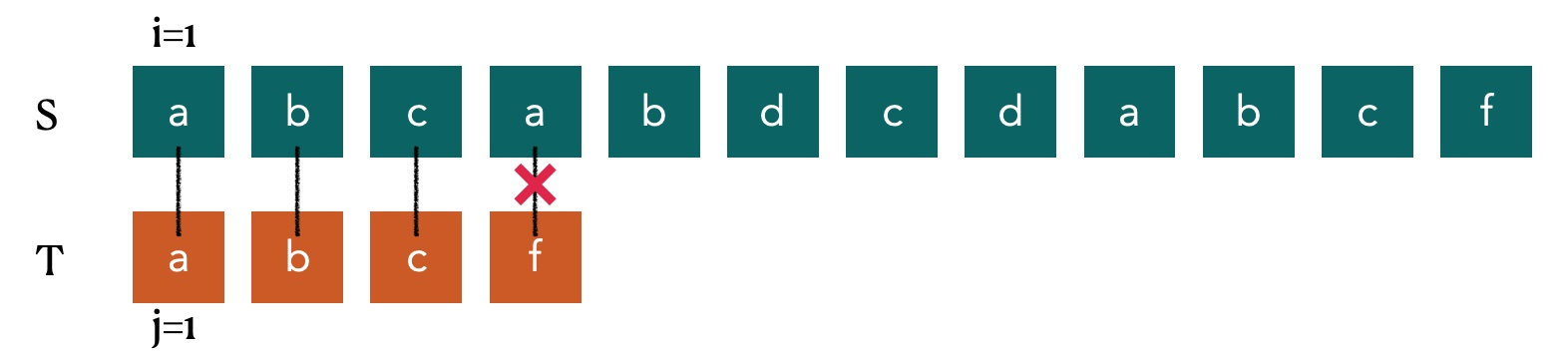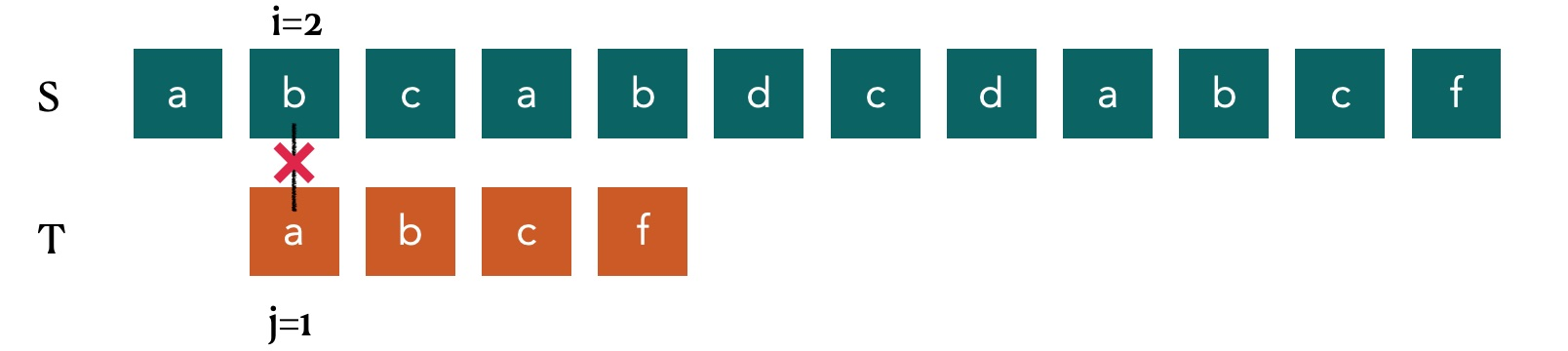`i=2`,`j=1` 发现他们的值不同，来到第三步：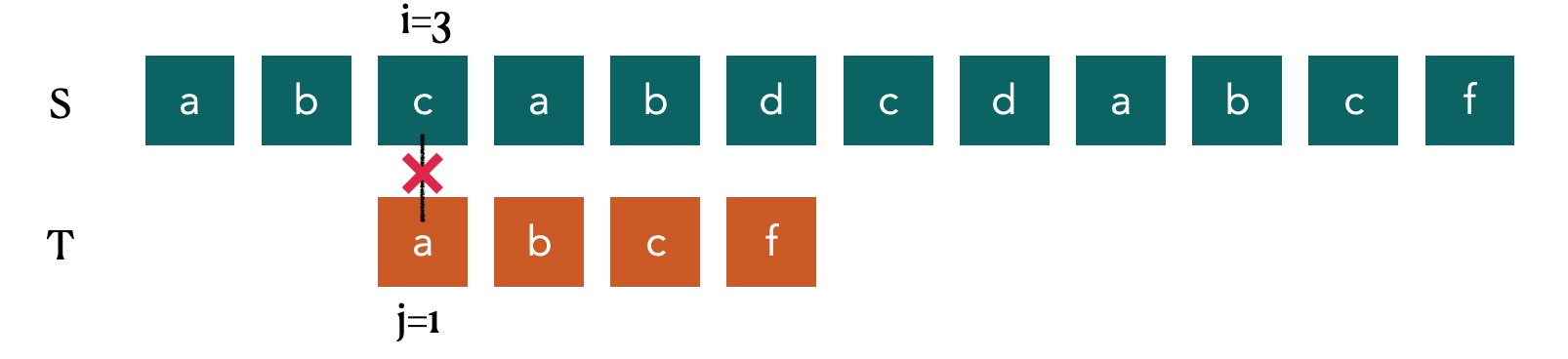`i=3`,`j=1` 发现他们的值不同，来到第四步：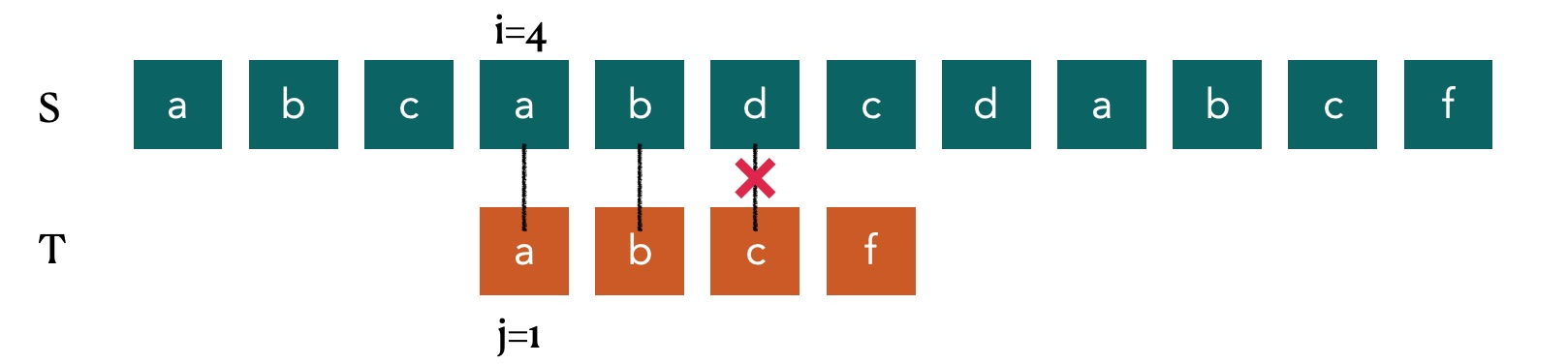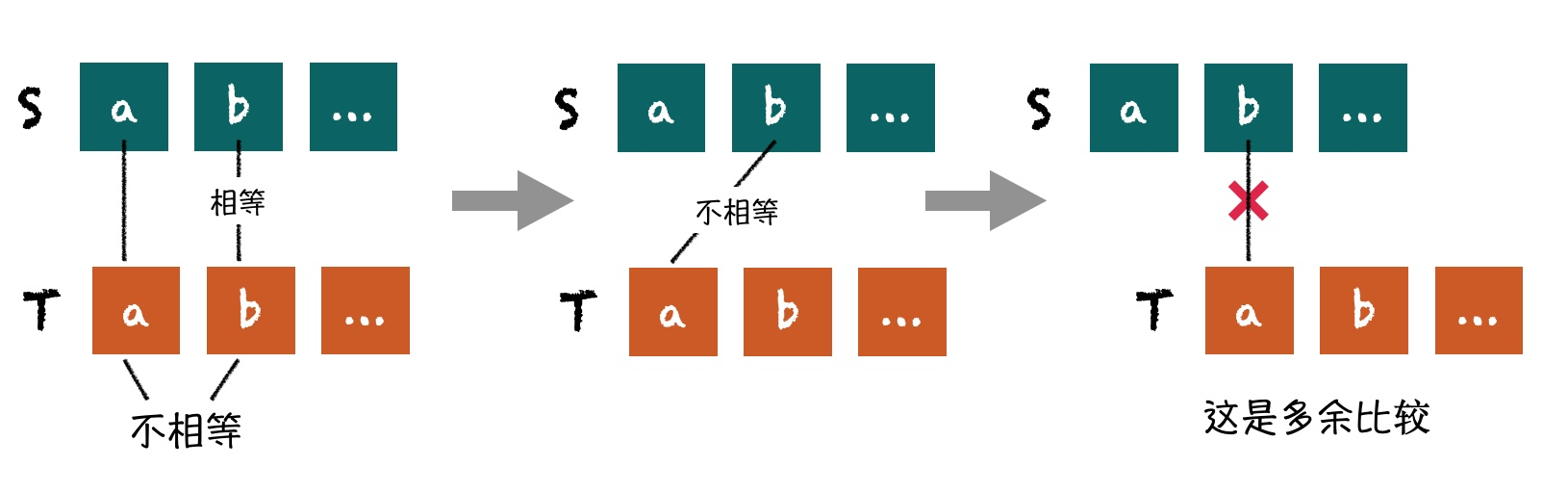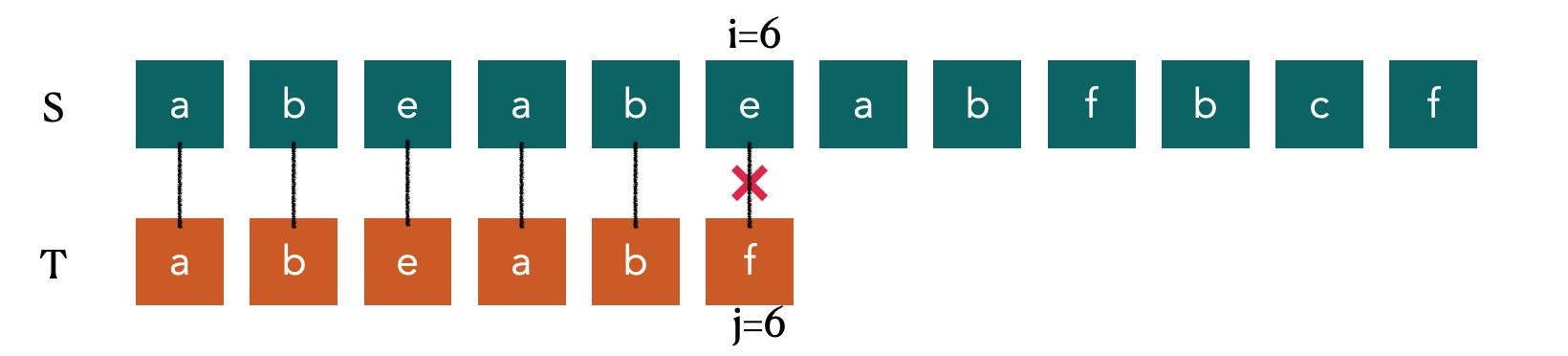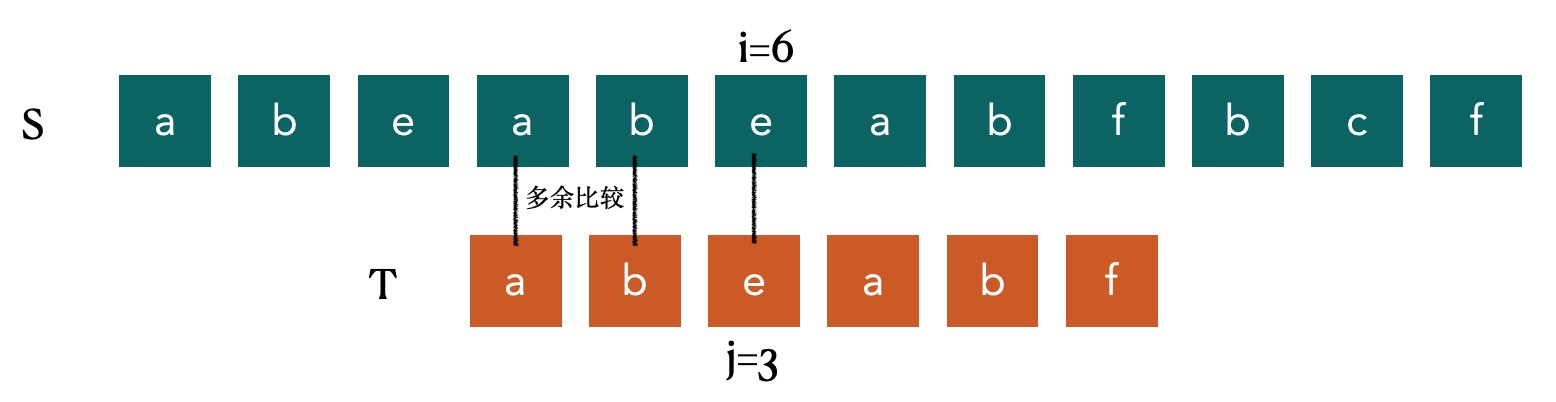### next数组

• 当 比较到`a`不相等的时候，`next = 0`
• 当比较到`b`不想等的时候，它前面的范围内只有一个字符 `a` 那么下次比较还是要跳到`j=1` 所以`next = 1`
• 以此类推，因为`T`串中没有相同的字符所以其每个位置只要发现不同，都要跳转到`j= 1` 的位置执行,所以针对 此`T` 串的 `next = [0,1,1,1,1,1]`

• 当 比较到`a`不相等的时候，`next = 0`

• 当比较到`b`不相等的时候，它前面的范围内只有一个字符 `a` 那么下次比较还是要跳到`j=1` 所以`next = 1`

• 当比较到`c`不相等的时候，它前面的范围内的字符是 `ab` 那么下次比较还是要跳到`j=1` 所以`next = 1`

• 当比较到第二个`a`不相等的时候，它前面的范围内的字符是 `abc` 那么下次比较还是要跳到`j=1` 所以`next = 1`

• 当比较到第二个`b`不相等的时候，它前面的范围内的字符是 `abca`,这里就要注意了，该串有相同的前缀和相同的后缀`a` 那么当我么再跳转的时候，应该是直接比较 `b` 这个位置才对，我们相同的前缀和后缀字符串长度是1，那么我们下一次比较的位置应该在相同前缀或后缀长度的下一个字符处，所以`next = 2`

所以针对此种情况 `next = [0,1,1,1,2]`

``//----KMP 模式匹配算法---//1.通过计算返回子串T的next数组;//注意字符串T中是存储的字符串长度; 真正的字符内容从T开始;void get_next(String T,int *next){    int i,j;    j = 1;    i = 0;    next = 0;    //abcdex    //遍历T模式串, 此时T为模式串T的长度;    //printf("length = %d\n",T);    while (j < T) {        //printf("i = %d j = %d\n",i,j);        if(i ==0 || T[i] == T[j]){            //T[i] 表示后缀的单个字符;            //T[j] 表示前缀的单个字符;            ++i;            ++j;            next[j] = i;            //printf("next[%d]=%d\n",j,next[j]);        }else        {            //如果字符不相同,则i值回溯;            i = next[i];        }    }}``

`next` 数组已经求解出来了，接下来我们就具体实现 `KMP算法`

``//返回子串T在主串S中第pos个字符之后的位置, 如不存在则返回0;int Index_KMP(String S,String T,int pos){    //i 是主串当前位置的下标准,j是模式串当前位置的下标准    int i = pos;    int j = 1;    //定义一个空的next数组;    int next[MAXSIZE];    //对T串进行分析,得到next数组;    get_next(T, next);    count = 0;    //注意: T 和 S 存储的是字符串T与字符串S的长度;    //若i小于S长度并且j小于T的长度是循环继续;    while (i <= S && j <= T) {        //如果两字母相等则继续,并且j++,i++        if(j == 0 || S[i] == T[j]){            i++;            j++;        }else{            //如果不匹配时,j回退到合适的位置,i值不变;            j = next[j];        }    }    if (j > T) {        return i-T;    }else{        return -1;    }}``

### KMP的优化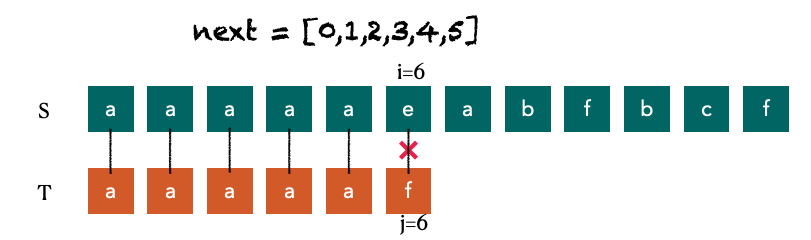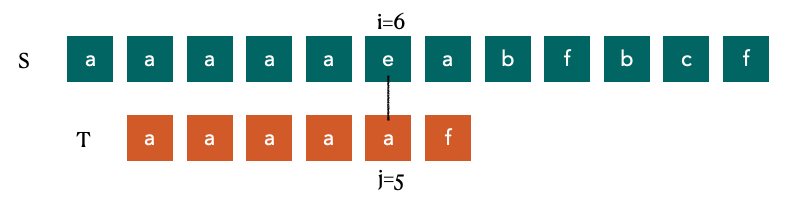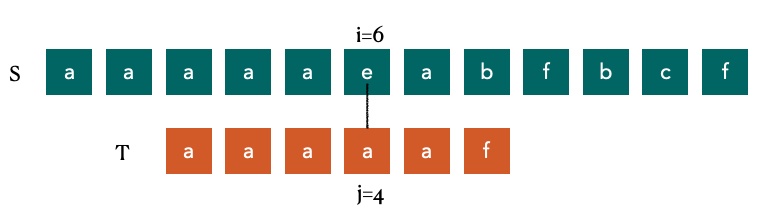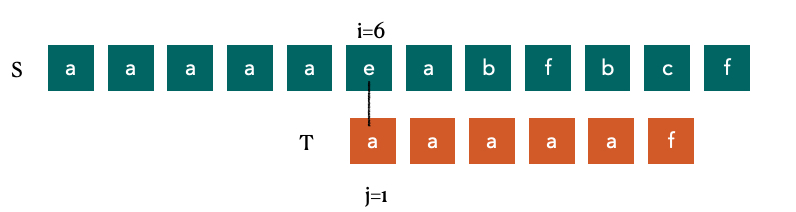``//求模式串T的next函数值修正值并存入nextval数组中;void get_nextVal(String T,int *nextVal){    int i,j;    j = 1;    i = 0;    nextVal = 0;    while (j < T) {        if (i == 0 || T[i] == T[j]) {            ++j;            ++i;            //如果当前字符与前缀不同,则当前的j为nextVal 在i的位置的值            if(T[i] != T[j])                nextVal[j] = i;            else            //如果当前字符与前缀相同,则将前缀的nextVal 值赋值给nextVal 在i的位置                nextVal[j] = nextVal[i];        }else{            i = nextVal[i];        }    }}``

``//返回子串T在主串S中第pos个字符之后的位置, 如不存在则返回0;int Index_KMP2(String S,String T,int pos){    //i 是主串当前位置的下标准,j是模式串当前位置的下标准    int i = pos;    int j = 1;    //定义一个空的next数组;    int next[MAXSIZE];    //对T串进行分析,得到next数组;    get_nextVal(T, next);    count = 0;    //注意: T 和 S 存储的是字符串T与字符串S的长度;    //若i小于S长度并且j小于T的长度是循环继续;    while (i <= S && j <= T) {        //如果两字母相等则继续,并且j++,i++        if(j == 0 || S[i] == T[j]){            i++;            j++;        }else{            //如果不匹配时,j回退到合适的位置,i值不变;            j = next[j];        }    }    if (j > T) {        return i-T;    }else{        return -1;    }}``

## 总结

]]>
<blockquote> <p>有主串 S = “abcacabdc”,模式串 T = “abd”,请查找出模式串在主串第一次出现的位置，注意：主串和模式串均为小写字母切都是合法输入。

## 进制转换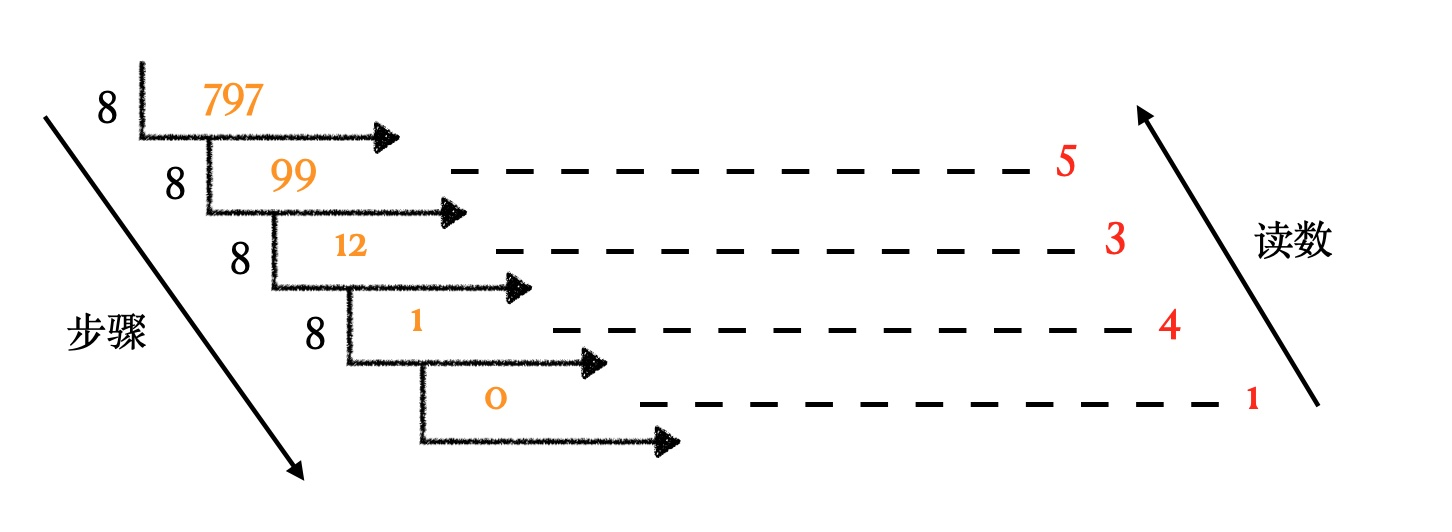``void conversion(int N){    SqStack S;    SElemType e;    //1.初始化一个空栈S    InitStack(&S);    //2.每次取余数直到结果为0为止，把每次的余数进栈    while (N) {        PushData(&S, N%8);        N = N/8;    }    //3.出栈得到我们想要的十进制数据    while (!StackEmpty(S)) {        Pop(&S, &e);        printf("%d\n",e);    }}``

## 每日温度

### 暴力法

``int  *dailyTemperatures_1(int* T, int TSize, int* returnSize){    int *result = (int *)malloc(sizeof(int) * TSize);    *returnSize = TSize;    result[TSize-1] = 0;    for(int i = 0;i < TSize-1;i++)        if(i>0 && T[i] == T[i-1])            result[i] = result[i-1] == 0?0:result[i-1]-1;        else{            for (int j = i+1; j < TSize; j++) {                if(T[j] > T[i]){                    result[i] = j-i;                    break;                }                if (j == TSize-1) {                    result[i] = 0;                }            }        }    return result;}``

### 倒序跳跃法

1. 从右到左遍历. 因为最后一天的气温不会再升高,默认等于0
2. i 从[TSize-2,0]; 从倒数第二天开始遍历比较. 每次减一
3. j 从[i+1,TSize]遍历, j+=result[j],可以利用已经有结果的位置进行跳跃,从而减少遍历次数
1. 若T[i]<T[j],那么result = j - i
2. 若reuslt[j] == 0,则表示后面不会有更大的值,那么当前值就应该也是0
``int  *dailyTemperatures_2(int* T, int TSize, int* returnSize){    int *result = (int *)malloc(sizeof(int) * TSize);    *returnSize = TSize;    result[TSize-1] = 0;    for (int i=TSize-2; i >= 0; i--) {        for (int j = i+1; j < TSize; j+=result[j]) {            if (T[i] < T[j]) {                result[i] = j-i;                break;            }else            {                if (result[j] == 0) {                    result[i] = 0;                    break;                }            }        }    }    return result;}``

### 单调递减栈

1. 初始化一个栈,栈中存储的是元素的索引值index

2. 遍历整个温度数组从[0,TSize]

1. 如果当前的栈为空,则直接入栈
2. 如果当前的元素小于栈顶元素,则入栈
3. 如果栈顶元素<当前元素,则将当前元素索引index-栈顶元素index,计算完毕则将当前栈顶元素移除,将当前元素索引index 存储到栈中; 出栈后,只要栈不为空.继续比较,直到栈顶元素不能满足T[i] > T[stack_index[top-1]]
4. while循环结束后,当前元素也需要入栈
``int* dailyTemperatures_3(int* T, int TSize, int* returnSize) {    int* result = (int*)malloc(sizeof(int)*TSize);    // 用栈记录T的下标。    int* stack_index = malloc(sizeof(int)*TSize);    *returnSize = TSize;    // 栈顶指针。    int top = 0;    int tIndex;    for (int i = 0; i < TSize; i++)        result[i] = 0;    for (int i = 0; i < TSize; i++) {        // 若当前元素大于栈顶元素，栈顶元素出栈。即温度升高了，所求天数为两者下标的差值。        while (top > 0 && T[i] > T[stack_index[top-1]]) {            tIndex = stack_index[top-1];            result[tIndex] = i - tIndex;            top--;        }        // 当前元素入栈。        stack_index[top] = i;        top++;    }    return result;}``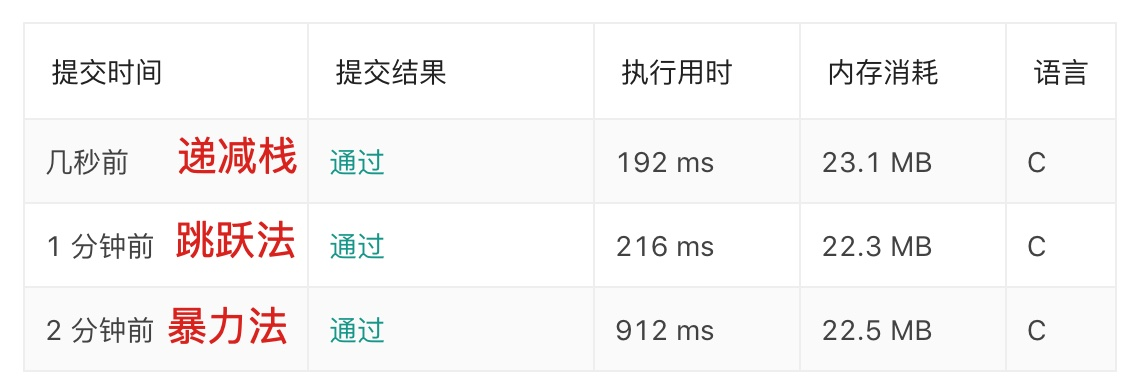## 括号匹配

`` 1// 括号匹配 2Status IsCorrect(char *string) { 3    Stack S; 4    InitStack(&S); 5 6    int i = 0; 7    char temp = string; 8    while (temp) { 9        printf("string is %c \n",temp);10        if (temp == '[') {11            PushData(&S, '[');12        } else if (temp == '(') {13            PushData(&S, '(');14        } else if (temp == ')') {15            char top;16            GetTop(S, &top);17            if (top == '(') {18                Pop(&S, &top);19            } else {20                PushData(&S, ')');21                // return FALSE;22            }23        } else if (temp == ']') {24            char top;25            GetTop(S, &top);26            if (top == '[') {27                Pop(&S, &top);28            } else {29                PushData(&S, ']');30                // return FALSE;31            }32        }33        i ++;34        temp = string[i];35    }3637    return IsEmpty(S)?SUCCESS:FALSE;38}``

## 杨辉三角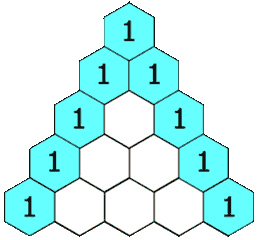``int** generate(int numRows, int* returnSize){    *returnSize = numRows;    int **res = (int **)malloc(sizeof(int*)*numRows);    for (int i = 0; i < numRows; i++) {        res[i] = (int *)malloc(sizeof(int)*(i+1));        res[i] = 1;        res[i][i] = 1;        for (int j = 1; j < i; j++) {            res[i][j] = res[i-1][j] + res[i-1][j-1];        }    }    return res;}``

## 爬楼梯

### 递归

• `n-1` 阶爬 1 个台阶
• `n-2` 阶爬 2个台阶

``int ClimbStairs(int n){    if (n<1)  return 0;    if (n == 1) return 1;    if (n == 2) return 2;    return ClimbStairs(n-1) + ClimbStairs(n-2);}``

### 动态规划

``int ClimbStairs(int n){    if(n==1) return 1;    int temp = n+1;    int *sum = (int *)malloc(sizeof(int) * (temp));    sum = 0;    sum = 1;    sum = 2;    for (int i = 3; i <= n; i++) {        sum[i] = sum[i-1] + sum[i-2];    }    return sum[n];}``

## 字符串编码

s = “3[a]2[bc]”,返回 “aaabcbc”
s = “3[a2[c]]” 返回 “accaccacc”
s = “2[abc]3[cd]ef” 返回 “abcabccdcdcdef”

1. 遍历字符串 S,如果当前字符不为方括号”]” 则入栈stack中
2. 如果当前字符遇到了方括号”]” 则:
1. 首先找到要复制的字符,例如stack=”12[a”,那么我要首先获取字符a;将这个a保存在另外一个栈去tempStack
2. 接下来,要找到需要备份的数量,例如stack=”12[a”,因为出栈过字符”a”,则当前的top指向了”[“,也就是等于2
3. 而12对于字符串是2个字符, 我们要通过遍历找到数字12的top上限/下限的位置索引, 此时上限curTop = 2, 下限通过出栈,top = -1
4. 根据范围[-1,2],读取出12保存到strOfInt 字符串中来, 并且将字符”12\0”,转化成数字12
5. 当前top=-1,将tempStack中的字符a,复制12份入栈到stack中来
6. 为当前的stack扩容, 在stack字符的末尾添加字符结束符合’\0’
``char * decodeString(char * s){    /*.     1.获取字符串长度     2.设置默认栈长度50     3.开辟字符串栈(空间为50)     4.设置栈头指针top = -1;     */    int len = (int)strlen(s);    int stackSize = 50;    char* stack = (char*)malloc(stackSize * sizeof(char));    int top = -1;    //遍历字符串,在没有遇到"]" 之前全部入栈    for (int i = 0; i < len; ++i) {        if (s[i] != ']') {            //优化:如果top到达了栈的上限,则为栈扩容;            if (top == stackSize - 1) {                stack = realloc(stack, (stackSize += 50) * sizeof(char));            }            //将字符入栈stack            stack[++top] = s[i];            printf("#① 没有遇到']'之前# top = %d\n",top);        }        else {            int tempSize = 10;            char* temp = (char*)malloc(tempSize * sizeof(char));            int topOfTemp = -1;            printf("#② 开始获取要复制的字符信息之前 # top = %d\n",top);            //从栈顶位置开始遍历stack,直到"["结束;            //把[a]这个字母a 赋值到temp栈中来;            //简单说,就是将stack中方括号里的字符出栈,复制到temp栈中来;            while (stack[top] != '[') {                //优化:如果topOfTemp到达了栈的上限,则为栈扩容;                if (topOfTemp == tempSize - 1) {                    temp = realloc(temp, (tempSize += 10) * sizeof(char));                }                //temp栈的栈顶指针自增;                ++topOfTemp;                //将stack栈顶字符复制到temp栈中来;                temp[topOfTemp] = stack[top];                //stack出栈,则top栈顶指针递减;                top--;            }            printf("#② 开始获取要复制的字符信息之后 # top = %d\n",top);            //找到倍数数字.strOfInt字符串;            //注意:如果是大于1位的情况就处理            char strOfInt;            //p记录当前的top;            int curTop = top;            printf("#③ 开始获取数字,数字位置上限 # curTop = %d\n",curTop);            //top--的目的是把"["剔除,才能找到数字;            top--;            //遍历stack得出数字            //例如39[a] 就要找到这个数字39.            //p指向当前的top,我就知道上限了; 那么接下来通过循环来找它的数字下限;            //结束条件:栈指针指向为空! stack[top] 不等于数字            while (top != -1 && stack[top] >= '0' && stack[top] <= '9') {                top--;            }            printf("#③ 开始获取数字,数字位置下限 # top = %d\n",top);            //从top-1遍历到p之间, 把stack[top-1,p]之间的数字复制到strOfInt中来;            //39中3和9都是字符. 我们要获取到这2个数字,存储到strOfInt数组            for (int j = top + 1; j < curTop; ++j) {                strOfInt[j - (top + 1)] = stack[j];            }            //为字符串strOfInt数组加一个字符结束后缀'\0'            strOfInt[curTop - (top + 1)] = '\0';            //把strOfInt字符串转换成整数 atoi函数;            //把字母复制strOfInt份到stack中去;            //例如39[a],就需要把复制39份a进去;            int curNum = atoi(strOfInt);            for (int k = 0; k < curNum ; ++k) {                //从-1到topOfTemp 范围内,复制curNum份到stackTop中去;                int kk = topOfTemp;                while (kk != -1) {                    //优化:如果stack到达了栈的上限,则为栈扩容;                    if (top == stackSize - 1) {                        stack = realloc(stack, (stackSize += 50) * sizeof(char));                    }                    //将temp栈的字符复制到stack中;                    //stack[++top] = temp[kk--];                    ++top;                    stack[top] = temp[kk];                    kk--;                }            }            free(temp);            temp = NULL;        }    }    //realloc 动态内存调整;    //void *realloc(void *mem_address, unsigned int newsize);    //构成字符串stack后, 在stack的空间扩容.    char* ans = realloc(stack, (top + 1) * sizeof(char));    ans[++top] = '\0';    //stack 栈不用,则释放;    free(stack);    return ans;}``

## 去除重复字母

1. 创建一个数组保存每个字母出现的次数，很显然只需要创建一个 `26` 个容量的数组即可
2. 遍历字符串，如果栈是空直接入栈，否则进行判断：
1. 如果栈中存在相同的字符，直接对相应字符存在的数量进行减一处理
2. 如果当前字符大于栈顶元素，那么直接入栈
3. 如果当前字符小于栈顶元素，继续判断栈顶元素后面是否还有，如果有那么出栈直到栈顶当前字符大于栈顶元素。
``char *MinString(char *string) {    // 边界判断    if (string == NULL || strlen(string) == 0) return "";    if (strlen(string) == 1) return string;    // 用一个数组保存每个字母出现的次数    int record = {0};    unsigned long len = strlen(string);    int top = -1;    char *stack = (char *)malloc(len*sizeof(char)+1);    stack = memset(stack, 0, len*sizeof(char)+1);    for (int i = 0; i < len; i++) {        record[string[i]-'a']++;    }    for (int i = 0; i < len; i++) {        // 判断当前字符是否已经在栈中        int exist = 0;        for (int j = 0; j <= top; j ++) {            if (stack[j] == string[i]) {                exist = 1;            }        }        if (exist == 1) {// 如果存在直接对数组中相应字母数量减一            record[string[i]-'a']--;        } else {            // 如果栈为空或者栈顶小于当前元素直接入栈，并减少数量            if (top == -1 || stack[top] < string[i]) {                top++;                stack[top] = string[i];                record[string[i]-'a']--;            } else {                // 否则一直出栈直到栈顶元素小于当前元素                while (top>=0 && record[stack[top]-'a'] > 0 && stack[top] > string[i]) {                    top--;                }                // 入栈新元素，并减少数量                stack[++top] = string[i];                record[string[i]-'a']--;            }        }    }    // 字符串末尾补\0    stack[++top] = '\0';    return stack;}``

## 总结

]]>
<p>这篇文章主要罗列一些常见的算法题，涉及到栈的使用等。

## 顺序存储队列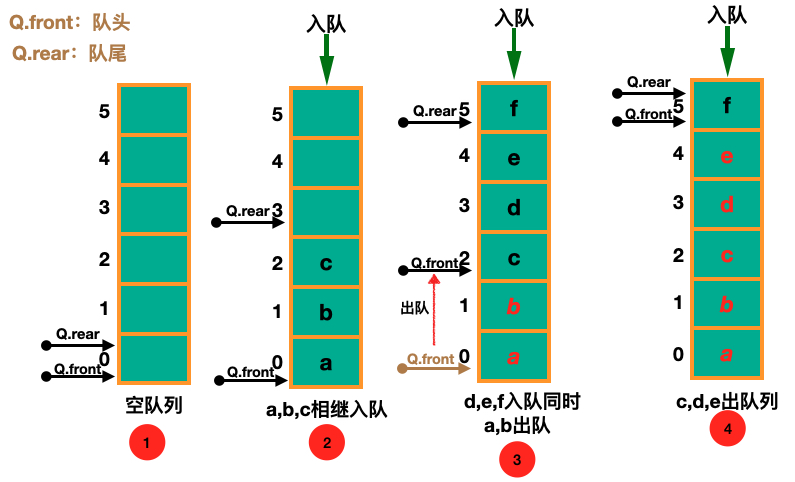• ①图：空队列的时候队头和队尾都指向默认的一个节点
• ②图：入队的时候只需要挪动队尾的位置即可
• ③图：出队同入队只需要挪动队头的位置即可
• ③④图：当队尾达到存储空间尾部即代表队列已满

### 循环队列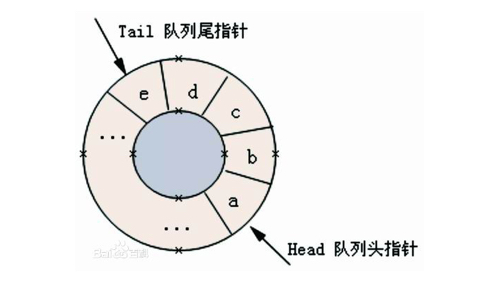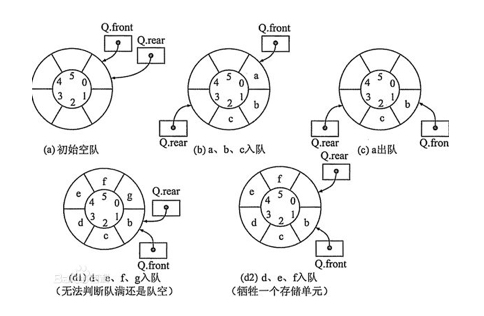### 循环队列的线性结构

``typedef struct Node {    Data data[MAXSIZE];    int rear;    int front;} Queue;``

``// 初始化一个空队列Status InitQueue(Queue *Q) {    Q->rear = 0;    Q->rear = 0;    return SUCCESS;}// 清空一个队列Status ClearQueue(Queue *Q) {    Q->rear = 0;    Q->front = 0;    return SUCCESS;}// 是否为空队列Status IsEmpty(Queue Q) {    return Q.rear == Q.front ? TRUE:FALSE;}// 队列长度int Length(Queue Q) {    return (Q.rear-Q.front+MAXSIZE)%MAXSIZE;}// 获取头节点Status GetHead(Queue Q,Data *data) {    if (IsEmpty(Q)) return ERROR;    *data = Q.data[Q.front];    return SUCCESS;}// 入丢列Status QueueEnter(Queue *Q,Data data) {    if ((Q->rear+1)%MAXSIZE == Q->front) return ERROR;    Q->data[Q->rear] = data;    // rear 指针向后移一位，如果到达最后则转到数组头部    Q->rear = (Q->rear+1)%MAXSIZE;    return SUCCESS;}// 出队列Status QueuePop(Queue *Q, Data *data) {    if (IsEmpty(*Q)) return ERROR;    *data = Q->data[Q->front];    // front 同上面出队列的rear一样    Q->front = (Q->front+1)%MAXSIZE;    return SUCCESS;}// 遍历队列Status QueueTraverse(Queue Q) {    if (IsEmpty(Q)) return ERROR;    int i = Q.front;    while ((i+Q.front) != Q.rear) {        printf("%d   ",Q.data[i]);        i = (i+1)%MAXSIZE;    }    printf("\n");    return SUCCESS;}``

• 我们牺牲了一个节点，所以在于队列是否满的状态判断中要针对 +1 进行操作
• 循环队列的循环入队以及出队的过程中涉及到 rearfront 位置的变迁，这也涉及到一个环状计算的问题，这里一般使用 %(模) 运算进行处理

## 链式存储队列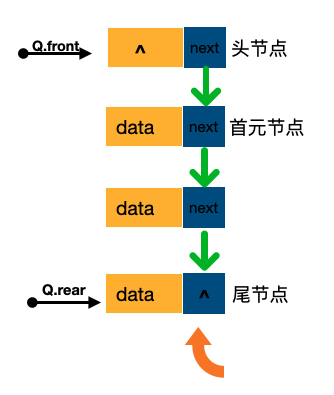``typedef int Status;typedef int Data;typedef struct Node {    Data data;    struct Node *next;} Node;typedef struct {    Node *front;    Node *rear;} Queue;``

``// 初始化队列Status InitQueue(Queue *Q) {    Q->front = Q->rear = (Node *)malloc(sizeof(Node));    if (!Q->front) return ERROR;    Q->front->next = NULL;    return SUCCESS;}// 销毁队列Status DestroyQueue(Queue *Q) {    // 遍历链表进行销毁    while (Q->front) {        Node *temp = = Q->front;        Q->front = Q->front->next;        free(temp);    }    return SUCCESS;}// 将队列置空Status ClearQueue(Queue *Q) {    Node *target = Q->front->next;    while (target) {        Node *temp = target;        target = target->next;        free(temp);    }    Q->front->next = Q->rear->next = NULL;    return SUCCESS;}// 判断队列是否为空Status IsEmpty(Queue Q) {    return (Q.rear == Q.front)?TRUE:FALSE;}// 获取队列的长度int GetLength(Queue Q) {    Node *target = Q.front->next;    int i=0;    while (target) {        i++;        target = target->next;    }    return i;}// 入队Status QueueEnter(Queue *Q,Data data) {    Node *node = (Node *)malloc(sizeof(node));    if (!node) return ERROR;    node->data = data;    node->next = NULL;    Q->rear->next = node;    // 修改队尾指针    Q->rear = node;    return SUCCESS;}// 出队Status QueuePop(Queue *Q,Data *data) {    if (Q->front == Q->rear) return ERROR;    Node *temp = Q->front->next;    *data = temp->data;    Q->front->next = temp->next;    // 如果只有一个节点，就要移动尾指针    if (temp == Q->rear) {        Q->rear = Q->front;    }    free(temp);    return SUCCESS;}// 获取队头元素Status GetHead(Queue Q,Data *data) {    if (Q.front == Q.rear) return ERROR;    *data = Q.front->next->data;    return SUCCESS;}// 遍历队列Status QueueTraverse(Queue Q) {    Node *target = Q.front->next;    printf("打印队列是：")    while (target) {        printf("%d ",target->data);        target = target->next;    }    printf("\n");    return SUCCESS;}``

]]>
<p>之前分享了一篇关于栈这种数据结构的逻辑和实现，这篇文章我们看看队列这种数据结构是一种什么样的结构以及如何从顺序存储和链式存储去实现这么一个结构。</p> <p>队列也是一种线性数据结构，跟栈的结构差不多，唯一不同的的就是 <strong>栈是先进后出</strong> 而 <strong>队列是先进先出</strong> 也就是通常所说的 <strong>FIFO</strong> : <strong>first in first out !</strong>

## 栈结构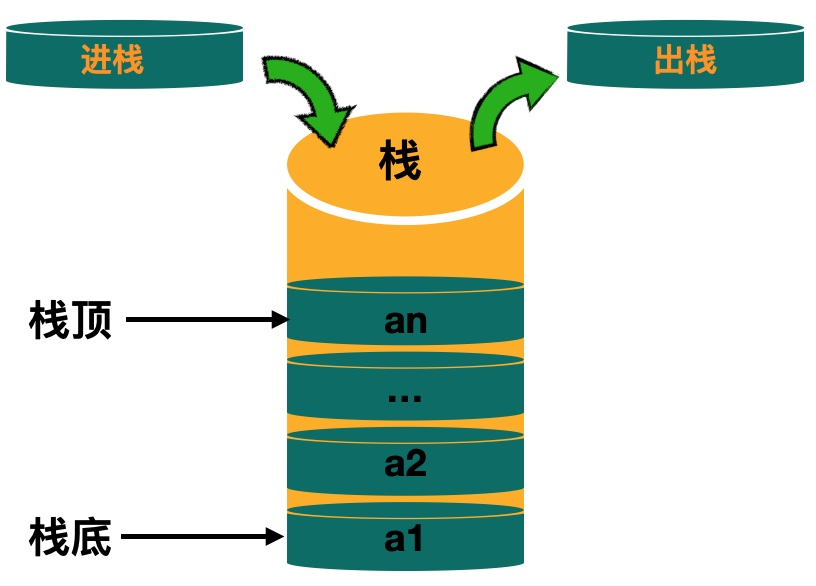## 顺序栈

``typedef int Status;typedef int Data;typedef struct {    Data data[MAXSIZE];    int top;}Stack;``

``// 初始化空栈Status InitStack (Stack *S) {    S->top = -1;    return SUCCESS;}// 清空栈Status ClearStack (Stack *S) {    S->top = -1;    return SUCCESS;}// 判断是否为空栈bool IsEmpty(Stack S) {    if (S.top == -1) {        return true;    } else {        return false;    }}// 返回栈长度int StackLength(Stack s) {    return s.top+1;}// 获取栈顶元素Status GetStackTop(Stack S,Data *data) {    if (S.top == -1)return ERROR;    *data = S.data[S.top];    return SUCCESS;}// 入栈Status PushData(Stack *S,Data data) {    if (S->top == MAXSIZE-1) return ERROR;    int top = S->top+1;    S->data[top] = data;    S->top++;    return SUCCESS;}// 出栈Status Pop(Stack *S,Data *data) {    if (S->top == -1) return ERROR;    *data = S->data[S->top];    S->top--;    return SUCCESS;}// 从栈底到栈顶打印栈Status PrintStack(Stack S) {    if (S.top == -1) {        printf("空栈 \n");        return ERROR;    }    for (int i = 0; i <= S.top; i ++) {        printf("%d--",S.data[i]);    }    printf("\n");    return SUCCESS;}``

``int main(int argc, const char * argv[]) {    // insert code here...    Stack S;    InitStack(&S);    for (int i = 0; i < 10; i ++) {        PushData(&S, i);    }    PrintStack(S);    Data data;    Pop(&S, &data);    PrintStack(S);    printf("出栈的数据是： %d \n",data);    GetStackTop(S, &data);    printf("栈顶的数据是： %d \n",data);    return 0;}------------------------打印数据栈中数据是：0--1--2--3--4--5--6--7--8--9--栈中数据是：0--1--2--3--4--5--6--7--8--出栈的数据是： 9 栈顶的数据是： 8 ``

• 线性栈的置空不用清空每个位置的数据，只需要修改 top 即可
• 返回栈的长度不用判断 top = -1 的情况，因为 -1+1 = 0 其最终结果是一样的

## 链式栈

``// 栈中每个位置的数据typedef struct Node {    Data data;    Node *next;} Node;// 栈的结构typedef struct {    Node *top; // 栈顶节点    int count; // 栈的数据量} Stack;``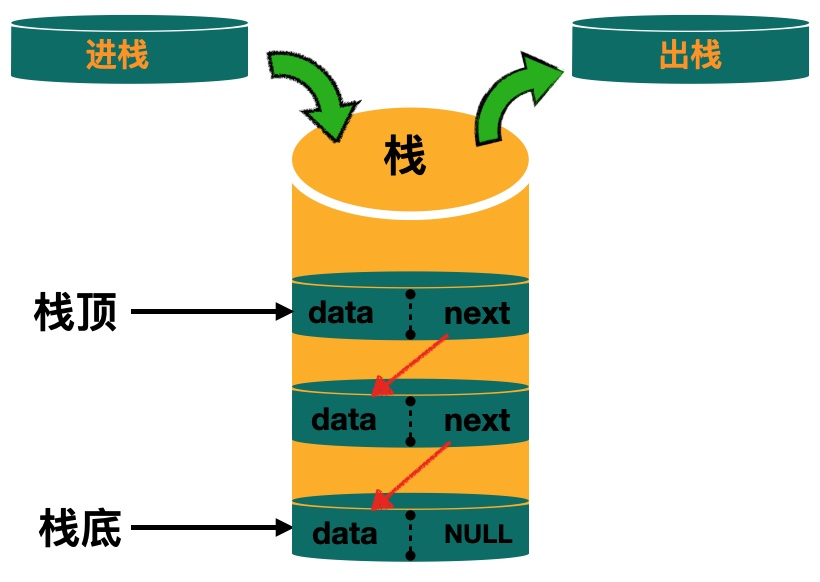``// 创建一个空栈Status InitStack(Stack *S) {    S->top = (Node *)malloc(sizeof(Node));    if (!S->top) return ERROR;    S->top = NULL;    S->count = 0;    return SUCCESS;}// 入栈Status PushData(Stack *S, Data data) {    if (!S) return ERROR;    Node *newTop = (Node *)malloc(sizeof(Node));    newTop->data = data;    newTop->next = S->top;    S->top = newTop;    S->count++;    return SUCCESS;}// 出栈Status Pop(Stack *S,Data *data) {    if (!S) return ERROR;    Node *temp = S->top;    S->top = temp->next;    S->count--;    *data = temp->data;    free(temp);    return SUCCESS;}// 获取栈顶元素Status GetTop(Stack S,Data *data) {    if (S.count == 0) return ERROR;    *data = S.top->data;    return SUCCESS;}// 清空栈Status ClearStack(Stack *S) {    Node *temp = S->top;    while (temp) {        Node *target = temp;        temp = temp->next;        free(target);    }    S->top = NULL;    S->count = 0;    return SUCCESS;}// 从栈顶到栈底打印Status PrintStack(Stack S) {    if (S.count <= 0) return ERROR;    printf("栈的数据从顶到底是：");    Node *temp = S.top;    while (temp) {        printf("%d - ",temp->data);        temp = temp->next;    }    printf("\n");    return SUCCESS;}``

``int main(int argc, const char * argv[]) {    // insert code here...    Stack S;    InitStack(&S);    if (InitStack(&S) == SUCCESS) {        for (int i = 1; i < 10; i++) {            PushData(&S, i);        }    }    PrintStack(S);    Data data;    Pop(&S, &data);    PrintStack(S);    printf("出栈的元素是: %d \n",data);    GetTop(S, &data);    printf("栈顶的元素是: %d \n",data);    return 0;}--------------------- 打印数据栈的数据从顶到底是：9 - 8 - 7 - 6 - 5 - 4 - 3 - 2 - 1 - 栈的数据从顶到底是：8 - 7 - 6 - 5 - 4 - 3 - 2 - 1 - 出栈的元素是: 9 栈顶的元素是: 8 ``

## 总结

]]>
<p>我们都知道函数都是存放在栈上，由系统帮我们管理，那么栈到底是一种什么样的数据结构呢？它是如何管理数据的？ 日常开发中我们或许并没有直接的用上栈这种数据结构，但是它却能帮我们解决一些很棘手的问题，这篇文章主要分享一下个人对栈的理解以及如何用 <strong>c</strong>去实现一个栈的结构

## 合并有序链表

``Status MergeList(LinkList *La,LinkList *Lb,LinkList *Lc) {    // 找到首元节点，依次遍历    Node *pa = (*La)->next;    Node *pb = (*Lb)->next;    // 由于不能开辟新的空间，我们借用La的空间    Node *pc = *La;    // pc是一个局部变量 保存的是Lc的尾节点，每次都指向两个值中的最小值，如果相等则保持其一，删除释放另外一个    while (pa && pb) {        if (pa->data < pb->data) {            pc->next = pa;            pc = pa;// 这地方相当于pc = pc->next 也就是说pc指针也要后移            pa = pa->next;        } else if (pa->data > pb->data) {            pc->next = pb;            pc = pb;            pb = pb->next;        } else {            Node *temp = pb;            pc->next = pa;            pc = pa;            pa = pa->next;            pb = pb->next;            free(temp);        }    }    // 最后多余的数据一定是后续最大的    pc->next = pa?pa:pb;    *Lc = *La;    return SUCCESS;}``

``int main(int argc, const char * argv[]) {    // insert code here...    printf("Hello, World!\n");    // 算法题1    LinkList la,lb,lc;    InitList(&la);    InitList(&lb);    InitList(&lc);    for (int i = 6; i > 0; i --) {        ListInsert(&la, 1, i);    }    printf("打印la ：\n");    ListTraverse(la);    for (int i = 10; i > 2; i-=2) {        ListInsert(&lb, 1, i);    }    printf("打印lb ：\n");    ListTraverse(lb);    MergeList(&la, &lb, &lc);    printf("打印lc ：\n");    ListTraverse(lc);    return 0;}-----------------------------打印结果Hello, World!打印la ：1  2  3  4  5  6  打印lb ：4  6  8  10  打印lc ：1  2  3  4  5  6  8  10  Program ended with exit code: 0``

## 求交集

``Status Intersection(LinkList *La,LinkList *Lb,LinkList *Lc) {    Node *pa = (*La)->next;    Node *pb = (*Lb)->next;    Node *pc = *La;    Node *temp;    while (pa && pb) {        // 每次碰到小的就过滤掉并释放        if (pa->data < pb->data) {            temp = pa;            pa = pa->next;            free(temp);        } else if (pa->data > pb->data) {            temp = pb;            pb = pb->next;            free(temp);        } else {            // 碰到相等的保留其中一个并释放另外一个            pc->next = pa;            pc = pc->next;            pa = pa->next;            temp = pb;            pb = pb->next;            free(temp);        }    }    // 要额外判断多余的情况    while (pa) {        temp = pa;        pa = pa->next;        free(temp);    }    while (pb) {        temp = pb;        pb = pb->next;        free(temp);    }    *Lc = *La;    return SUCCESS;}``

``int main(int argc, const char * argv[]) {    LinkList la,lb,lc;    InitList(&la);    InitList(&lb);    InitList(&lc);    for (int i = 6; i > 0; i --) {        ListInsert(&la, 1, i);    }    printf("打印la ：\n");    ListTraverse(la);    for (int i = 10; i > 2; i-=2) {        ListInsert(&lb, 1, i);    }    printf("打印lb ：\n");    ListTraverse(lb);    Intersection(&la, &lb, &lc);    printf("打印lc ：\n");    ListTraverse(lc);    return 0;}--------------------------- 打印结果打印la ：1  2  3  4  5  6  打印lb ：4  6  8  10  打印 lc ：4  6  ``

## 链表倒序

``void Inverse(LinkList *L) {    //  头插法每次要插入的节点，初始是首元节点    Node *target = (*L)->next;    Node *tNext;    // 为了让链表的尾结点指向NULL    (*L)->next = NULL;    while (target) {        // 每次让tNext保存target之后的数据        tNext = target->next;        target->next = (*L)->next;        (*L)->next = target;        target = tNext;    }}``